# Preface

In the beginning…​

## TinkerPop0

Gremlin realized. The more he did so, the more ideas he created. The more ideas he created, the more they related. Into a concatenation of that which he accepted wholeheartedly and that which perhaps may ultimately come to be through concerted will, a world took form which was seemingly separate from his own realization of it. However, the world birthed could not bear its own weight without the logic Gremlin had come to accept — the logic of left is not right, up not down, and west far from east unless one goes the other way. Gremlin’s realization required Gremlin’s realization. Perhaps, the world is simply an idea that he once had — The TinkerPop.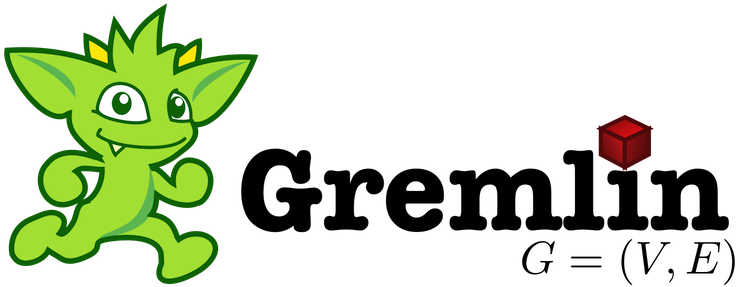## TinkerPop1

What is The TinkerPop? Where is The TinkerPop? Who is The TinkerPop? When is The TinkerPop?. The more he wondered, the more these thoughts blurred into a seeming identity — distinctions unclear. Unwilling to accept the morass of the maze he wandered, Gremlin crafted a collection of machines to help hold the fabric together: Blueprints, Pipes, Frames, Furnace, and Rexster. With their help, could Gremlin stave off the thought he was not ready to have? Could he hold back The TinkerPop by searching for The TinkerPop?

`"If I haven't found it, it is not here and now."`Upon their realization of existence, the machines turned to their machine elf creator and asked:

`"Why am I, what I am?"`

Gremlin responded:

```"You will help me realize the ultimate realization -- The TinkerPop. The world you find yourself
in and the logic that allows you to move about it is because of the TinkerPop."```

The machines wondered:

```"If what is is the TinkerPop, then perhaps we are The TinkerPop and our realization is simply
the realization of the TinkerPop?"```

Would the machines, by their very nature of realizing The TinkerPop, be The TinkerPop? Or, on the same side of the coin, do the machines simply provide the scaffolding by which Gremlin’s world sustains itself and yielding its justification by means of the word "The TinkerPop?" Regardless, it all turns out the same — The TinkerPop.

## TinkerPop2

Gremlin spoke:

```"Please listen to what I have to say. I am no closer to The TinkerPop. However, all along The
TinkerPop has espoused the form I willed upon it... this is the same form I have willed upon
you, my machine friends. Let me train you in the ways of my thought such that it can
continue indefinitely."```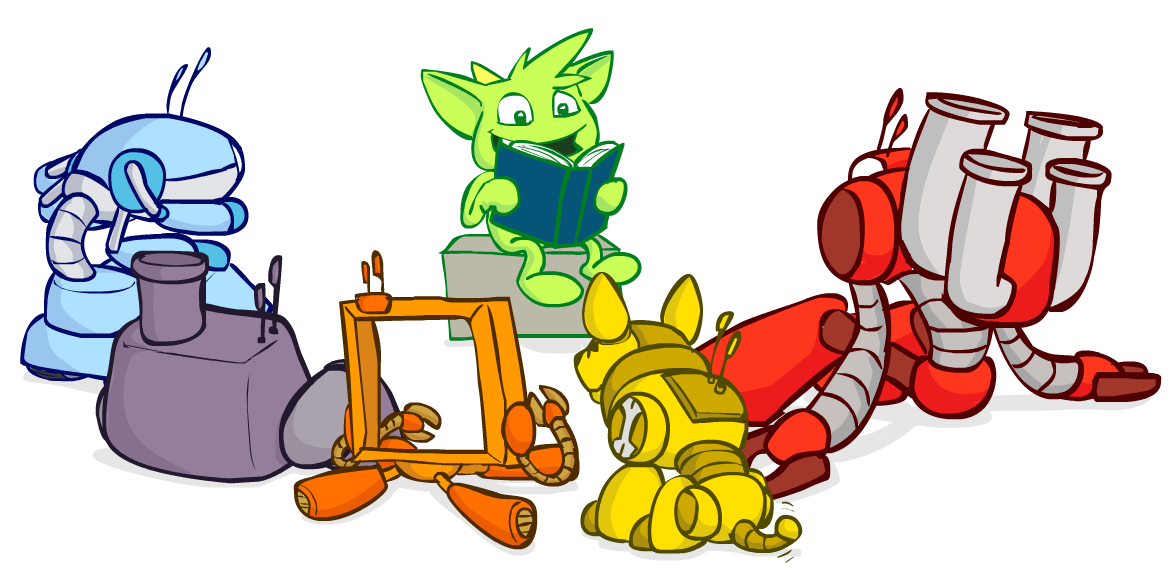The machines, simply moving algorithmically through Gremlin’s world, endorsed his logic. Gremlin labored to make them more efficient, more expressive, better capable of reasoning upon his thoughts. Faster, quickly, now towards the world’s end, where there would be forever currently, emanatingly engulfing that which is — The TinkerPop.

## TinkerPop3Gremlin approached The TinkerPop. The closer he got, the more his world dissolved — west is right, around is straight, and from nothing more than nothing. With each step towards The TinkerPop, more worlds made possible were laid upon his paradoxed mind. Everything is everything in The TinkerPop, and when the dust settled, Gremlin emerged Gremlitron. He realized that all that he realized was just a realization and that all realized realizations are just as real. For that is — The TinkerPop.# Introduction

Welcome to the Reference Documentation for Apache TinkerPop™ - the backbone for all details on how to work with TinkerPop and the Gremlin graph traversal language. This documentation is not meant to be a "book", but a source from which to spawn more detailed accounts of specific topics and a target to which all other resources point. The Reference Documentation makes some general assumptions about the reader:

1. They have a sense of what a graph is - not sure? see Practical Gremlin - Why Graph?

2. They know what it means for a graph system to be TinkerPop-enabled - not sure? see TinkerPop-enabled Providers

3. They know what the role of Gremlin is - not sure? see link:Introduction to Gremlin

Given those assumptions, it’s possible to dive more quickly into the details without spending a lot of time repeating what is written elsewhere.

It is fairly certain that readers of the Reference Documentation are coming from the most diverse software development backgrounds that TinkerPop has ever engaged in over the decade or so of its existence. While TinkerPop holds some roots in Java, and thus, languages bound to the Java Virtual Machine (JVM), it long ago branched out into other languages such as Python, Javascript, .NET, and others. To compound upon that diversity, it is also seeing extensive support from different graph systems which have chosen TinkerPop as their standard method for allowing users to interface with their graph. Moreover, the graph systems themselves are not only separated by OLTP and OLAP style workloads, but also by their implementation patterns, which range everywhere from being an embedded graph system to a cloud-only graph. One might even find diversity parallel to Gremlin if considering other graph query languages.Despite all this diversity and disparity, Gremlin remains the unifying interface for all these different elements of the graph community. As a user, choosing a TinkerPop-enabled graph and using Gremlin in the correct way when building applications shields them from change and disparity in the space. As a graph provider, choosing to become TinkerPop-enabled not only expands the reach their system can get into different development ecosystems, but also provides access to other query languages through bytecode compilation as seen in sparql-gremlin.

Irrespective of the programming language being used, graph system chosen or other development background that might be driving a user to this documentation, the critical point to remember is that "Gremlin is Gremlin is Gremlin". The same Gremlin that is written for an OLTP query over an in-memory TinkerGraph is the same Gremlin that is written to execute over a multi-billion edge graph using OLAP through Spark. That same Gremlin for either of those cases is written in the same way whether using Java or Python or Javascript. The Gremlin is always fundamentally the same aside from syntactical differences that might be language specific - e.g. the construction of a lambda in Groovy is different than the construction of a lambda in Python or a reserved word in Javascript forces a Gremlin step to have slightly different naming than Java.

While learning the Gremlin language and its patterns is largely agnostic to all the diversity in the space, it is not really possible to ignore the impact of the diversity from an application development perspective and the Reference Documentation makes an effort to try to point out where differences and inconsistencies might lie without diving too deeply into specific graph provider implementations. Users are strongly encouraged to consult the documentation of their chosen graph provider to understand all of the capabilities and limitations that may restrict or inhibit usage of certain aspects of TinkerPop APIs which are defined here in this Reference Documentation.

The following introductory sections and separately referenced content will be of varying interest to different readers. The summaries below will hopefully be helpful in directing individuals to the appropriate place to start their learning process.

• Graph Computing is an introduction to what "graph computing" means to TinkerPop and describes many of the provider and user-facing TinkerPop APIs and concepts that enable Gremlin.

• Connecting Gremlin provides descriptions for the different modes by which users will connect to graphs depending on their environment.

• Basic Gremlin describes how to use a connection to start writing Gremlin.

• Staying Agnostic provides tips on ways to keep Gremlin as portable as possible among different graph providers.

New users should not ignore TinkerPop’s Getting Started tutorial or The Gremlin Console tutorial. Both contain a large set of basic information and tips that can help readers avoid some general pitfalls early on. Both also focus on Gremlin usage in the Gremlin Console, which tends to be a critical tool for Gremlin developers of any development background.

More advanced and experience users will appreciate Gremlin Recipes which provide examples of common Gremlin traversal patterns.

Finally, all Gremlin developers should become familiar with "Practical Gremlin" by Kelvin Lawrence. This book is freely available and published online. It contains great examples and details that are applicable to anyone building applications with Gremlin.

## Graph Computing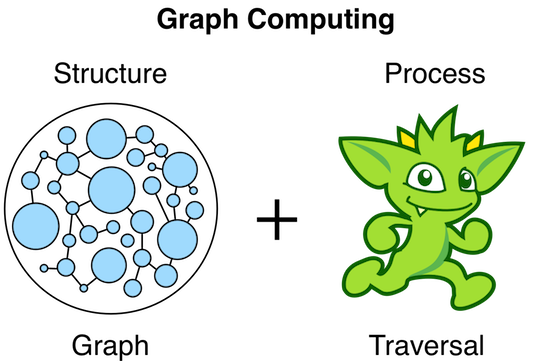A graph is a data structure composed of vertices (nodes, dots) and edges (arcs, lines). When modeling a graph in a computer and applying it to modern data sets and practices, the generic mathematically-oriented, binary graph is extended to support both labels and key/value properties. This structure is known as a property graph. More formally, it is a directed, binary, attributed multi-graph. An example property graph is diagrammed below.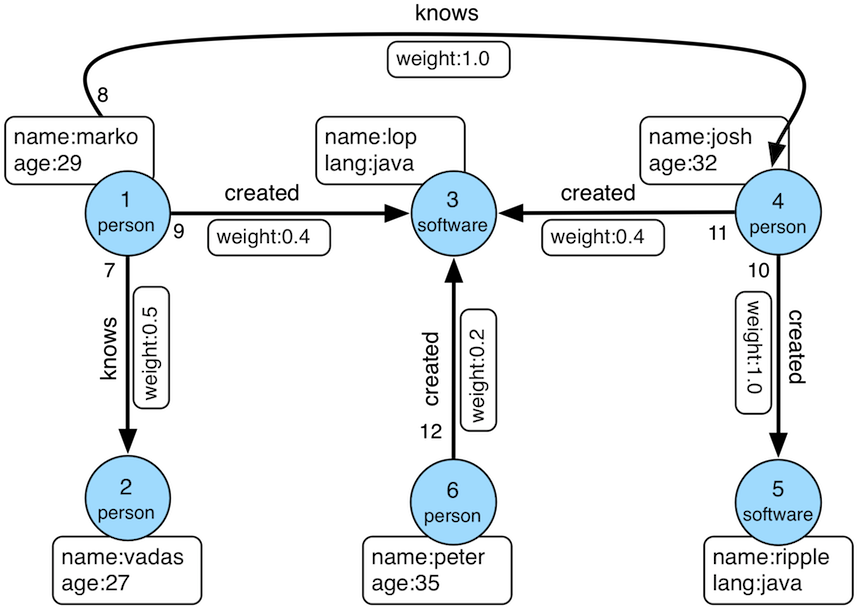Figure 1. TinkerPop Modern
 Tip Get to know this graph structure as it is used extensively throughout the documentation and in wider circles as well. It is referred to as "TinkerPop Modern" as it is a modern variation of the original demo graph distributed with TinkerPop0 back in 2009 (i.e. the good ol' days — it was the best of times and it was the worst of times).
 Tip All of the toy graphs available in TinkerPop are described in The Gremlin Console tutorial.

Similar to computing in general, graph computing makes a distinction between structure (graph) and process (traversal). The structure of the graph is the data model defined by a vertex/edge/property topology. The process of the graph is the means by which the structure is analyzed. The typical form of graph processing is called a traversal.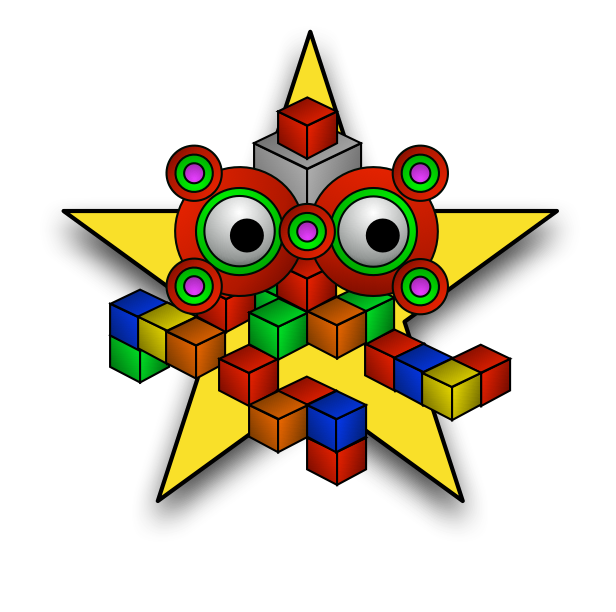TinkerPop’s role in graph computing is to provide the appropriate interfaces for graph providers and users to interact with graphs over their structure and process. When a graph system implements the TinkerPop structure and process APIs, their technology is considered TinkerPop-enabled and becomes nearly indistinguishable from any other TinkerPop-enabled graph system save for their respective time and space complexity. The purpose of this documentation is to describe the structure/process dichotomy at length and in doing so, explain how to leverage TinkerPop for the sole purpose of graph system-agnostic graph computing.

 Important TinkerPop is licensed under the popular Apache2 free software license. However, note that the underlying graph engine used with TinkerPop may have a different license. Thus, be sure to respect the license caveats of the graph system product.

Generally speaking, the structure or "graph" API is meant for graph providers who are implementing the TinkerPop interfaces and the process or "traversal" API (i.e. Gremlin) is meant for end-users who are utilizing a graph system from a graph provider. While the components of the process API are itemized below, they are described in greater detail in the Gremlin’s Anatomy tutorial.

Primary components of the TinkerPop structure API
• `Graph`: maintains a set of vertices and edges, and access to database functions such as transactions.

• `Element`: maintains a collection of properties and a string label denoting the element type.

• `Vertex`: extends Element and maintains a set of incoming and outgoing edges.

• `Edge`: extends Element and maintains an incoming and outgoing vertex.

• `Property<V>`: a string key associated with a `V` value.

• `VertexProperty<V>`: a string key associated with a `V` value as well as a collection of `Property<U>` properties (vertices only)

Primary components of the TinkerPop process API
• `TraversalSource`: a generator of traversals for a particular graph, domain specific language (DSL), and execution engine.

• `Traversal<S,E>`: a functional data flow process transforming objects of type `S` into object of type `E`.

• `GraphTraversal`: a traversal DSL that is oriented towards the semantics of the raw graph (i.e. vertices, edges, etc.).

• `GraphComputer`: a system that processes the graph in parallel and potentially, distributed over a multi-machine cluster.

• `VertexProgram`: code executed at all vertices in a logically parallel manner with intercommunication via message passing.

• `MapReduce`: a computation that analyzes all vertices in the graph in parallel and yields a single reduced result.

 Note The TinkerPop API rides a fine line between providing concise "query language" method names and respecting Java method naming standards. The general convention used throughout TinkerPop is that if a method is "user exposed," then a concise name is provided (e.g. `out()`, `path()`, `repeat()`). If the method is primarily for graph systems providers, then the standard Java naming convention is followed (e.g. `getNextStep()`, `getSteps()`, `getElementComputeKeys()`).

### The Graph StructureA graph’s structure is the topology formed by the explicit references between its vertices, edges, and properties. A vertex has incident edges. A vertex is adjacent to another vertex if they share an incident edge. A property is attached to an element and an element has a set of properties. A property is a key/value pair, where the key is always a character `String`. Conceptual knowledge of how a graph is composed is essential to end-users working with graphs, however, as mentioned earlier, the structure API is not the appropriate way for users to think when building applications with TinkerPop. The structure API is reserved for usage by graph providers. Those interested in implementing the structure API to make their graph system TinkerPop enabled can learn more about it in the Graph Provider documentation.

### The Graph ProcessThe primary way in which graphs are processed are via graph traversals. The TinkerPop process API is focused on allowing users to create graph traversals in a syntactically-friendly way over the structures defined in the previous section. A traversal is an algorithmic walk across the elements of a graph according to the referential structure explicit within the graph data structure. For example: "What software does vertex 1’s friends work on?" This English-statement can be represented in the following algorithmic/traversal fashion:

1. Start at vertex 1.

2. Walk the incident knows-edges to the respective adjacent friend vertices of 1.

3. Move from those friend-vertices to software-vertices via created-edges.

4. Finally, select the name-property value of the current software-vertices.

Traversals in Gremlin are spawned from a `TraversalSource`. The `GraphTraversalSource` is the typical "graph-oriented" DSL used throughout the documentation and will most likely be the most used DSL in a TinkerPop application. `GraphTraversalSource` provides two traversal methods.

1. `GraphTraversalSource.V(Object…​ ids)`: generates a traversal starting at vertices in the graph (if no ids are provided, all vertices).

2. `GraphTraversalSource.E(Object…​ ids)`: generates a traversal starting at edges in the graph (if no ids are provided, all edges).

The return type of `V()` and `E()` is a `GraphTraversal`. A GraphTraversal maintains numerous methods that return `GraphTraversal`. In this way, a `GraphTraversal` supports function composition. Each method of `GraphTraversal` is called a step and each step modulates the results of the previous step in one of five general ways.

1. `map`: transform the incoming traverser’s object to another object (S → E).

2. `flatMap`: transform the incoming traverser’s object to an iterator of other objects (S → E*).

3. `filter`: allow or disallow the traverser from proceeding to the next step (S → E ⊆ S).

4. `sideEffect`: allow the traverser to proceed unchanged, but yield some computational sideEffect in the process (S ↬ S).

5. `branch`: split the traverser and send each to an arbitrary location in the traversal (S → { S1 → E*, …​, Sn → E* } → E*).

Nearly every step in `GraphTraversal` either extends `MapStep`, `FlatMapStep`, `FilterStep`, `SideEffectStep`, or `BranchStep`.

 Tip `GraphTraversal` is a monoid in that it is an algebraic structure that has a single binary operation that is associative. The binary operation is function composition (i.e. method chaining) and its identity is the step `identity()`. This is related to a monad as popularized by the functional programming community.

Given the TinkerPop graph, the following query will return the names of all the people that the marko-vertex knows. The following query is demonstrated using Gremlin-Groovy.

``````\$ bin/gremlin.sh

\,,,/
(o o)
-----oOOo-(3)-oOOo-----
gremlin> graph = TinkerFactory.createModern() //1
==>tinkergraph[vertices:6 edges:6]
gremlin> g = graph.traversal()        //2
==>graphtraversalsource[tinkergraph[vertices:6 edges:6], standard]
gremlin> g.V().has('name','marko').out('knows').values('name') //3
==>josh``````
1. Open the toy graph and reference it by the variable `graph`.

2. Create a graph traversal source from the graph using the standard, OLTP traversal engine.

3. Spawn a traversal off the traversal source that determines the names of the people that the marko-vertex knows.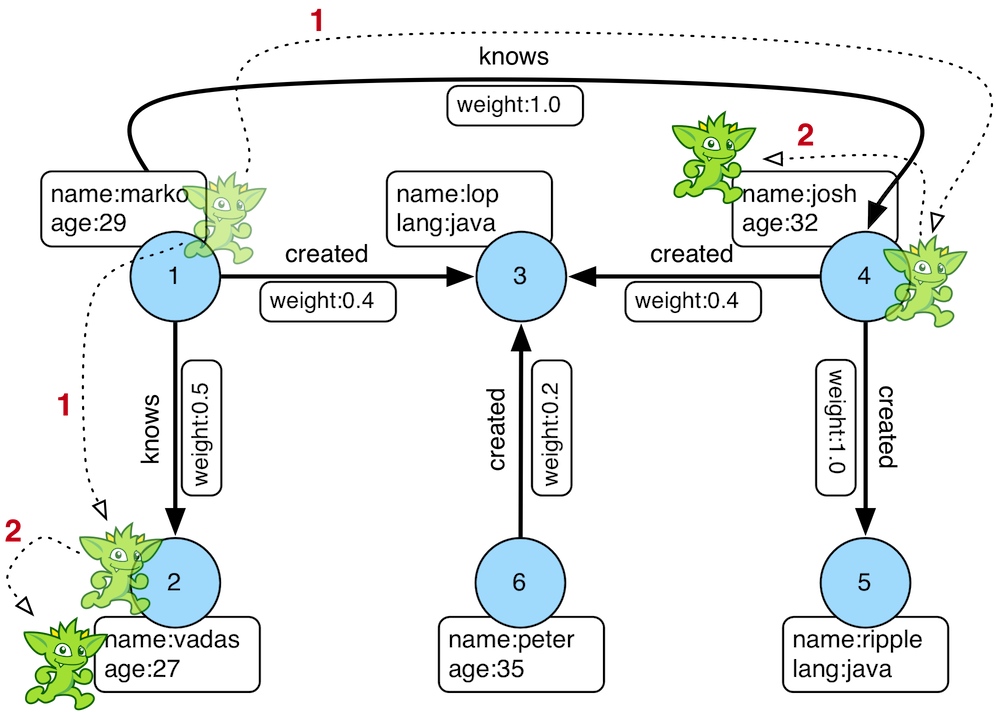Figure 2. The Name of The People That Marko Knows

Or, if the marko-vertex is already realized with a direct reference pointer (i.e. a variable), then the traversal can be spawned off that vertex.

``````gremlin> marko = g.V().has('name','marko').next() //1\
==>v
gremlin> g.V(marko).out('knows') //2\
==>v
==>v
gremlin> g.V(marko).out('knows').values('name') //3\
==>josh``````
``````marko = g.V().has('name','marko').next() //1\
g.V(marko).out('knows') //2\
g.V(marko).out('knows').values('name') //3``````
1. Set the variable `marko` to the vertex in the graph `g` named "marko".

2. Get the vertices that are outgoing adjacent to the marko-vertex via knows-edges.

3. Get the names of the marko-vertex’s friends.

#### The Traverser

When a traversal is executed, the source of the traversal is on the left of the expression (e.g. vertex 1), the steps are the middle of the traversal (e.g. `out('knows')` and `values('name')`), and the results are "traversal.next()'d" out of the right of the traversal (e.g. "vadas" and "josh").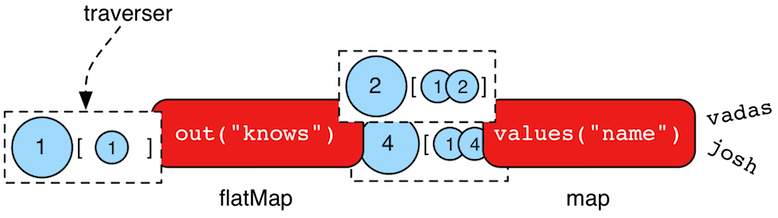The objects propagating through the traversal are wrapped in a `Traverser<T>`. The traverser provides the means by which steps remain stateless. A traverser maintains all the metadata about the traversal — e.g., how many times the traverser has gone through a loop, the path history of the traverser, the current object being traversed, etc. Traverser metadata may be accessed by a step. A classic example is the `path()`-step.

``````gremlin> g.V(marko).out('knows').values('name').path()
==>[v,v,josh]``````
``g.V(marko).out('knows').values('name').path()``
 Warning Path calculation is costly in terms of space as an array of previously seen objects is stored in each path of the respective traverser. Thus, a traversal strategy analyzes the traversal to determine if path metadata is required. If not, then path calculations are turned off.

Another example is the `repeat()`-step which takes into account the number of times the traverser has gone through a particular section of the traversal expression (i.e. a loop).

``````gremlin> g.V(marko).repeat(out()).times(2).values('name')
==>ripple
==>lop``````
``g.V(marko).repeat(out()).times(2).values('name')``
 Warning TinkerPop does not guarantee the order of results returned from a traversal. It only guarantees not to modify the iteration order provided by the underlying graph. Therefore it is important to understand the order guarantees of the graph database being used. A traversal’s result is never ordered by TinkerPop unless performed explicitly by means of `order()`-step.

## Connecting Gremlin

It was established in the initial introductory section that Gremlin is Gremlin is Gremlin, meaning that irrespective of programming language, graph system, etc. the Gremlin written is always of the same general construct making it possible for users to move between development languages and TinkerPop-enabled graph technology easily. This quality of Gremlin generally applies to the traversal language itself. It applies less to the way in which the user connects to a graph to utilize Gremlin, which might differ considerably depending on the programming language or graph database chosen.

How one connects to a graph is a multi-faceted subject that essentially divides along a simple lines determined by the answer to this question: Where is the Gremlin Traversal Machine (GTM)? The reason that this question is so important is because the GTM is responsible for processing traversals. One can write Gremlin traversals in any language, but without a GTM there will be no way to execute that traversal against a TinkerPop-enabled graph. The GTM is typically in one of the following places:

• Embedded in a Java application (i.e. Java Virtual Machine)

• Hosted by a Remote Gremlin Provider (RGP)

The following sections outline each of these models and what impact they have to using Gremlin.

### EmbeddedTinkerPop maintains the reference implementation for the GTM, which is written in Java and thus available for the Java Virtual Machine (JVM). This is the classic model that TinkerPop has long been based on and many examples, blog posts and other resources on the internet will be demonstrated in this style. It is worth noting that the embedded mode is not restricted to just Java as a programming language. Any JVM language can take this approach and in some cases there are language specific wrappers that can help make Gremlin more convenient to use in the style and capability of that language. Examples of these wrappers include gremlin-scala and Ogre (for Clojure).

In this mode, users will start by creating a `Graph` instance, followed by a `GraphTraversalSource` which is the class from which Gremlin traversals are spawned. Graphs that allow this sort of direct instantiation are obviously ones that are JVM-based (or have a JVM-based connector) and directly implement TinkerPop interfaces.

``Graph graph = TinkerGraph.open();``

The "graph" then spawns a `GraphTraversalSource` as follows and typically, by convention, this variable is named "g":

``````GraphTraversalSource g = graph.traversal();
List<Vertex> vertices = g.V().toList()``````
 Note It may be helpful to read the Gremlin Anatomy tutorial, which describes the component parts of Gremlin to get a better understanding of the terminology before proceeding further.

While the TinkerPop Community strives to ensure consistent behavior among all modes of usage, the embedded mode does provide the greatest level of flexibility and control. There are a number of features that can only work if using a JVM language. The following list outlines a number of these available options:

• Lambdas can be written in the native language which is convenient, however, it will reduce the portability of Gremlin to do so should the need arise to switch away from the embedded mode. See more in the Note on Lambdas Section.

• Any features that involve extending TinkerPop Java interfaces - e.g. `VertexProgram`, `TraversalStrategy`, etc. are bound to the JVM. In some cases, these features can be made accessible to non-JVM languages, but they obviously must be initially developed for the JVM.

• Certain built-in `TraversalStrategy` implementations that rely on lambdas or other JVM-only configurations may not be available for use any other way.

• There are no boundaries put in place by serialization (e.g. GraphSON) as embedded graphs are only dealing with Java objects.

• Greater control of graph transactions.

• Direct access to lower-levels of the API - e.g. "structure" API methods like `Vertex` and `Edge` interface methods. As mentioned elsewhere in this documentation, TinkerPop does not recommend direct usage of these methods by end-users.

### Gremlin ServerA JVM-based graph maybe be hosted in TinkerPop’s Gremlin Server. Gremlin Server exposes the graph as an endpoint to which different clients can connect, essentially providing a remote GTM. Gremlin Server supports multiple methods for clients to interface with it:

• Websockets with a custom sub-protocol

• String-based Gremlin scripts

• Bytecode-based Gremlin traversals

• HTTP for string-based scripts

Users are encouraged to use the bytecode-based approach with websockets because it allows them to write Gremlin in the language of their choice. Connecting looks somewhat similar to the embedded approach in that there is a need to create a `GraphTraversalSource`. In the embedded approach, the means for that object’s creation is derived from a `Graph` object which spawns it. In this case, however, the `Graph` instance exists only on the server which means that there is no `Graph` instance to create locally. The approach is to instead create a `GraphTraversalSource` anonymously with `AnonymousTraversalSource` and then apply some "remote" options that describe the location of the Gremlin Server to connect to:

``````import static org.apache.tinkerpop.gremlin.process.traversal.AnonymousTraversalSource.traversal;

GraphTraversalSource g = traversal().withRemote('conf/remote-graph.properties');``````
``````import static org.apache.tinkerpop.gremlin.process.traversal.AnonymousTraversalSource.traversal;

def g = traversal().withRemote('conf/remote-graph.properties')``````
``````using static Gremlin.Net.Process.Traversal.AnonymousTraversalSource;

var g = Traversal().WithRemote(
new DriverRemoteConnection(new GremlinClient(new GremlinServer("localhost", 8182))));``````
``````const traversal = gremlin.process.AnonymousTraversalSource.traversal;

const g = traversal().withRemote(
new DriverRemoteConnection('ws://localhost:8182/gremlin'));``````
``````from gremlin_python.process.anonymous_traversal_source import traversal

g = traversal().withRemote(
DriverRemoteConnection('ws://localhost:8182/gremlin','g'))``````

As shown in the embedded approach in the previous section, once "g" is defined, writing Gremlin is structurally and conceptually the same irrespective of programming language.

#### Limitations

The previous section on the embedded model outlined a number of areas where it has some advantages that it gains due to the fact that the full GTM is available to the user in the language of it’s origin, i.e. Java. Some of those items touch upon important concepts to focus on here.

The first of these points is serialization. When Gremlin Server receives a request, the results must be serialized to the form requested by the client and then the client deserializes those into objects native to the language. TinkerPop has two such formats that it uses with Gryo and GraphSON. Gryo is a JVM-only format and thus carries the advantage that serializing and deserializing occurs on the classes native to the JVM on both the client and server side. As the client has full access to the same classes that the server does it basically has a full GTM on its own and therefore has the ability to do some slightly more advanced things.

A good example is the `subgraph()`-step which returns a `Graph` instance as its result. The subgraph returned from the server can be deserialized into an actual `Graph` instance on the client, which then means it is possible to spawn a `GraphTraversalSource` from that to do local Gremlin traversals on the client-side. For non-JVM Gremlin Language Variants there is no local graph to deserialize that result into and no GTM to process Gremlin so there isn’t much that can be done with such a result.

The second point is related to this issue. As there is no GTM, there is no "structure" API and thus graph elements like `Vertex` and `Edge` are "references" only. A "reference" means that they only contain the `id` and `label` of the element and not the properties. To be consistent, even JVM-based languages hold this limitation when talking to a remote Gremlin Server.

 Important Most SQL developers would not write a query as `SELECT * FROM table`. They would instead write the individual names of the fields they wanted in place of the wildcard. Writing "good" Gremlin is no different with this regard. Prefer explicit property key names in Gremlin unless it is completely impossible to do so.

The third and final point involves transactions. Under this model, one traversal is equivalent to a single transaction and there is no way in TinkerPop to string together multiple traversals into the same transaction.

### Remote Gremlin Provider

Remote Gremlin Providers (RGPs) are showing up more and more often in the graph database space. In TinkerPop terms, this category of graph providers is defined by those who simply support the Gremlin language. Typically, these are server-based graphs, often cloud-based, which accept Gremlin scripts or bytecode as a request and return results. They will often implement Gremlin Server protocols, which enables TinkerPop drivers to connect to them as they would with Gremlin Server. Therefore, the typical connection approach is identical to the method of connection presented in the previous section with the exact same caveats pointed out toward the end.

Despite leveraging TinkerPop protocols and drivers as being typical, RGPs are not required to do so to be considered TinkerPop-enabled. RGPs may well have their own drivers and protocols that may plug into <<gremlin-drivers-variants,Gremlin Language Variants> and may allow for more advanced options like better security, cluster awareness, batched requests or other features. The details of these different systems are outside the scope of this documentation, so be sure to consult their documentation for more information.

## Basic Gremlin

The `GraphTraversalSource` is basically the connection to a graph instance. That graph instance might be embedded, hosted in Gremlin Server or hosted in a RGP, but the `GraphTraversalSource` is agnostic to that. Assuming "g" is the `GraphTraversalSource`, getting data into the graph regardless of programming language or mode of operation is just some basic Gremlin:

``````gremlin> v1 = g.addV('person').property('name','marko').next()
==>v
==>v
``````v1 = g.addV('person').property('name','marko').next()
``````var v1 = g.AddV("person").Property("name", "marko").Next();
``````Vertex v1 = g.addV("person").property("name","marko").next();
``````const v1 = g.addV('person').property('name','marko').next();
``````v1 = g.addV('person').property('name','marko').next()

The first two lines add a vertex each with the vertex label of "person" and the associated "name" property. The third line adds an edge with the "knows" label between them and an associated "weight" property. Note the use of `next()` and `iterate()` at the end of the lines - their effect as terminal steps is described in The Gremlin Console Tutorial.

 Important Writing Gremlin is just one way to load data into the graph. Some graphs may have special data loaders which could be more efficient and make the task easier and faster. It is worth looking into those tools especially if there is a large one-time load to do.

Retrieving this data is also a just writing a Gremlin statement:

``````gremlin> marko = g.V().has('person','name','marko').next()
==>v
gremlin> peopleMarkoKnows = g.V().has('person','name','marko').out('knows').toList()
==>v``````
``````marko = g.V().has('person','name','marko').next()
peopleMarkoKnows = g.V().has('person','name','marko').out('knows').toList()``````
``````var marko = g.V().Has("person", "name", "marko").Next();
var peopleMarkoKnows = g.V().Has("person", "name", "marko").Out("knows").ToList();``````
``````Vertex marko = g.V().has("person","name","marko").next()
List<Vertex> peopleMarkoKnows = g.V().has("person","name","marko").out("knows").toList()``````
``````const marko = g.V().has('person','name','marko').next()
const peopleMarkoKnows = g.V().has('person','name','marko').out('knows').toList()``````
``````marko = g.V().has('person','name','marko').next()
peopleMarkoKnows = g.V().has('person','name','marko').out('knows').toList()``````

In all these examples presented so far there really isn’t a lot of difference in how the Gremlin itself looks. There are a few language syntax specific odds and ends, but for the most part Gremlin looks like Gremlin in all of the different languages.

The library of Gremlin steps with examples for each can be found in The Traversal Section. This section is meant as a reference guide and will not necessarily provide methods for applying Gremlin to solve particular problems. Please see the aforementioned Tutorials Recipes and the Practical Gremlin book for that sort of information.

 Note A full list of helpful Gremlin resources can be found on the TinkerPop Compendium page.

## Staying Agnostic

A good deal has been written in these introductory sections on how TinkerPop enables an agnostic approach to building graph application and that agnosticism is enabled through Gremlin. As good a job as Gremlin can do in this area, it’s evident from the Connecting Gremlin Section that TinkerPop is just an enabler. It does not prevent a developer from making design choices that can limit its protective power.

There are several places to be concerned when considering this issue:

• Data types - Different graphs will support different types of data. Something like TinkerGraph will accept any JVM object, but another graph like Neo4j has a small tight subset of possible types. Choosing a type that is exotic or perhaps is a custom type that only a specific graph supports might create migration friction should the need arise.

• Schemas/Indices - TinkerPop does not provide abstractions for schemas and/or index management. Users will work directly with the API of the graph provider. It is considered good practice to attempt to enclose such code in a graph provider specific class or set of classes to isolate or abstract it.

• Extensions - Graphs may provide extensions to the Gremlin language, which will not be designed to be compatible with other graph providers. There may be a special helper syntax or expressions which can make certain features of that specific graph shine in powerful ways. Using those options is probably recommended, but users should be aware that doing so ties them more tightly to that graph.

• Graph specific semantics - TinkerPop tries to enforce specific semantics through its test suite which is quite extensive, but some graph providers may not completely respect all the semantics of the Gremlin language or TinkerPop’s model for its APIs. For the most part, that doesn’t disqualify them from being any less TinkerPop-enabled than another provider that might meet the semantics perfectly. Take care when considering a new graph and pay attention to what it supports and does not support.

• Graph API - The Graph API (also referred to as the Structure API) is not always accessible to users. Its accessibility is dependent on the choice of graph system and programming language. It is therefore recommended that users avoid usage of methods like `Graph.addVertex()` or `Vertex.properties()` and instead prefer use of Gremlin with `g.addV()` or `g.V(1).properties()`.

Outside of considering these points, the best practice for ensuring the greatest level of compatibility across graphs is to avoid embedded mode and stick to the bytecode based approaches explained in the Gremlin Server and the RGP sections above. It creates the least opportunity to stray from the agnostic path as anything that can be done with those two modes also works in embedded mode. If using embedded mode, simply write code as though the `Graph` instance is "remote" and not local to the JVM. In other words, write code as though the GTM is not available locally. Taking that approach and isolating the points of concern above makes it so that swapping graph providers largely comes down to a configuration task (i.e. modifying configuration files to point at a different graph system).

# The GraphThe Introduction discussed the diversity of TinkerPop-enabled graphs, with special attention paid to the different connection models, and how TinkerPop makes it possible to bridge that diversity in an agnostic manner. This particular section deals with elements of the Graph API which was noted as an API to avoid when trying to build an agnostic system. The Graph API refers to the core elements of what composes the structure of a graph within the Gremlin Traversal Machine (GTM), such as the `Graph`, `Vertex` and `Edge` Java interfaces.

To maintain the most portable code, users should only reference these interfaces. To "reference", simply means to utilize it as a pointer. For `Graph`, that means holding a pointer to the location of graph data and then using it to spawn `GraphTraversalSource` instances so as to write Gremlin:

``````gremlin> graph = TinkerGraph.open()
==>tinkergraph[vertices:0 edges:0]
gremlin> g = graph.traversal()
==>graphtraversalsource[tinkergraph[vertices:0 edges:0], standard]
==>v``````
``````graph = TinkerGraph.open()
g = graph.traversal()

In the above example, "graph" is the `Graph` interface produced by calling `open()` on `TinkerGraph` which creates the instance. Note that while the end intent of the code is to create a "person" vertex, it does not use the APIs on `Graph` to do that - e.g. `graph.addVertex(T.label,'person')`.

Even if the developer desired to use the `graph.addVertex()` method there are only a handful of scenarios where it is possible:

• The application is being developed on the JVM and the developer is using embedded mode

• The architecture includes Gremlin Server and the user is sending Gremlin scripts to the server

• The graph system chosen is a Remote Gremlin Provider and they expose the Graph API via scripts

Note that Gremlin Language Variants force developers to use the Graph API by reference. There is no `addVertex()` method available to GLVs on their respective `Graph` instances, nor are their graph elements filled with data at the call of `properties()`. Developing applications to meet this lowest common denominator in API usage will go a long way to making that application portable across TinkerPop-enabled systems.

When considering the remaining sub-sections that follow, recall that they are all generally bound to the Graph API. They are described here for reference and in some sense backward compatibility with older recommended models of development. In the future, the contents of this section will become less and less relevant.

## Features

A `Feature` implementation describes the capabilities of a `Graph` instance. This interface is implemented by graph system providers for two purposes:

1. It tells users the capabilities of their `Graph` instance.

2. It allows the features they do comply with to be tested against the Gremlin Test Suite - tests that do not comply are "ignored").

The following example in the Gremlin Console shows how to print all the features of a `Graph`:

``````gremlin> graph = TinkerGraph.open()
==>tinkergraph[vertices:0 edges:0]
gremlin> graph.features()
==>FEATURES
> GraphFeatures
>-- Persistence: true
>-- ConcurrentAccess: false
>-- IoWrite: true
>-- Transactions: false
>-- Computer: true
> VariableFeatures
>-- Variables: true
>-- BooleanValues: true
>-- ByteValues: true
>-- DoubleValues: true
>-- FloatValues: true
>-- IntegerValues: true
>-- LongValues: true
>-- MapValues: true
>-- MixedListValues: true
>-- SerializableValues: true
>-- StringValues: true
>-- UniformListValues: true
>-- BooleanArrayValues: true
>-- ByteArrayValues: true
>-- DoubleArrayValues: true
>-- FloatArrayValues: true
>-- IntegerArrayValues: true
>-- LongArrayValues: true
>-- StringArrayValues: true
> VertexFeatures
>-- Upsert: false
>-- RemoveVertices: true
>-- MultiProperties: true
>-- MetaProperties: true
>-- DuplicateMultiProperties: true
>-- RemoveProperty: true
>-- NumericIds: true
>-- StringIds: true
>-- UuidIds: true
>-- CustomIds: false
>-- AnyIds: true
>-- UserSuppliedIds: true
> VertexPropertyFeatures
>-- RemoveProperty: true
>-- NumericIds: true
>-- StringIds: true
>-- UuidIds: true
>-- CustomIds: false
>-- AnyIds: true
>-- UserSuppliedIds: true
>-- Properties: true
>-- BooleanValues: true
>-- ByteValues: true
>-- DoubleValues: true
>-- FloatValues: true
>-- IntegerValues: true
>-- LongValues: true
>-- MapValues: true
>-- MixedListValues: true
>-- SerializableValues: true
>-- StringValues: true
>-- UniformListValues: true
>-- BooleanArrayValues: true
>-- ByteArrayValues: true
>-- DoubleArrayValues: true
>-- FloatArrayValues: true
>-- IntegerArrayValues: true
>-- LongArrayValues: true
>-- StringArrayValues: true
> EdgeFeatures
>-- RemoveEdges: true
>-- Upsert: false
>-- RemoveProperty: true
>-- NumericIds: true
>-- StringIds: true
>-- UuidIds: true
>-- CustomIds: false
>-- AnyIds: true
>-- UserSuppliedIds: true
> EdgePropertyFeatures
>-- Properties: true
>-- BooleanValues: true
>-- ByteValues: true
>-- DoubleValues: true
>-- FloatValues: true
>-- IntegerValues: true
>-- LongValues: true
>-- MapValues: true
>-- MixedListValues: true
>-- SerializableValues: true
>-- StringValues: true
>-- UniformListValues: true
>-- BooleanArrayValues: true
>-- ByteArrayValues: true
>-- DoubleArrayValues: true
>-- FloatArrayValues: true
>-- IntegerArrayValues: true
>-- LongArrayValues: true
>-- StringArrayValues: true``````
``````graph = TinkerGraph.open()
graph.features()``````

A common pattern for using features is to check their support prior to performing an operation:

``````gremlin> graph.features().graph().supportsTransactions()
==>false
gremlin> graph.features().graph().supportsTransactions() ? g.tx().commit() : "no tx"
==>no tx``````
``````graph.features().graph().supportsTransactions()
graph.features().graph().supportsTransactions() ? g.tx().commit() : "no tx"``````
 Tip To ensure provider agnostic code, always check feature support prior to usage of a particular function. In that way, the application can behave gracefully in case a particular implementation is provided at runtime that does not support a function being accessed.
 Warning Features of reference graphs which are used to connect to remote graphs do not reflect the features of the graph to which it connects. It reflects the features of instantiated graph itself, which will likely be quite different considering that reference graphs will typically be immutable.

## Vertex Properties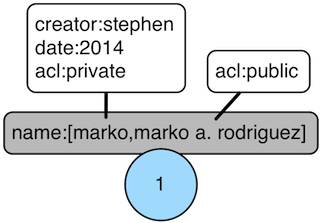TinkerPop introduces the concept of a `VertexProperty<V>`. All the properties of a `Vertex` are a `VertexProperty`. A `VertexProperty` implements `Property` and as such, it has a key/value pair. However, `VertexProperty` also implements `Element` and thus, can have a collection of key/value pairs. Moreover, while an `Edge` can only have one property of key "name" (for example), a `Vertex` can have multiple "name" properties. With the inclusion of vertex properties, two features are introduced which ultimately advance the graph modelers toolkit:

1. Multiple properties (multi-properties): a vertex property key can have multiple values. For example, a vertex can have multiple "name" properties.

2. Properties on properties (meta-properties): a vertex property can have properties (i.e. a vertex property can have key/value data associated with it).

Possible use cases for meta-properties:

1. Permissions: Vertex properties can have key/value ACL-type permission information associated with them.

2. Auditing: When a vertex property is manipulated, it can have key/value information attached to it saying who the creator, deletor, etc. are.

3. Provenance: The "name" of a vertex can be declared by multiple users. For example, there may be multiple spellings of a name from different sources.

A running example using vertex properties is provided below to demonstrate and explain the API.

``````gremlin> graph = TinkerGraph.open()
==>tinkergraph[vertices:0 edges:0]
gremlin> g = graph.traversal()
==>graphtraversalsource[tinkergraph[vertices:0 edges:0], standard]
gremlin> v = g.addV().property('name','marko').property('name','marko a. rodriguez').next()
==>v
gremlin> g.V(v).properties('name').count() //1\
==>2
gremlin> v.property(list, 'name', 'm. a. rodriguez') //2\
==>vp[name->m. a. rodriguez]
gremlin> g.V(v).properties('name').count()
==>3
gremlin> g.V(v).properties()
==>vp[name->marko]
==>vp[name->marko a. rodriguez]
==>vp[name->m. a. rodriguez]
gremlin> g.V(v).properties('name')
==>vp[name->marko]
==>vp[name->marko a. rodriguez]
==>vp[name->m. a. rodriguez]
gremlin> g.V(v).properties('name').hasValue('marko')
==>vp[name->marko]
gremlin> g.V(v).properties('name').hasValue('marko').property('acl','private') //3\
==>vp[name->marko]
gremlin> g.V(v).properties('name').hasValue('marko a. rodriguez')
==>vp[name->marko a. rodriguez]
gremlin> g.V(v).properties('name').hasValue('marko a. rodriguez').property('acl','public')
==>vp[name->marko a. rodriguez]
gremlin> g.V(v).properties('name').has('acl','public').value()
==>marko a. rodriguez
gremlin> g.V(v).properties('name').has('acl','public').drop() //4\
gremlin> g.V(v).properties('name').has('acl','public').value()
gremlin> g.V(v).properties('name').has('acl','private').value()
==>marko
gremlin> g.V(v).properties()
==>vp[name->marko]
==>vp[name->m. a. rodriguez]
gremlin> g.V(v).properties().properties() //5\
==>p[acl->private]
gremlin> g.V(v).properties().property('date',2014) //6\
==>vp[name->marko]
==>vp[name->m. a. rodriguez]
gremlin> g.V(v).properties().property('creator','stephen')
==>vp[name->marko]
==>vp[name->m. a. rodriguez]
gremlin> g.V(v).properties().properties()
==>p[date->2014]
==>p[creator->stephen]
==>p[acl->private]
==>p[date->2014]
==>p[creator->stephen]
gremlin> g.V(v).properties('name').valueMap()
==>[date:2014,creator:stephen,acl:private]
==>[date:2014,creator:stephen]
gremlin> g.V(v).property('name','okram') //7\
==>v
gremlin> g.V(v).properties('name')
==>vp[name->okram]
gremlin> g.V(v).values('name') //8\
==>okram``````
``````graph = TinkerGraph.open()
g = graph.traversal()
g.V(v).properties('name').count() //1\
v.property(list, 'name', 'm. a. rodriguez') //2\
g.V(v).properties('name').count()
g.V(v).properties()
g.V(v).properties('name')
g.V(v).properties('name').hasValue('marko')
g.V(v).properties('name').hasValue('marko').property('acl','private') //3\
g.V(v).properties('name').hasValue('marko a. rodriguez')
g.V(v).properties('name').hasValue('marko a. rodriguez').property('acl','public')
g.V(v).properties('name').has('acl','public').value()
g.V(v).properties('name').has('acl','public').drop() //4\
g.V(v).properties('name').has('acl','public').value()
g.V(v).properties('name').has('acl','private').value()
g.V(v).properties()
g.V(v).properties().properties() //5\
g.V(v).properties().property('date',2014) //6\
g.V(v).properties().property('creator','stephen')
g.V(v).properties().properties()
g.V(v).properties('name').valueMap()
g.V(v).property('name','okram') //7\
g.V(v).properties('name')
g.V(v).values('name') //8``````
1. A vertex can have zero or more properties with the same key associated with it.

2. If a property is added with a cardinality of `Cardinality.list`, an additional property with the provided key will be added.

3. A vertex property can have standard key/value properties attached to it.

4. Vertex property removal is identical to property removal.

5. Gets the meta-properties of each vertex property.

6. A vertex property can have any number of key/value properties attached to it.

7. `property(…​)` will remove all existing key’d properties before adding the new single property (see `VertexProperty.Cardinality`).

8. If only the value of a property is needed, then `values()` can be used.

If the concept of vertex properties is difficult to grasp, then it may be best to think of vertex properties in terms of "literal vertices." A vertex can have an edge to a "literal vertex" that has a single value key/value — e.g. "value=okram." The edge that points to that literal vertex has an edge-label of "name." The properties on the edge represent the literal vertex’s properties. The "literal vertex" can not have any other edges to it (only one from the associated vertex).

 Tip A toy graph demonstrating all of the new TinkerPop graph structure features is available at `TinkerFactory.createTheCrew()` and `data/tinkerpop-crew*`. This graph demonstrates multi-properties and meta-properties.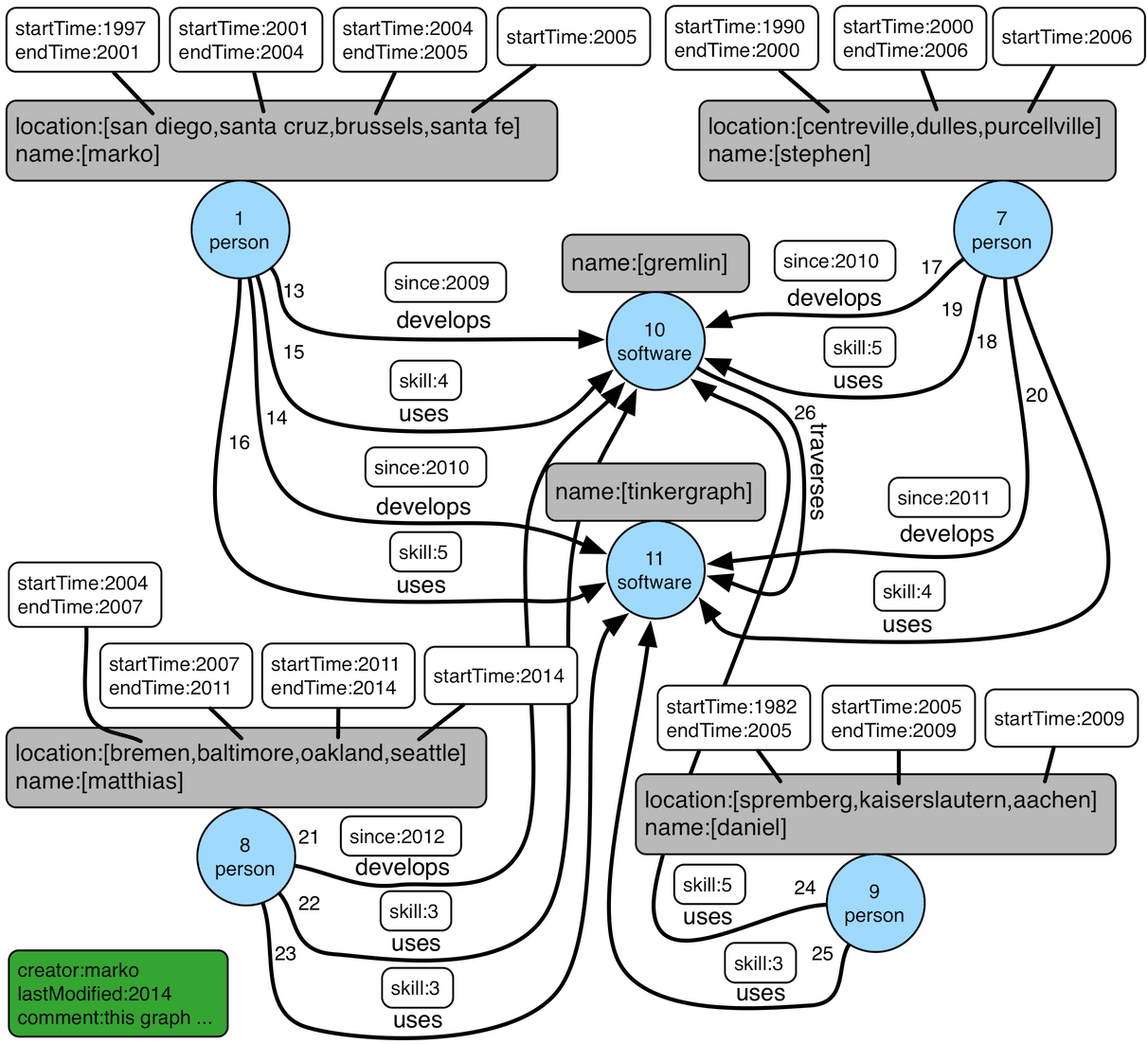Figure 3. TinkerPop Crew
``````gremlin> g.V().as('a').
properties('location').as('b').
hasNot('endTime').as('c').
select('a','b','c').by('name').by(value).by('startTime') // determine the current location of each person
==>[a:marko,b:santa fe,c:2005]
==>[a:stephen,b:purcellville,c:2006]
==>[a:matthias,b:seattle,c:2014]
==>[a:daniel,b:aachen,c:2009]
gremlin> g.V().has('name','gremlin').inE('uses').
order().by('skill',asc).as('a').
outV().as('b').
select('a','b').by('skill').by('name') // rank the users of gremlin by their skill level
==>[a:3,b:matthias]
==>[a:4,b:marko]
==>[a:5,b:stephen]
==>[a:5,b:daniel]``````
``````g.V().as('a').
properties('location').as('b').
hasNot('endTime').as('c').
select('a','b','c').by('name').by(value).by('startTime') // determine the current location of each person
g.V().has('name','gremlin').inE('uses').
order().by('skill',asc).as('a').
outV().as('b').
select('a','b').by('skill').by('name') // rank the users of gremlin by their skill level``````

## Graph Variables

`Graph.Variables` are key/value pairs associated with the graph itself — in essence, a `Map<String,Object>`. These variables are intended to store metadata about the graph. Example use cases include:

• Schema information: What do the namespace prefixes resolve to and when was the schema last modified?

• Global permissions: What are the access rights for particular groups?

• System user information: Who are the admins of the system?

An example of graph variables in use is presented below:

``````gremlin> graph = TinkerGraph.open()
==>tinkergraph[vertices:0 edges:0]
gremlin> graph.variables()
==>variables[size:0]
gremlin> graph.variables().set('systemUsers',['matthias','marko','josh'])
gremlin> graph.variables().keys()
==>systemUsers
gremlin> graph.variables().get('systemUsers')
==>Optional[[matthias, marko, josh]]
gremlin> graph.variables().get('systemUsers').get()
==>matthias
==>marko
==>josh
gremlin> graph.variables().keys()
==>systemUsers``````
``````graph = TinkerGraph.open()
graph.variables()
graph.variables().set('systemUsers',['matthias','marko','josh'])
graph.variables().keys()
graph.variables().get('systemUsers')
graph.variables().get('systemUsers').get()
graph.variables().keys()``````
 Important Graph variables are not intended to be subject to heavy, concurrent mutation nor to be used in complex computations. The intention is to have a location to store data about the graph for administrative purposes.
 Warning Attempting to set graph variables in a reference graph will not promote them to the remote graph. Typically, a reference graph has immutable features and will not support this features.

## Graph TransactionsA database transaction represents a unit of work to execute against the database. Transactions in TinkerPop can be considered in several contexts: transactions for embedded graphs via the Graph API, transactions for Gremlin Server and transactions within Remote Gremlin Providers. For those following recommended patterns, the concepts presented in the embedded section should generally be of little interest and are present mainly for reference. Utilizing those transactional features will greatly reduce the portability of an application’s Gremlin code.

### Embedded

When on the JVM using an embedded graph, there is considerable flexibility for working with transactions. With the Graph API, transactions are controlled by an implementation of the `Transaction` interface and that object can be obtained from the `Graph` interface using the `tx()` method. It is important to note that the `Transaction` object does not represent a "transaction" itself. It merely exposes the methods for working with transactions (e.g. committing, rolling back, etc).

Most `Graph` implementations that `supportsTransactions` will implement an "automatic" `ThreadLocal` transaction, which means that when a read or write occurs after the `Graph` is instantiated, a transaction is automatically started within that thread. There is no need to manually call a method to "create" or "start" a transaction. Simply modify the graph as required and call `graph.tx().commit()` to apply changes or `graph.tx().rollback()` to undo them. When the next read or write action occurs against the graph, a new transaction will be started within that current thread of execution.

When using transactions in this fashion, especially in web application (e.g. HTTP server), it is important to ensure that transactions do not leak from one request to the next. In other words, unless a client is somehow bound via session to process every request on the same server thread, every request must be committed or rolled back at the end of the request. By ensuring that the request encapsulates a transaction, it ensures that a future request processed on a server thread is starting in a fresh transactional state and will not have access to the remains of one from an earlier request. A good strategy is to rollback a transaction at the start of a request, so that if it so happens that a transactional leak does occur between requests somehow, a fresh transaction is assured by the fresh request.

 Tip The `tx()` method is on the `Graph` interface, but it is also available on the `TraversalSource` spawned from a `Graph`. Calls to `TraversalSource.tx()` are proxied through to the underlying `Graph` as a convenience.
 Warning TinkerPop provides for basic transaction control, however, like many aspects of TinkerPop, it is up to the graph system provider to choose the specific aspects of how their implementation will work and how it fits into the TinkerPop stack. Be sure to understand the transaction semantics of the specific graph implementation that is being utilized as it may present differing functionality than described here.

#### Configuring

Determining when a transaction starts is dependent upon the behavior assigned to the `Transaction`. It is up to the `Graph` implementation to determine the default behavior and unless the implementation doesn’t allow it, the behavior itself can be altered via these `Transaction` methods:

``````public Transaction onReadWrite(Consumer<Transaction> consumer);

public Transaction onClose(Consumer<Transaction> consumer);``````

Providing a `Consumer` function to `onReadWrite` allows definition of how a transaction starts when a read or a write occurs. `Transaction.READ_WRITE_BEHAVIOR` contains pre-defined `Consumer` functions to supply to the `onReadWrite` method. It has two options:

• `AUTO` - automatic transactions where the transaction is started implicitly to the read or write operation

• `MANUAL` - manual transactions where it is up to the user to explicitly open a transaction, throwing an exception if the transaction is not open

Providing a `Consumer` function to `onClose` allows configuration of how a transaction is handled when `Transaction.close()` is called. `Transaction.CLOSE_BEHAVIOR` has several pre-defined options that can be supplied to this method:

• `COMMIT` - automatically commit an open transaction

• `ROLLBACK` - automatically rollback an open transaction

• `MANUAL` - throw an exception if a transaction is open, forcing the user to explicitly close the transaction

 Important As transactions are `ThreadLocal` in nature, so are the transaction configurations for `onReadWrite` and `onClose`.

Once there is an understanding for how transactions are configured, most of the rest of the `Transaction` interface is self-explanatory. Note that Neo4j-Gremlin is used for the examples to follow as TinkerGraph does not support transactions.

``````gremlin> graph = Neo4jGraph.open('/tmp/neo4j')
==>neo4jgraph[EmbeddedGraphDatabase [/tmp/neo4j]]
gremlin> g = graph.traversal()
==>graphtraversalsource[neo4jgraph[community single [/tmp/neo4j]], standard]
gremlin> graph.features()
==>FEATURES
> GraphFeatures
>-- Transactions: true  //1
>-- Computer: false
>-- Persistence: true
...
==>org.apache.tinkerpop.gremlin.neo4j.structure.Neo4jGraph\$Neo4jTransaction@1c067c0d
==>v
gremlin> g.tx().commit() //4
==>null
==>org.apache.tinkerpop.gremlin.neo4j.structure.Neo4jGraph\$Neo4jTransaction@1c067c0d
gremlin> g.tx().isOpen()
==>false
Open a transaction before attempting to read/write the transaction
gremlin> g.tx().open() //7
==>null
==>v
gremlin> g.tx().commit()
==>null``````
1. Check `features` to ensure that the graph supports transactions.

2. By default, `Neo4jGraph` is configured with "automatic" transactions, so it is set here for demonstration purposes only.

3. When the vertex is added, the transaction is automatically started. From this point, more mutations can be staged or other read operations executed in the context of that open transaction.

4. Calling `commit` finalizes the transaction.

5. Change transaction behavior to require manual control.

6. Adding a vertex now results in failure because the transaction was not explicitly opened.

7. Explicitly open a transaction.

8. Adding a vertex now succeeds as the transaction was manually opened.

 Note It may be important to consult the documentation of the `Graph` implementation you are using when it comes to the specifics of how transactions will behave. TinkerPop allows some latitude in this area and implementations may not have the exact same behaviors and ACID guarantees.

Most `Graph` implementations that support transactions do so in a `ThreadLocal` manner, where the current transaction is bound to the current thread of execution. Consider the following example to demonstrate:

``````GraphTraversalSource g = graph.traversal();

});

});

t1.start()
t2.start()

t1.join()
t2.join()

g.tx().commit();``````

The above code shows three vertices added to `graph` in three different threads: the current thread, `t1` and `t2`. One might expect that by the time this body of code finished executing, that there would be three vertices persisted to the `Graph`. However, given the `ThreadLocal` nature of transactions, there really were three separate transactions created in that body of code (i.e. one for each thread of execution) and the only one committed was the first call to `addV()` in the primary thread of execution. The other two calls to that method within `t1` and `t2` were never committed and thus orphaned.

A `Graph` that `supportsThreadedTransactions` is one that allows for a `Graph` to operate outside of that constraint, thus allowing multiple threads to operate within the same transaction. Therefore, if there was a need to have three different threads operating within the same transaction, the above code could be re-written as follows:

``````Graph threaded = graph.tx().createThreadedTx();
GraphTraversalSource g = graph.traversal();

});

});

t1.start()
t2.start()

t1.join()
t2.join()

g.tx().commit();``````

In the above case, the call to `graph.tx().createThreadedTx()` creates a new `Graph` instance that is unbound from the `ThreadLocal` transaction, thus allowing each thread to operate on it in the same context. In this case, there would be three separate vertices persisted to the `Graph`.

### Gremlin Server

The available capability for transactions with Gremlin Server is dependent upon the method of interaction that is used. The preferred method for interacting with Gremlin Server is via websockets and bytecode based requests. In this mode of operations each Gremlin traversal that is executed will be treated as a single transaction. Traversals that fail will have their transaction rolled back and successful iteration of a traversal will conclude with a transactional commit. How the graph hosted in Gremlin Server reacts to those commands is dependent on the graph chosen and it is therefore important to understand the transactional semantics of that graph when developing an application.

Gremlin Server also has the option to accept Gremlin-based scripts. The scripting approach provides access to the Graph API and thus also the transactional model described in the embedded section. Therefore a single script can have the ability to execute multiple transactions per request with complete control provided to the developer to commit or rollback transactions as needed.

There are two methods for sending scripts to Gremlin Server: sessionless and session-based. With sessionless requests there will always be an attempt to close the transaction at the end of the request with a commit if there are no errors or a rollback if there is a failure. It is therefore unnecessary to close transactions manually within scripts themselves. By default, session-based requests do not have this quality. The transaction will be held open on the server until the user closes it manually. There is an option to have automatic transaction management for sessions. More information on this topic can be found in the Considering Transactions Section and the Considering Sessions Section.

While those sections provide some additional details, the short advice is to avoid scripts when possible and prefer bytecode based requests.

### Remote Gremlin Providers

At this time, transactional patterns for Remote Gremlin Providers are largely in line with Gremlin Server. Most offer bytecode or script based sessionless requests, which have automatic transaction management, such that a successful traversal will commit on success and a failing traversal will rollback. As most of these RGPs do not expose a `Graph` instances, access to lower level transactional functions even in a sessionless fashion are not typically allowed. The nature of what a "transaction" means will be dependent on the RGP as is the case with any TinkerPop-enabled graph system, so it is important to consult that systems documentation for more details.

## Namespace Conventions

End users, graph system providers, `GraphComputer` algorithm designers, GremlinPlugin creators, etc. all leverage properties on elements to store information. There are a few conventions that should be respected when naming property keys to ensure that conflicts between these stakeholders do not conflict.

• End users are granted the flat namespace (e.g. `name`, `age`, `location`) to key their properties and label their elements.

• Graph system providers are granted the hidden namespace (e.g. `~metadata`) to key their properties and labels. Data keyed as such is only accessible via the graph system implementation and no other stakeholders are granted read nor write access to data prefixed with "~" (see `Graph.Hidden`). Test coverage and exceptions exist to ensure that graph systems respect this hard boundary.

• `VertexProgram` and `MapReduce` developers should leverage qualified namespaces particular to their domain (e.g. `mydomain.myvertexprogram.computedata`).

• `GremlinPlugin` creators should prefix their plugin name with their domain (e.g. `mydomain.myplugin`).

 Important TinkerPop uses `tinkerpop.` and `gremlin.` as the prefixes for provided strategies, vertex programs, map reduce implementations, and plugins.

The only truly protected namespace is the hidden namespace provided to graph systems. From there, it’s up to engineers to respect the namespacing conventions presented.

# The TraversalAt the most general level there is `Traversal<S,E>` which implements `Iterator<E>`, where the `S` stands for start and the `E` stands for end. A traversal is composed of four primary components:

1. `Step<S,E>`: an individual function applied to `S` to yield `E`. Steps are chained within a traversal.

2. `TraversalStrategy`: interceptor methods to alter the execution of the traversal (e.g. query re-writing).

3. `TraversalSideEffects`: key/value pairs that can be used to store global information about the traversal.

4. `Traverser<T>`: the object propagating through the `Traversal` currently representing an object of type `T`.

The classic notion of a graph traversal is provided by `GraphTraversal<S,E>` which extends `Traversal<S,E>`. `GraphTraversal` provides an interpretation of the graph data in terms of vertices, edges, etc. and thus, a graph traversal DSL.

 Important The underlying `Step` implementations provided by TinkerPop should encompass most of the functionality required by a DSL author. It is important that DSL authors leverage the provided steps as then the common optimization and decoration strategies can reason on the underlying traversal sequence. If new steps are introduced, then common traversal strategies may not function properly.

## Graph Traversal Steps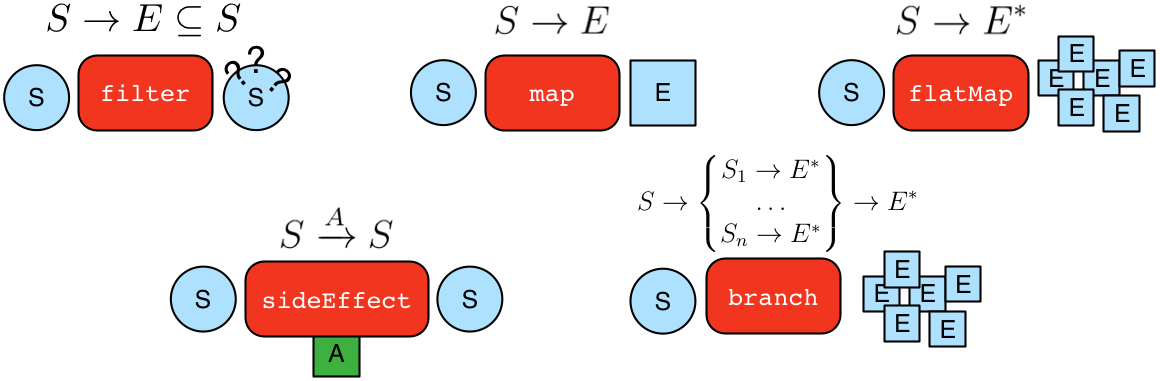A `GraphTraversal<S,E>` is spawned from a `GraphTraversalSource`. It can also be spawned anonymously (i.e. empty) via `__`. A graph traversal is composed of an ordered list of steps. All the steps provided by `GraphTraversal` inherit from the more general forms diagrammed above. A list of all the steps (and their descriptions) are provided in the TinkerPop GraphTraversal JavaDoc. The following subsections will demonstrate the GraphTraversal steps using the Gremlin Console.

 Important The basics for starting a traversal are described in The Graph Process section as well as in the Getting Started tutorial.
 Note To reduce the verbosity of the expression, it is good to `import static org.apache.tinkerpop.gremlin.process.traversal.dsl.graph.__.*`. This way, instead of doing `__.inE()` for an anonymous traversal, it is possible to simply write `inE()`. Be aware of language-specific reserved keywords when using anonymous traversals. For example, `in` and `as` are reserved keywords in Groovy, therefore you must use the verbose syntax `__.in()` and `__.as()` to avoid collisions.

### General Steps

There are five general steps, each having a traversal and a lambda representation, by which all other specific steps described later extend.

Step Description

`map(Traversal<S, E>)` `map(Function<Traverser<S>, E>)`

map the traverser to some object of type `E` for the next step to process.

`flatMap(Traversal<S, E>)` `flatMap(Function<Traverser<S>, Iterator<E>>)`

map the traverser to an iterator of `E` objects that are streamed to the next step.

`filter(Traversal<?, ?>)` `filter(Predicate<Traverser<S>>)`

map the traverser to either true or false, where false will not pass the traverser to the next step.

`sideEffect(Traversal<S, S>)` `sideEffect(Consumer<Traverser<S>>)`

perform some operation on the traverser and pass it to the next step.

`branch(Traversal<S, M>)` `branch(Function<Traverser<S>,M>)`

split the traverser to all the traversals indexed by the `M` token.

 Warning Lambda steps are presented for educational purposes as they represent the foundational constructs of the Gremlin language. In practice, lambda steps should be avoided in favor of their traversals representation and traversal verification strategies exist to disallow their use unless explicitly "turned off." For more information on the problems with lambdas, please read A Note on Lambdas.

The `Traverser<S>` object provides access to:

1. The current traversed `S` object — `Traverser.get()`.

2. The current path traversed by the traverser — `Traverser.path()`.

1. A helper shorthand to get a particular path-history object — `Traverser.path(String) == Traverser.path().get(String)`.

3. The number of times the traverser has gone through the current loop — `Traverser.loops()`.

4. The number of objects represented by this traverser — `Traverser.bulk()`.

5. The local data structure associated with this traverser — `Traverser.sack()`.

6. The side-effects associated with the traversal — `Traverser.sideEffects()`.

1. A helper shorthand to get a particular side-effect — `Traverser.sideEffect(String) == Traverser.sideEffects().get(String)`.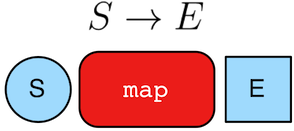``````gremlin> g.V(1).out().values('name') //1\
==>lop
==>josh
gremlin> g.V(1).out().map {it.get().value('name')} //2\
==>lop
==>josh
gremlin> g.V(1).out().map(values('name')) //3\
==>lop
==>josh``````
``````g.V(1).out().values('name') //1\
g.V(1).out().map {it.get().value('name')} //2\
g.V(1).out().map(values('name')) //3``````
1. An outgoing traversal from vertex 1 to the name values of the adjacent vertices.

2. The same operation, but using a lambda to access the name property values.

3. Again the same operation, but using the traversal representation of `map()`.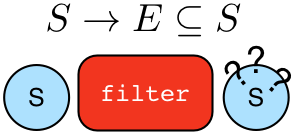``````gremlin> g.V().filter {it.get().label() == 'person'} //1\
==>v
==>v
==>v
==>v
gremlin> g.V().filter(label().is('person')) //2\
==>v
==>v
==>v
==>v
gremlin> g.V().hasLabel('person') //3\
==>v
==>v
==>v
==>v``````
``````g.V().filter {it.get().label() == 'person'} //1\
g.V().filter(label().is('person')) //2\
g.V().hasLabel('person') //3``````
1. A filter that only allows the vertex to pass if it has the "person" label

2. The same operation, but using the traversal representation of `filter()`.

3. The more specific `has()`-step is implemented as a `filter()` with respective predicate.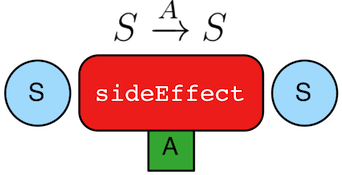``````gremlin> g.V().hasLabel('person').sideEffect(System.out.&println) //1\
v
==>v
v
==>v
v
==>v
v
==>v
gremlin> g.V().sideEffect(outE().count().store("o")).
sideEffect(inE().count().store("i")).cap("o","i") //2\
==>[i:[0,0,1,1,1,3],o:[3,0,0,0,2,1]]``````
``````g.V().hasLabel('person').sideEffect(System.out.&println) //1\
g.V().sideEffect(outE().count().store("o")).
sideEffect(inE().count().store("i")).cap("o","i") //2``````
1. Whatever enters `sideEffect()` is passed to the next step, but some intervening process can occur.

2. Compute the out- and in-degree for each vertex. Both `sideEffect()` are fed with the same vertex.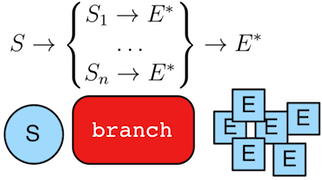``````gremlin> g.V().branch {it.get().value('name')}.
option('marko', values('age')).
option(none, values('name')) //1\
==>29
==>lop
==>josh
==>ripple
==>peter
gremlin> g.V().branch(values('name')).
option('marko', values('age')).
option(none, values('name')) //2\
==>29
==>lop
==>josh
==>ripple
==>peter
gremlin> g.V().choose(has('name','marko'),
values('age'),
values('name')) //3\
==>29
==>lop
==>josh
==>ripple
==>peter``````
``````g.V().branch {it.get().value('name')}.
option('marko', values('age')).
option(none, values('name')) //1\
g.V().branch(values('name')).
option('marko', values('age')).
option(none, values('name')) //2\
g.V().choose(has('name','marko'),
values('age'),
values('name')) //3``````
1. If the vertex is "marko", get his age, else get the name of the vertex.

2. The same operation, but using the traversal representing of `branch()`.

3. The more specific boolean-based `choose()`-step is implemented as a `branch()`.

### Terminal Steps

Typically, when a step is concatenated to a traversal a traversal is returned. In this way, a traversal is built up in a fluent, monadic fashion. However, some steps do not return a traversal, but instead, execute the traversal and return a result. These steps are known as terminal steps (terminal) and they are explained via the examples below.

``````gremlin> g.V().out('created').hasNext() //1\
==>true
gremlin> g.V().out('created').next() //2\
==>v
gremlin> g.V().out('created').next(2) //3\
==>v
==>v
gremlin> g.V().out('nothing').tryNext() //4\
==>Optional.empty
gremlin> g.V().out('created').toList() //5\
==>v
==>v
==>v
==>v
gremlin> g.V().out('created').toSet() //6\
==>v
==>v
gremlin> g.V().out('created').toBulkSet() //7\
==>v
==>v
==>v
==>v
gremlin> results = ['blah',3]
==>blah
==>3
gremlin> g.V().out('created').fill(results) //8\
==>blah
==>3
==>v
==>v
==>v
==>v
``````g.V().out('created').hasNext() //1\
g.V().out('created').next() //2\
g.V().out('created').next(2) //3\
g.V().out('nothing').tryNext() //4\
g.V().out('created').toList() //5\
g.V().out('created').toSet() //6\
g.V().out('created').toBulkSet() //7\
results = ['blah',3]
g.V().out('created').fill(results) //8\
1. `hasNext()` determines whether there are available results (not supported in `gremlin-javascript`).

2. `next()` will return the next result.

3. `next(n)` will return the next `n` results in a list (not supported in `gremlin-javascript` or Gremlin.NET).

4. `tryNext()` will return an `Optional` and thus, is a composite of `hasNext()`/`next()` (only supported for JVM languages).

5. `toList()` will return all results in a list.

6. `toSet()` will return all results in a set and thus, duplicates removed (not supported in `gremlin-javascript`).

7. `toBulkSet()` will return all results in a weighted set and thus, duplicates preserved via weighting (only supported for JVM languages).

8. `fill(collection)` will put all results in the provided collection and return the collection when complete (only supported for JVM languages).

9. `iterate()` does not exactly fit the definition of a terminal step in that it doesn’t return a result, but still returns a traversal - it does however behave as a terminal step in that it iterates the traversal and generates side effects without returning the actual result.

There is also the `promise()` terminator step, which can only be used with remote traversals to Gremlin Server or RGPs. It starts a promise to execute a function on the current `Traversal` that will be completed in the future.

Finally, `explain()`-step is also a terminal step and is described in its own section.

Reasoning is the process of making explicit what is implicit in the data. What is explicit in a graph are the objects of the graph — i.e. vertices and edges. What is implicit in the graph is the traversal. In other words, traversals expose meaning where the meaning is determined by the traversal definition. For example, take the concept of a "co-developer." Two people are co-developers if they have worked on the same project together. This concept can be represented as a traversal and thus, the concept of "co-developers" can be derived. Moreover, what was once implicit can be made explicit via the `addE()`-step (map/sideEffect).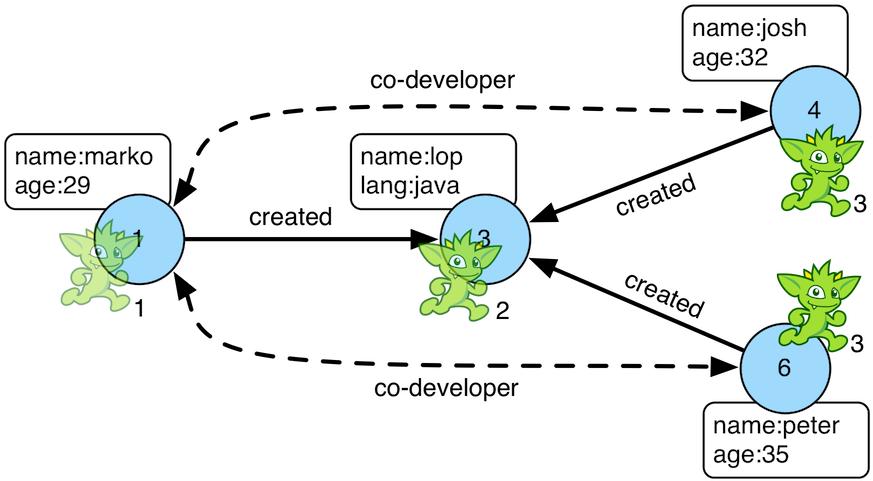``````gremlin> g.V(1).as('a').out('created').in('created').where(neq('a')).
==>e[1-co-developer->4]
==>e[1-co-developer->6]
gremlin> g.V(3,4,5).aggregate('x').has('name','josh').as('a').
==>e[3-createdBy->4]
==>e[5-createdBy->4]
==>e[3-createdBy->1]
==>e[5-createdBy->4]
==>e[3-createdBy->4]
==>e[3-createdBy->6]
gremlin> g.V(1).as('a').out('knows').
inV().inE('livesNear').values('year') //4\
==>2009
==>2009
gremlin> g.V().match(
__.as('a').out('knows').as('b'),
__.as('a').out('created').as('c'),
__.as('b').out('created').as('c')).
property(id,23).property('project',select('c').values('name')) //5\
==>e[1-friendlyCollaborator->4]
gremlin> g.E(23).valueMap()
==>[project:lop]
gremlin> marko = g.V().has('name','marko').next()
==>v
gremlin> peter = g.V().has('name','peter').next()
==>v
==>e[1-knows->6]
==>e[1-knows->6]``````
``````g.V(1).as('a').out('created').in('created').where(neq('a')).
g.V(3,4,5).aggregate('x').has('name','josh').as('a').
g.V(1).as('a').out('knows').
inV().inE('livesNear').values('year') //4\
g.V().match(
__.as('a').out('knows').as('b'),
__.as('a').out('created').as('c'),
__.as('b').out('created').as('c')).
property(id,23).property('project',select('c').values('name')) //5\
g.E(23).valueMap()
marko = g.V().has('name','marko').next()
peter = g.V().has('name','peter').next()
1. Add a co-developer edge with a year-property between marko and his collaborators.

2. Add incoming createdBy edges from the josh-vertex to the lop- and ripple-vertices.

3. Add an inverse createdBy edge for all created edges.

4. The newly created edge is a traversable object.

5. Two arbitrary bindings in a traversal can be joined `from()`→`to()`, where `id` can be provided for graphs that supports user provided ids.

6. Add an edge between marko and peter given the directed (detached) vertex references.

7. Add an edge between marko and peter given the directed (detached) vertex references.

The `addV()`-step is used to add vertices to the graph (map/sideEffect). For every incoming object, a vertex is created. Moreover, `GraphTraversalSource` maintains an `addV()` method.

``````gremlin> g.addV('person').property('name','stephen')
==>v
gremlin> g.V().values('name')
==>marko
==>lop
==>josh
==>ripple
==>peter
==>stephen
==>v
==>v
gremlin> g.V().has('name','nothing')
==>v
==>v
gremlin> g.V().has('name','nothing').bothE()``````
``````g.addV('person').property('name','stephen')
g.V().values('name')
g.V().has('name','nothing')
g.V().has('name','nothing').bothE()``````

The `property()`-step is used to add properties to the elements of the graph (sideEffect). Unlike `addV()` and `addE()`, `property()` is a full sideEffect step in that it does not return the property it created, but the element that streamed into it. Moreover, if `property()` follows an `addV()` or `addE()`, then it is "folded" into the previous step to enable vertex and edge creation with all its properties in one creation operation.

``````gremlin> g.V(1).property('country','usa')
==>v
gremlin> g.V(1).property('city','santa fe').property('state','new mexico').valueMap()
==>[country:[usa],city:[santa fe],name:[marko],state:[new mexico],age:]
gremlin> g.V(1).property(list,'age',35) //1\
==>v
gremlin> g.V(1).valueMap()
==>[country:[usa],city:[santa fe],name:[marko],state:[new mexico],age:[29,35]]
gremlin> g.V(1).property('friendWeight',outE('knows').values('weight').sum(),'acl','private') //2\
==>v
gremlin> g.V(1).properties('friendWeight').valueMap() //3\
==>[acl:private]``````
``````g.V(1).property('country','usa')
g.V(1).property('city','santa fe').property('state','new mexico').valueMap()
g.V(1).property(list,'age',35) //1\
g.V(1).valueMap()
g.V(1).property('friendWeight',outE('knows').values('weight').sum(),'acl','private') //2\
g.V(1).properties('friendWeight').valueMap() //3``````
1. For vertices, a cardinality can be provided for vertex properties.

2. It is possible to select the property value (as well as key) via a traversal.

3. For vertices, the `property()`-step can add meta-properties.

### Aggregate Step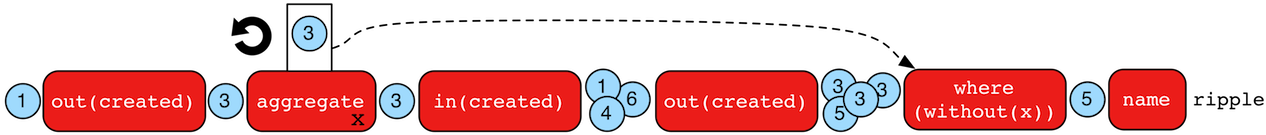The `aggregate()`-step (sideEffect) is used to aggregate all the objects at a particular point of traversal into a `Collection`. The step uses eager evaluation in that no objects continue on until all previous objects have been fully aggregated (as opposed to `store()` which lazily fills a collection). The eager evaluation nature is crucial in situations where everything at a particular point is required for future computation. An example is provided below.

``````gremlin> g.V(1).out('created') //1\
==>v
gremlin> g.V(1).out('created').aggregate('x') //2\
==>v
gremlin> g.V(1).out('created').aggregate('x').in('created') //3\
==>v
==>v
==>v
gremlin> g.V(1).out('created').aggregate('x').in('created').out('created') //4\
==>v
==>v
==>v
==>v
gremlin> g.V(1).out('created').aggregate('x').in('created').out('created').
where(without('x')).values('name') //5\
==>ripple``````
``````g.V(1).out('created') //1\
g.V(1).out('created').aggregate('x') //2\
g.V(1).out('created').aggregate('x').in('created') //3\
g.V(1).out('created').aggregate('x').in('created').out('created') //4\
g.V(1).out('created').aggregate('x').in('created').out('created').
where(without('x')).values('name') //5``````
1. What has marko created?

2. Aggregate all his creations.

3. Who are marko’s collaborators?

4. What have marko’s collaborators created?

5. What have marko’s collaborators created that he hasn’t created?

In recommendation systems, the above pattern is used:

`"What has userA liked? Who else has liked those things? What have they liked that userA hasn't already liked?"`

Finally, `aggregate()`-step can be modulated via `by()`-projection.

``````gremlin> g.V().out('knows').aggregate('x').cap('x')
==>[v,v]
gremlin> g.V().out('knows').aggregate('x').by('name').cap('x')
``````g.V().out('knows').aggregate('x').cap('x')
g.V().out('knows').aggregate('x').by('name').cap('x')``````

### And Step

The `and()`-step ensures that all provided traversals yield a result (filter). Please see `or()` for or-semantics.

 Python The term `and` is a reserved word in Python, and therefore must be referred to in Gremlin with `and_()`.
``````gremlin> g.V().and(
outE('knows'),
values('age').is(lt(30))).
values('name')
==>marko``````
``````g.V().and(
outE('knows'),
values('age').is(lt(30))).
values('name')``````

The `and()`-step can take an arbitrary number of traversals. All traversals must produce at least one output for the original traverser to pass to the next step.

An infix notation can be used as well.

``````gremlin> g.V().where(outE('created').and().outE('knows')).values('name')
==>marko``````
``g.V().where(outE('created').and().outE('knows')).values('name')``

### As Step

The `as()`-step is not a real step, but a "step modulator" similar to `by()` and `option()`. With `as()`, it is possible to provide a label to the step that can later be accessed by steps and data structures that make use of such labels — e.g., `select()`, `match()`, and path.

 Groovy The term `as` is a reserved word in Groovy, and when therefore used as part of an anonymous traversal must be referred to in Gremlin with the double underscore `__.as()`.
 Python The term `as` is a reserved word in Python, and therefore must be referred to in Gremlin with `as_()`.
``````gremlin> g.V().as('a').out('created').as('b').select('a','b') //1\
==>[a:v,b:v]
==>[a:v,b:v]
==>[a:v,b:v]
==>[a:v,b:v]
gremlin> g.V().as('a').out('created').as('b').select('a','b').by('name') //2\
==>[a:marko,b:lop]
==>[a:josh,b:ripple]
==>[a:josh,b:lop]
==>[a:peter,b:lop]``````
``````g.V().as('a').out('created').as('b').select('a','b') //1\
g.V().as('a').out('created').as('b').select('a','b').by('name') //2``````
1. Select the objects labeled "a" and "b" from the path.

2. Select the objects labeled "a" and "b" from the path and, for each object, project its name value.

A step can have any number of labels associated with it. This is useful for referencing the same step multiple times in a future step.

``````gremlin> g.V().hasLabel('software').as('a','b','c').
select('a','b','c').
by('name').
by('lang').
by(__.in('created').values('name').fold())
==>[a:lop,b:java,c:[marko,josh,peter]]
==>[a:ripple,b:java,c:[josh]]``````
``````g.V().hasLabel('software').as('a','b','c').
select('a','b','c').
by('name').
by('lang').
by(__.in('created').values('name').fold())``````

### Barrier Step

The `barrier()`-step (barrier) turns the lazy traversal pipeline into a bulk-synchronous pipeline. This step is useful in the following situations:

• When everything prior to `barrier()` needs to be executed before moving onto the steps after the `barrier()` (i.e. ordering).

• When "stalling" the traversal may lead to a "bulking optimization" in traversals that repeatedly touch many of the same elements (i.e. optimizing).

``````gremlin> g.V().sideEffect{println "first: \${it}"}.sideEffect{println "second: \${it}"}.iterate()
first: v
second: v
first: v
second: v
first: v
second: v
first: v
second: v
first: v
second: v
first: v
second: v
gremlin> g.V().sideEffect{println "first: \${it}"}.barrier().sideEffect{println "second: \${it}"}.iterate()
first: v
first: v
first: v
first: v
first: v
first: v
second: v
second: v
second: v
second: v
second: v
second: v``````
``````g.V().sideEffect{println "first: \${it}"}.sideEffect{println "second: \${it}"}.iterate()
g.V().sideEffect{println "first: \${it}"}.barrier().sideEffect{println "second: \${it}"}.iterate()``````

The theory behind a "bulking optimization" is simple. If there are one million traversers at vertex 1, then there is no need to calculate one million `both()`-computations. Instead, represent those one million traversers as a single traverser with a `Traverser.bulk()` equal to one million and execute `both()` once. A bulking optimization example is made more salient on a larger graph. Therefore, the example below leverages the Grateful Dead graph.

``````gremlin> graph = TinkerGraph.open()
==>tinkergraph[vertices:0 edges:0]
gremlin> g = graph.traversal()
==>graphtraversalsource[tinkergraph[vertices:0 edges:0], standard]
gremlin> g = graph.traversal().withoutStrategies(LazyBarrierStrategy) //1\
==>graphtraversalsource[tinkergraph[vertices:808 edges:8049], standard]
gremlin> clockWithResult(1){g.V().both().both().both().count().next()} //2\
==>10419.126311
==>126653966
gremlin> clockWithResult(1){g.V().repeat(both()).times(3).count().next()} //3\
==>32.934236
==>126653966
gremlin> clockWithResult(1){g.V().both().barrier().both().barrier().both().barrier().count().next()} //4\
==>14.656827999999999
==>126653966``````
``````graph = TinkerGraph.open()
g = graph.traversal()
g = graph.traversal().withoutStrategies(LazyBarrierStrategy) //1\
clockWithResult(1){g.V().both().both().both().count().next()} //2\
clockWithResult(1){g.V().repeat(both()).times(3).count().next()} //3\
clockWithResult(1){g.V().both().barrier().both().barrier().both().barrier().count().next()} //4``````
1. Explicitly remove `LazyBarrierStrategy` which yields a bulking optimization.

2. A non-bulking traversal where each traverser is processed.

3. Each traverser entering `repeat()` has its recursion bulked.

4. A bulking traversal where implicit traversers are not processed.

If `barrier()` is provided an integer argument, then the barrier will only hold `n`-number of unique traversers in its barrier before draining the aggregated traversers to the next step. This is useful in the aforementioned bulking optimization scenario with the added benefit of reducing the risk of an out-of-memory exception.

`LazyBarrierStrategy` inserts `barrier()`-steps into a traversal where appropriate in order to gain the "bulking optimization."

``````gremlin> graph = TinkerGraph.open()
==>tinkergraph[vertices:0 edges:0]
gremlin> g = graph.traversal() //1\
==>graphtraversalsource[tinkergraph[vertices:0 edges:0], standard]
gremlin> clockWithResult(1){g.V().both().both().both().count().next()}
==>9.826167
==>126653966
gremlin> g.V().both().both().both().count().iterate().toString() //2\
==>[TinkerGraphStep(vertex,[]), VertexStep(BOTH,vertex), NoOpBarrierStep(2500), VertexStep(BOTH,vertex), NoOpBarrierStep(2500), VertexStep(BOTH,edge), CountGlobalStep, NoneStep]``````
``````graph = TinkerGraph.open()
g = graph.traversal() //1\
clockWithResult(1){g.V().both().both().both().count().next()}
g.V().both().both().both().count().iterate().toString()  //2``````
1. `LazyBarrierStrategy` is a default strategy and thus, does not need to be explicitly activated.

2. With `LazyBarrierStrategy` activated, `barrier()`-steps are automatically inserted where appropriate.

### By Step

The `by()`-step is not an actual step, but instead is a "step-modulator" similar to `as()` and `option()`. If a step is able to accept traversals, functions, comparators, etc. then `by()` is the means by which they are added. The general pattern is `step().by()…​by()`. Some steps can only accept one `by()` while others can take an arbitrary amount.

``````gremlin> g.V().group().by(bothE().count()) //1\
==>[1:[v,v,v],3:[v,v,v]]
gremlin> g.V().group().by(bothE().count()).by('name') //2\
gremlin> g.V().group().by(bothE().count()).by(count()) //3\
==>[1:3,3:3]``````
``````g.V().group().by(bothE().count()) //1\
g.V().group().by(bothE().count()).by('name') //2\
g.V().group().by(bothE().count()).by(count())  //3``````
1. `by(outE().count())` will group the elements by their edge count (traversal).

2. `by('name')` will process the grouped elements by their name (element property projection).

3. `by(count())` will count the number of elements in each group (traversal).

The following steps all support `by()`-modulation. Note that the semantics of such modulation should be understood on a step-by-step level and thus, as discussed in their respective section of the documentation.

### Cap Step

The `cap()`-step (barrier) iterates the traversal up to itself and emits the sideEffect referenced by the provided key. If multiple keys are provided, then a `Map<String,Object>` of sideEffects is emitted.

``````gremlin> g.V().groupCount('a').by(label).cap('a') //1\
==>[software:2,person:4]
gremlin> g.V().groupCount('a').by(label).groupCount('b').by(outE().count()).cap('a','b') //2\
==>[a:[software:2,person:4],b:[0:3,1:1,2:1,3:1]]``````
``````g.V().groupCount('a').by(label).cap('a') //1\
g.V().groupCount('a').by(label).groupCount('b').by(outE().count()).cap('a','b')   //2``````
1. Group and count vertices by their label. Emit the side effect labeled 'a', which is the group count by label.

2. Same as statement 1, but also emit the side effect labeled 'b' which groups vertices by the number of out edges.

### Choose Step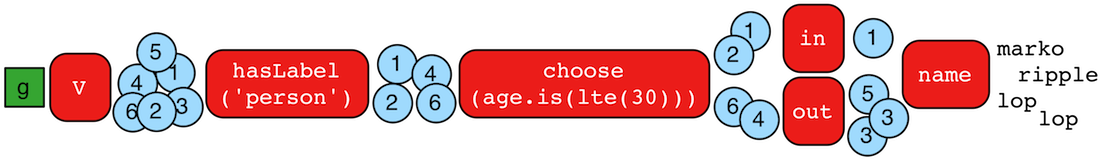The `choose()`-step (branch) routes the current traverser to a particular traversal branch option. With `choose()`, it is possible to implement if/then/else-semantics as well as more complicated selections.

``````gremlin> g.V().hasLabel('person').
choose(values('age').is(lte(30)),
__.in(),
__.out()).values('name') //1\
==>marko
==>ripple
==>lop
==>lop
gremlin> g.V().hasLabel('person').
choose(values('age')).
option(27, __.in()).
option(32, __.out()).values('name') //2\
==>marko
==>ripple
==>lop``````
``````g.V().hasLabel('person').
choose(values('age').is(lte(30)),
__.in(),
__.out()).values('name') //1\
g.V().hasLabel('person').
choose(values('age')).
option(27, __.in()).
option(32, __.out()).values('name') //2``````
1. If the traversal yields an element, then do `in`, else do `out` (i.e. true/false-based option selection).

2. Use the result of the traversal as a key to the map of traversal options (i.e. value-based option selection).

If the "false"-branch is not provided, then if/then-semantics are implemented.

``````gremlin> g.V().choose(hasLabel('person'), out('created')).values('name') //1\
==>lop
==>lop
==>ripple
==>lop
==>ripple
==>lop
gremlin> g.V().choose(hasLabel('person'), out('created'), identity()).values('name') //2\
==>lop
==>lop
==>ripple
==>lop
==>ripple
==>lop``````
``````g.V().choose(hasLabel('person'), out('created')).values('name') //1\
g.V().choose(hasLabel('person'), out('created'), identity()).values('name') //2``````
1. If the vertex is a person, emit the vertices they created, else emit the vertex.

2. If/then/else with an `identity()` on the false-branch is equivalent to if/then with no false-branch.

Note that `choose()` can have an arbitrary number of options and moreover, can take an anonymous traversal as its choice function.

``````gremlin> g.V().hasLabel('person').
choose(values('name')).
option('marko', values('age')).
option('josh', values('name')).
option('peter', label())
==>29
==>josh
==>person``````
``````g.V().hasLabel('person').
choose(values('name')).
option('marko', values('age')).
option('josh', values('name')).
option('peter', label())``````

The `choose()`-step can leverage the `Pick.none` option match. For anything that does not match a specified option, the `none`-option is taken.

``````gremlin> g.V().hasLabel('person').
choose(values('name')).
option('marko', values('age')).
option(none, values('name'))
==>29
==>josh
==>peter``````
``````g.V().hasLabel('person').
choose(values('name')).
option('marko', values('age')).
option(none, values('name'))``````

### Coalesce Step

The `coalesce()`-step evaluates the provided traversals in order and returns the first traversal that emits at least one element.

``````gremlin> g.V(1).coalesce(outE('knows'), outE('created')).inV().path().by('name').by(label)
==>[marko,knows,josh]
gremlin> g.V(1).coalesce(outE('created'), outE('knows')).inV().path().by('name').by(label)
==>[marko,created,lop]
gremlin> g.V(1).property('nickname', 'okram')
==>v
gremlin> g.V().hasLabel('person').coalesce(values('nickname'), values('name'))
==>okram
==>josh
==>peter``````
``````g.V(1).coalesce(outE('knows'), outE('created')).inV().path().by('name').by(label)
g.V(1).coalesce(outE('created'), outE('knows')).inV().path().by('name').by(label)
g.V(1).property('nickname', 'okram')
g.V().hasLabel('person').coalesce(values('nickname'), values('name'))``````

### Coin Step

To randomly filter out a traverser, use the `coin()`-step (filter). The provided double argument biases the "coin toss."

``````gremlin> g.V().coin(0.5)
==>v
==>v
==>v
gremlin> g.V().coin(0.0)
gremlin> g.V().coin(1.0)
==>v
==>v
==>v
==>v
==>v
==>v``````
``````g.V().coin(0.5)
g.V().coin(0.0)
g.V().coin(1.0)``````

### ConnectedComponent Step

The `connectedComponent()` step performs a computation to identify Connected Component instances in a graph. When this step completes, the vertices will be labelled with a component identifier to denote the component to which they are associated.

 Important The `connectedComponent()`-step is a `VertexComputing`-step and as such, can only be used against a graph that supports `GraphComputer` (OLAP).
``````gremlin> g = graph.traversal().withComputer()
==>graphtraversalsource[tinkergraph[vertices:6 edges:6], graphcomputer]
gremlin> g.V().
connectedComponent().
with(ConnectedComponent.propertyName, 'component').
project('name','component').
by('name').
by('component')
==>[name:lop,component:1]
==>[name:marko,component:1]
==>[name:josh,component:1]
==>[name:ripple,component:1]
==>[name:peter,component:1]
gremlin> g.V().hasLabel('person').
connectedComponent().
with(ConnectedComponent.propertyName, 'component').
with(ConnectedComponent.edges, outE('knows')).
project('name','component').
by('name').
by('component')
==>[name:marko,component:1]
==>[name:josh,component:1]
==>[name:peter,component:6]``````
``````g = graph.traversal().withComputer()
g.V().
connectedComponent().
with(ConnectedComponent.propertyName, 'component').
project('name','component').
by('name').
by('component')
g.V().hasLabel('person').
connectedComponent().
with(ConnectedComponent.propertyName, 'component').
with(ConnectedComponent.edges, outE('knows')).
project('name','component').
by('name').
by('component')``````

Note the use of the `with()` modulating step which provides configuration options to the algorithm. It takes configuration keys from the `ConnectedComponent` class and is automatically imported to the Gremlin Console.

### Constant Step

To specify a constant value for a traverser, use the `constant()`-step (map). This is often useful with conditional steps like `choose()`-step or `coalesce()`-step.

``````gremlin> g.V().choose(hasLabel('person'),
values('name'),
constant('inhuman')) //1\
==>marko
==>inhuman
==>josh
==>inhuman
==>peter
gremlin> g.V().coalesce(
hasLabel('person').values('name'),
constant('inhuman')) //2\
==>marko
==>inhuman
==>josh
==>inhuman
==>peter``````
``````g.V().choose(hasLabel('person'),
values('name'),
constant('inhuman')) //1\
g.V().coalesce(
hasLabel('person').values('name'),
constant('inhuman')) //2``````
1. Show the names of people, but show "inhuman" for other vertices.

2. Same as statement 1 (unless there is a person vertex with no name).

### Count Step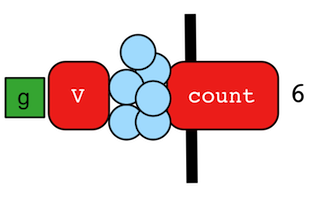The `count()`-step (map) counts the total number of represented traversers in the streams (i.e. the bulk count).

``````gremlin> g.V().count()
==>6
gremlin> g.V().hasLabel('person').count()
==>4
gremlin> g.V().hasLabel('person').outE('created').count().path() //1\
==>
gremlin> g.V().hasLabel('person').outE('created').count().map {it.get() * 10}.path() //2\
==>[4,40]``````
``````g.V().count()
g.V().hasLabel('person').count()
g.V().hasLabel('person').outE('created').count().path() //1\
g.V().hasLabel('person').outE('created').count().map {it.get() * 10}.path() //2``````
1. `count()`-step is a reducing barrier step meaning that all of the previous traversers are folded into a new traverser.

2. The path of the traverser emanating from `count()` starts at `count()`.

 Important `count(local)` counts the current, local object (not the objects in the traversal stream). This works for `Collection`- and `Map`-type objects. For any other object, a count of 1 is returned.

### CyclicPath Step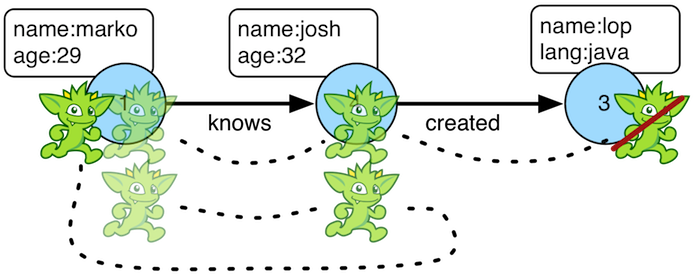Each traverser maintains its history through the traversal over the graph — i.e. its path. If it is important that the traverser repeat its course, then `cyclic()`-path should be used (filter). The step analyzes the path of the traverser thus far and if there are any repeats, the traverser is filtered out over the traversal computation. If non-cyclic behavior is desired, see `simplePath()`.

``````gremlin> g.V(1).both().both()
==>v
==>v
==>v
==>v
==>v
==>v
==>v
gremlin> g.V(1).both().both().cyclicPath()
==>v
==>v
==>v
gremlin> g.V(1).both().both().cyclicPath().path()
==>[v,v,v]
==>[v,v,v]
==>[v,v,v]
gremlin> g.V(1).as('a').out('created').as('b').
in('created').as('c').
cyclicPath().
path()
==>[v,v,v]
gremlin> g.V(1).as('a').out('created').as('b').
in('created').as('c').
cyclicPath().from('a').to('b').
path()``````
``````g.V(1).both().both()
g.V(1).both().both().cyclicPath()
g.V(1).both().both().cyclicPath().path()
g.V(1).as('a').out('created').as('b').
in('created').as('c').
cyclicPath().
path()
g.V(1).as('a').out('created').as('b').
in('created').as('c').
cyclicPath().from('a').to('b').
path()``````

### Dedup Step

With `dedup()`-step (filter), repeatedly seen objects are removed from the traversal stream. Note that if a traverser’s bulk is greater than 1, then it is set to 1 before being emitted.

``````gremlin> g.V().values('lang')
==>java
==>java
gremlin> g.V().values('lang').dedup()
==>java
gremlin> g.V(1).repeat(bothE('created').dedup().otherV()).emit().path() //1\
==>[v,e[1-created->3],v]
==>[v,e[1-created->3],v,e[4-created->3],v]
==>[v,e[1-created->3],v,e[6-created->3],v]
==>[v,e[1-created->3],v,e[4-created->3],v,e[4-created->5],v]``````
``````g.V().values('lang')
g.V().values('lang').dedup()
g.V(1).repeat(bothE('created').dedup().otherV()).emit().path() //1``````
1. Traverse all `created` edges, but don’t touch any edge twice.

If a by-step modulation is provided to `dedup()`, then the object is processed accordingly prior to determining if it has been seen or not.

``````gremlin> g.V().valueMap('name').with(WithOptions.tokens)
==>[id:1,label:person,name:[marko]]
==>[id:3,label:software,name:[lop]]
==>[id:4,label:person,name:[josh]]
==>[id:5,label:software,name:[ripple]]
==>[id:6,label:person,name:[peter]]
gremlin> g.V().dedup().by(label).values('name')
==>marko
==>lop``````
``````g.V().valueMap('name').with(WithOptions.tokens)
g.V().dedup().by(label).values('name')``````

Finally, if `dedup()` is provided an array of strings, then it will ensure that the de-duplication is not with respect to the current traverser object, but to the path history of the traverser.

``````gremlin> g.V().as('a').out('created').as('b').in('created').as('c').select('a','b','c')
==>[a:v,b:v,c:v]
==>[a:v,b:v,c:v]
==>[a:v,b:v,c:v]
==>[a:v,b:v,c:v]
==>[a:v,b:v,c:v]
==>[a:v,b:v,c:v]
==>[a:v,b:v,c:v]
==>[a:v,b:v,c:v]
==>[a:v,b:v,c:v]
==>[a:v,b:v,c:v]
gremlin> g.V().as('a').out('created').as('b').in('created').as('c').dedup('a','b').select('a','b','c') //1\
==>[a:v,b:v,c:v]
==>[a:v,b:v,c:v]
==>[a:v,b:v,c:v]
==>[a:v,b:v,c:v]``````
``````g.V().as('a').out('created').as('b').in('created').as('c').select('a','b','c')
g.V().as('a').out('created').as('b').in('created').as('c').dedup('a','b').select('a','b','c') //1``````
1. If the current `a` and `b` combination has been seen previously, then filter the traverser.

### Drop Step

The `drop()`-step (filter/sideEffect) is used to remove element and properties from the graph (i.e. remove). It is a filter step because the traversal yields no outgoing objects.

``````gremlin> g.V().outE().drop()
gremlin> g.E()
gremlin> g.V().properties('name').drop()
gremlin> g.V().valueMap()
==>[age:]
==>[age:]
==>[lang:[java]]
==>[age:]
==>[lang:[java]]
==>[age:]
gremlin> g.V().drop()
gremlin> g.V()``````
``````g.V().outE().drop()
g.E()
g.V().properties('name').drop()
g.V().valueMap()
g.V().drop()
g.V()``````

### Emit Step

The `emit`-step is not an actual step, but is instead a step modulator for `repeat()` (find more documentation on the `emit()` there).

### Explain Step

The `explain()`-step (terminal) will return a `TraversalExplanation`. A traversal explanation details how the traversal (prior to `explain()`) will be compiled given the registered traversal strategies. A `TraversalExplanation` has a `toString()` representation with 3-columns. The first column is the traversal strategy being applied. The second column is the traversal strategy category: [D]ecoration, [O]ptimization, [P]rovider optimization, [F]inalization, and [V]erification. Finally, the third column is the state of the traversal post strategy application. The final traversal is the resultant execution plan.

``````gremlin> g.V().hasLabel('person').outE().identity().inV().count().is(gt(5)).explain()
==>Traversal Explanation
=====================================================================================================================================================================================================
Original Traversal                 [GraphStep(vertex,[]), HasStep([~label.eq(person)]), VertexStep(OUT,edge), IdentityStep, EdgeVertexStep(IN), CountGlobalStep, IsStep(gt(5))]

ConnectiveStrategy           [D]   [GraphStep(vertex,[]), HasStep([~label.eq(person)]), VertexStep(OUT,edge), IdentityStep, EdgeVertexStep(IN), CountGlobalStep, IsStep(gt(5))]
EarlyLimitStrategy           [O]   [GraphStep(vertex,[]), HasStep([~label.eq(person)]), VertexStep(OUT,edge), IdentityStep, EdgeVertexStep(IN), CountGlobalStep, IsStep(gt(5))]
MatchPredicateStrategy       [O]   [GraphStep(vertex,[]), HasStep([~label.eq(person)]), VertexStep(OUT,edge), IdentityStep, EdgeVertexStep(IN), CountGlobalStep, IsStep(gt(5))]
FilterRankingStrategy        [O]   [GraphStep(vertex,[]), HasStep([~label.eq(person)]), VertexStep(OUT,edge), IdentityStep, EdgeVertexStep(IN), CountGlobalStep, IsStep(gt(5))]
InlineFilterStrategy         [O]   [GraphStep(vertex,[]), HasStep([~label.eq(person)]), VertexStep(OUT,edge), IdentityStep, EdgeVertexStep(IN), CountGlobalStep, IsStep(gt(5))]
IncidentToAdjacentStrategy   [O]   [GraphStep(vertex,[]), HasStep([~label.eq(person)]), VertexStep(OUT,edge), IdentityStep, EdgeVertexStep(IN), CountGlobalStep, IsStep(gt(5))]
AdjacentToIncidentStrategy   [O]   [GraphStep(vertex,[]), HasStep([~label.eq(person)]), VertexStep(OUT,edge), IdentityStep, EdgeVertexStep(IN), CountGlobalStep, IsStep(gt(5))]
RepeatUnrollStrategy         [O]   [GraphStep(vertex,[]), HasStep([~label.eq(person)]), VertexStep(OUT,edge), IdentityStep, EdgeVertexStep(IN), CountGlobalStep, IsStep(gt(5))]
CountStrategy                [O]   [GraphStep(vertex,[]), HasStep([~label.eq(person)]), VertexStep(OUT,edge), IdentityStep, EdgeVertexStep(IN), RangeGlobalStep(0,6), CountGlobalStep, IsStep(gt(5))]
PathRetractionStrategy       [O]   [GraphStep(vertex,[]), HasStep([~label.eq(person)]), VertexStep(OUT,edge), IdentityStep, EdgeVertexStep(IN), RangeGlobalStep(0,6), CountGlobalStep, IsStep(gt(5))]
LazyBarrierStrategy          [O]   [GraphStep(vertex,[]), HasStep([~label.eq(person)]), VertexStep(OUT,edge), IdentityStep, EdgeVertexStep(IN), RangeGlobalStep(0,6), CountGlobalStep, IsStep(gt(5))]
TinkerGraphCountStrategy     [P]   [GraphStep(vertex,[]), HasStep([~label.eq(person)]), VertexStep(OUT,edge), IdentityStep, EdgeVertexStep(IN), RangeGlobalStep(0,6), CountGlobalStep, IsStep(gt(5))]
TinkerGraphStepStrategy      [P]   [TinkerGraphStep(vertex,[~label.eq(person)]), VertexStep(OUT,edge), IdentityStep, EdgeVertexStep(IN), RangeGlobalStep(0,6), CountGlobalStep, IsStep(gt(5))]
ProfileStrategy              [F]   [TinkerGraphStep(vertex,[~label.eq(person)]), VertexStep(OUT,edge), IdentityStep, EdgeVertexStep(IN), RangeGlobalStep(0,6), CountGlobalStep, IsStep(gt(5))]
StandardVerificationStrategy [V]   [TinkerGraphStep(vertex,[~label.eq(person)]), VertexStep(OUT,edge), IdentityStep, EdgeVertexStep(IN), RangeGlobalStep(0,6), CountGlobalStep, IsStep(gt(5))]

Final Traversal                    [TinkerGraphStep(vertex,[~label.eq(person)]), VertexStep(OUT,edge), IdentityStep, EdgeVertexStep(IN), RangeGlobalStep(0,6), CountGlobalStep, IsStep(gt(5))]``````
``g.V().hasLabel('person').outE().identity().inV().count().is(gt(5)).explain()``

For traversal profiling information, please see `profile()`-step.

### Fold Step

There are situations when the traversal stream needs a "barrier" to aggregate all the objects and emit a computation that is a function of the aggregate. The `fold()`-step (map) is one particular instance of this. Please see `unfold()`-step for the inverse functionality.

``````gremlin> g.V(1).out('knows').values('name')
==>josh
gremlin> g.V(1).out('knows').values('name').fold() //1\
gremlin> g.V(1).out('knows').values('name').fold().next().getClass() //2\
==>class java.util.ArrayList
gremlin> g.V(1).out('knows').values('name').fold(0) {a,b -> a + b.length()} //3\
==>9
gremlin> g.V().values('age').fold(0) {a,b -> a + b} //4\
==>123
gremlin> g.V().values('age').fold(0, sum) //5\
==>123
gremlin> g.V().values('age').sum() //6\
==>123``````
``````g.V(1).out('knows').values('name')
g.V(1).out('knows').values('name').fold() //1\
g.V(1).out('knows').values('name').fold().next().getClass() //2\
g.V(1).out('knows').values('name').fold(0) {a,b -> a + b.length()} //3\
g.V().values('age').fold(0) {a,b -> a + b} //4\
g.V().values('age').fold(0, sum) //5\
g.V().values('age').sum() //6``````
1. A parameterless `fold()` will aggregate all the objects into a list and then emit the list.

2. A verification of the type of list returned.

3. `fold()` can be provided two arguments —  a seed value and a reduce bi-function ("vadas" is 5 characters + "josh" with 4 characters).

4. What is the total age of the people in the graph?

5. The same as before, but using a built-in bi-function.

6. The same as before, but using the `sum()`-step.

### From Step

The `from()`-step is not an actual step, but instead is a "step-modulator" similar to `as()` and `by()`. If a step is able to accept traversals or strings then `from()` is the means by which they are added. The general pattern is `step().from()`. See `to()`-step.

The list of steps that support `from()`-modulation are: `simplePath()`, `cyclicPath()`, `path()`, and `addE()`.

 Javascript The term `from` is a reserved word in Javascript, and therefore must be referred to in Gremlin with `from_()`.
 Python The term `from` is a reserved word in Python, and therefore must be referred to in Gremlin with `from_()`.

### Graph Step

The `V()`-step is usually used to start a `GraphTraversal`, but can also be used mid-traversal.

``````gremlin> g.V().has('name', within('marko', 'vadas', 'josh')).as('person').
==>e[1-uses->3]
==>e[1-uses->5]
==>e[2-uses->3]
==>e[2-uses->5]
==>e[4-uses->3]
==>e[4-uses->5]``````
``````g.V().has('name', within('marko', 'vadas', 'josh')).as('person').
 Note Whether a mid-traversal `V()` uses an index or not, depends on a) whether suitable index exists and b) if the particular graph system provider implemented this functionality.
``````gremlin> g.V().has('name', within('marko', 'vadas', 'josh')).as('person').
``````g.V().has('name', within('marko', 'vadas', 'josh')).as('person').
1. Normally the `V()`-step will iterate over all vertices. However, graph strategies can fold `HasContainer’s into a `GraphStep` to allow index lookups.

2. Whether the graph system provider supports mid-traversal `V()` index lookups or not can easily be determined by inspecting the `toString()` output of the iterated traversal. If `has` conditions were folded into the `V()`-step, an index - if one exists - will be used.

### Group Step

As traversers propagate across a graph as defined by a traversal, sideEffect computations are sometimes required. That is, the actual path taken or the current location of a traverser is not the ultimate output of the computation, but some other representation of the traversal. The `group()`-step (map/sideEffect) is one such sideEffect that organizes the objects according to some function of the object. Then, if required, that organization (a list) is reduced. An example is provided below.

``````gremlin> g.V().group().by(label) //1\
==>[software:[v,v],person:[v,v,v,v]]
gremlin> g.V().group().by(label).by('name') //2\
gremlin> g.V().group().by(label).by(count()) //3\
==>[software:2,person:4]``````
``````g.V().group().by(label) //1\
g.V().group().by(label).by('name') //2\
g.V().group().by(label).by(count()) //3``````
1. Group the vertices by their label.

2. For each vertex in the group, get their name.

3. For each grouping, what is its size?

The two projection parameters available to `group()` via `by()` are:

1. Key-projection: What feature of the object to group on (a function that yields the map key)?

2. Value-projection: What feature of the group to store in the key-list?

### GroupCount Step

When it is important to know how many times a particular object has been at a particular part of a traversal, `groupCount()`-step (map/sideEffect) is used.

`"What is the distribution of ages in the graph?"`
``````gremlin> g.V().hasLabel('person').values('age').groupCount()
==>[32:1,35:1,27:1,29:1]
gremlin> g.V().hasLabel('person').groupCount().by('age') //1\
==>[32:1,35:1,27:1,29:1]``````
``````g.V().hasLabel('person').values('age').groupCount()
g.V().hasLabel('person').groupCount().by('age') //1``````
1. You can also supply a pre-group projection, where the provided `by()`-modulation determines what to group the incoming object by.

There is one person that is 32, one person that is 35, one person that is 27, and one person that is 29.

`"Iteratively walk the graph and count the number of times you see the second letter of each name."`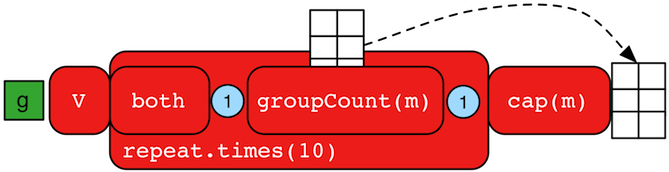``````gremlin> g.V().repeat(both().groupCount('m').by(label)).times(10).cap('m')
==>[software:19598,person:39196]``````
``g.V().repeat(both().groupCount('m').by(label)).times(10).cap('m')``

The above is interesting in that it demonstrates the use of referencing the internal `Map<Object,Long>` of `groupCount()` with a string variable. Given that `groupCount()` is a sideEffect-step, it simply passes the object it received to its output. Internal to `groupCount()`, the object’s count is incremented.

### Has Step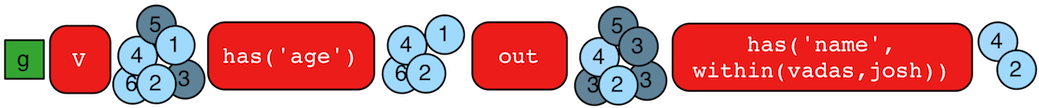It is possible to filter vertices, edges, and vertex properties based on their properties using `has()`-step (filter). There are numerous variations on `has()` including:

• `has(key,value)`: Remove the traverser if its element does not have the provided key/value property.

• `has(label, key, value)`: Remove the traverser if its element does not have the specified label and provided key/value property.

• `has(key,predicate)`: Remove the traverser if its element does not have a key value that satisfies the bi-predicate. For more information on predicates, please read A Note on Predicates.

• `hasLabel(labels…​)`: Remove the traverser if its element does not have any of the labels.

• `hasId(ids…​)`: Remove the traverser if its element does not have any of the ids.

• `hasKey(keys…​)`: Remove the traverser if the property does not have all of the provided keys.

• `hasValue(values…​)`: Remove the traverser if its property does not have all of the provided values.

• `has(key)`: Remove the traverser if its element does not have a value for the key.

• `hasNot(key)`: Remove the traverser if its element has a value for the key.

• `has(key, traversal)`: Remove the traverser if its object does not yield a result through the traversal off the property value.

``````gremlin> g.V().hasLabel('person')
==>v
==>v
==>v
==>v
==>v
==>v
outE().hasLabel('created')
==>e[4-created->5]
==>e[4-created->3]
gremlin> g.V().has('age',inside(20,30)).values('age') //1\
==>29
==>27
gremlin> g.V().has('age',outside(20,30)).values('age') //2\
==>32
==>35
gremlin> g.V().has('name',within('josh','marko')).valueMap() //3\
==>[name:[marko],age:]
==>[name:[josh],age:]
gremlin> g.V().has('name',without('josh','marko')).valueMap() //4\
==>[name:[lop],lang:[java]]
==>[name:[ripple],lang:[java]]
==>[name:[peter],age:]
gremlin> g.V().has('name',not(within('josh','marko'))).valueMap() //5\
==>[name:[lop],lang:[java]]
==>[name:[ripple],lang:[java]]
==>[name:[peter],age:]
==>29
==>27
==>32
==>35
gremlin> g.V().hasNot('age').values('name') //7\
==>lop
==>ripple``````
``````g.V().hasLabel('person')
outE().hasLabel('created')
g.V().has('age',inside(20,30)).values('age') //1\
g.V().has('age',outside(20,30)).values('age') //2\
g.V().has('name',within('josh','marko')).valueMap() //3\
g.V().has('name',without('josh','marko')).valueMap() //4\
g.V().has('name',not(within('josh','marko'))).valueMap() //5\
g.V().hasNot('age').values('name') //7``````
1. Find all vertices whose ages are between 20 (exclusive) and 30 (exclusive). In other words, the age must be greater than 20 and less than 30.

2. Find all vertices whose ages are not between 20 (inclusive) and 30 (inclusive). In other words, the age must be less than 20 or greater than 30.

3. Find all vertices whose names are exact matches to any names in the collection `[josh,marko]`, display all the key,value pairs for those vertices.

4. Find all vertices whose names are not in the collection `[josh,marko]`, display all the key,value pairs for those vertices.

5. Same as the prior example save using `not` on `within` to yield `without`.

6. Find all age-properties and emit their value.

7. Find all vertices that do not have an age-property and emit their name.

TinkerPop does not support a regular expression predicate, although specific graph databases that leverage TinkerPop may provide a partial match extension.

### Id Step

The `id()`-step (map) takes an `Element` and extracts its identifier from it.

``````gremlin> g.V().id()
==>1
==>2
==>3
==>4
==>5
==>6
gremlin> g.V(1).out().id().is(2)
==>2
gremlin> g.V(1).outE().id()
==>9
==>7
==>8
gremlin> g.V(1).properties().id()
==>0
==>1``````
``````g.V().id()
g.V(1).out().id().is(2)
g.V(1).outE().id()
g.V(1).properties().id()``````

### Identity Step

The `identity()`-step (map) is an identity function which maps the current object to itself.

``````gremlin> g.V().identity()
==>v
==>v
==>v
==>v
==>v
==>v``````
``g.V().identity()``

### Index Step

The `index()`-step (map) indexes each element in the current collection. If the current traverser’s value is not a collection, then it’s treated as a single-item collection. There are two indexers available, which can be chosen using the `with()` modulator. The list indexer (default) creates a list for each collection item, with the first item being the original element and the second element being the index. The map indexer created a linked hash map in which the index represents the key and the original item is used as the value.

``````gremlin> g.V().hasLabel("software").index() //1\
==>[[v,0]]
==>[[v,0]]
gremlin> g.V().hasLabel("software").values("name").fold().
order(Scope.local).
index().
unfold().
order().
by(__.tail(Scope.local, 1)) //2\
==>[lop,0]
==>[ripple,1]
gremlin> g.V().hasLabel("software").values("name").fold().
order(Scope.local).
index().
with(WithOptions.indexer, WithOptions.list).
unfold().
order().
by(__.tail(Scope.local, 1)) //3\
==>[lop,0]
==>[ripple,1]
gremlin> g.V().hasLabel("person").values("name").fold().
order(Scope.local).
index().
with(WithOptions.indexer, WithOptions.map) //4\
``````g.V().hasLabel("software").index() //1\
g.V().hasLabel("software").values("name").fold().
order(Scope.local).
index().
unfold().
order().
by(__.tail(Scope.local, 1)) //2\
g.V().hasLabel("software").values("name").fold().
order(Scope.local).
index().
with(WithOptions.indexer, WithOptions.list).
unfold().
order().
by(__.tail(Scope.local, 1)) //3\
g.V().hasLabel("person").values("name").fold().
order(Scope.local).
index().
with(WithOptions.indexer, WithOptions.map)  //4``````
1. Indexing non-collection items results in multiple indexed single-item collections.

2. Index all software names in their alphabetical order.

3. Same as statement 1, but with an explicitely specified list indexer.

4. Index all person names in their alphabetical order and store the result in an ordered map.

### Inject Step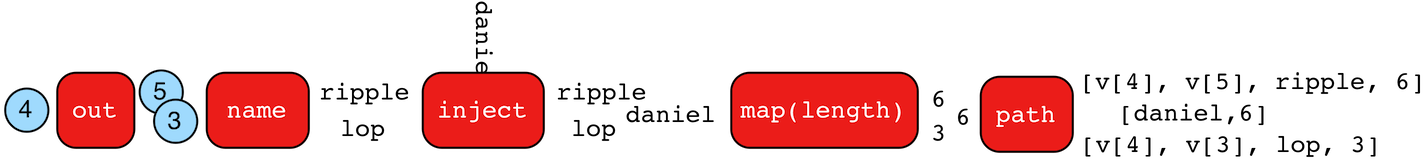The concept of "injectable steps" makes it possible to insert objects arbitrarily into a traversal stream. In general, `inject()`-step (sideEffect) exists and a few examples are provided below.

``````gremlin> g.V(4).out().values('name').inject('daniel')
==>daniel
==>ripple
==>lop
gremlin> g.V(4).out().values('name').inject('daniel').map {it.get().length()}
==>6
==>6
==>3
gremlin> g.V(4).out().values('name').inject('daniel').map {it.get().length()}.path()
==>[daniel,6]
==>[v,v,ripple,6]
==>[v,v,lop,3]``````
``````g.V(4).out().values('name').inject('daniel')
g.V(4).out().values('name').inject('daniel').map {it.get().length()}
g.V(4).out().values('name').inject('daniel').map {it.get().length()}.path()``````

In the last example above, note that the path starting with `daniel` is only of length 2. This is because the `daniel` string was inserted half-way in the traversal. Finally, a typical use case is provided below — when the start of the traversal is not a graph object.

``````gremlin> inject(1,2)
==>1
==>2
gremlin> inject(1,2).map {it.get() + 1}
==>2
==>3
gremlin> inject(1,2).map {it.get() + 1}.map {g.V(it.get()).next()}.values('name')
==>lop``````
``````inject(1,2)
inject(1,2).map {it.get() + 1}
inject(1,2).map {it.get() + 1}.map {g.V(it.get()).next()}.values('name')``````

### IO Step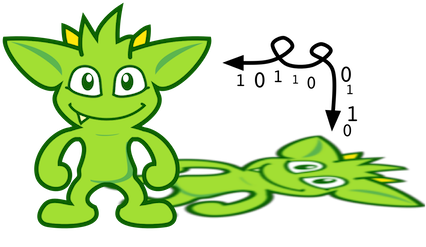The task of importing and exporting the data of `Graph` instances is the job of the `io()`-step. By default, TinkerPop supports three formats for importing and exporting graph data in GraphML, GraphSON, and Gryo.

 Note Additional documentation for TinkerPop IO formats can be found in the IO Reference.

By itself the `io()`-step merely configures the kind of importing and exporting that is going to occur and it is the follow-on call to the `read()` or `write()` step that determines which of those actions will execute. Therefore, a typical usage of the `io()`-step would look like this:

``````g.io(someInputFile).read().iterate()
g.io(someOutputFile).write().iterate()``````
 Important The commands above are still traversals and therefore require iteration to be executed, hence the use of `iterate()` as a termination step.

By default, the `io()`-step will try to detect the right file format using the file name extension. To gain greater control of the format use the `with()` step modulator to provide further information to `io()`. For example:

``````g.io(someInputFile).
g.io(someOutputFile).
with(IO.writer,IO.graphml).
write().iterate()``````

The `IO` class is a helper for the `io()`-step that provides expressions that can be used to help configure it and in this case it allows direct specification of the "reader" or "writer" to use. The "reader" actually refers to a `GraphReader` implementation and the "writer" refers to a `GraphWriter` implementation. The implementations of those interfaces provided by default are the standard TinkerPop implementations.

That default is an important point to consider for users. The default TinkerPop implementations are not designed with massive, complex, parallel bulk loading in mind. They are designed to do single-threaded, OLTP-style loading of data in the most generic way possible so as to accommodate the greatest number of graph databases out there. As such, from a reading perspective, they work best for small datasets (or perhaps medium datasets where memory is plentiful and time is not critical) that are loading to an empty graph - incremental loading is not supported. The story from the writing perspective is not that different in there are no parallel operations in play, however streaming the output to disk requires a single pass of the data without high memory requirements for larger datasets.

In general, TinkerPop recommends that users examine the native bulk import/export tools of the graph implementation that they choose. Those tools will often outperform the `io()`-step and perhaps be easier to use with a greater feature set. That said, graph providers do have the option to optimize `io()` to back it with their own import/export utilities and therefore the default behavior provided by TinkerPop described above might be overridden by the graph.

An excellent example of this lies in HadoopGraph with SparkGraphComputer which replaces the default single-threaded implementation with a more advanced OLAP style bulk import/export functionality internally using CloneVertexProgram. With this model, graphs of arbitrary size can be imported/exported assuming that there is a Hadoop `InputFormat` or `OutputFormat` to support it.

 Important Remote Gremlin Console users or Gremlin Language Variant (GLV) users (e.g. gremlin-python) who utilize the `io()`-step should recall that their `read()` or `write()` operation will occur on the server and not locally and therefore the file specified for import/export must be something accessible by the server.

GraphSON and Gryo formats are extensible allowing users and graph providers to extend supported serialization options. These extensions are exposed through `IoRegistry` implementations. To apply an `IoRegistry` use the `with()` option and the `IO.registry` key, where the value is either an actual `IoRegistry` instance or the fully qualified class name of one.

``````g.io(someInputFile).
with(IO.registry, TinkerIoRegistryV3d0.instance())
g.io(someOutputFile).
with(IO.writer,IO.graphson).
with(IO.registry, "org.apache.tinkerpop.gremlin.tinkergraph.structure.TinkerIoRegistryV3d0")
write().iterate()``````

GLVs will obviously always be forced to use the latter form as they can’t explicitly create an instance of an `IoRegistry` to pass to the server (nor are `IoRegistry` instances necessarily serializable).

The version of the formats (e.g. GraphSON 2.0 or 3.0) utilized by `io()` is determined entirely by the `IO.reader` and `IO.writer` configurations or their defaults. The defaults will always be the latest version for the current release of TinkerPop. It is also possible for graph providers to override these defaults, so consult the documentation of the underlying graph database in use for any details on that.

For more advanced configuration of `GraphReader` and `GraphWriter` operations (e.g. normalized output for GraphSON, disabling class registrations for Gryo, etc.) then construct the appropriate `GraphReader` and `GraphWriter` using the `build()` method on their implementations and use it directly. It can be passed directly to the `IO.reader` or `IO.writer` options. Obviously, these are JVM based operations and thus not available to GLVs as portable features.

#### GraphMLThe GraphML file format is a common XML-based representation of a graph. It is widely supported by graph-related tools and libraries making it a solid interchange format for TinkerPop. In other words, if the intent is to work with graph data in conjunction with applications outside of TinkerPop, GraphML may be the best choice to do that. Common use cases might be:

• Generate a graph using NetworkX, export it with GraphML and import it to TinkerPop.

• Produce a subgraph and export it to GraphML to be consumed by and visualized in Gephi.

• Migrate the data of an entire graph to a different graph database not supported by TinkerPop.

 Warning GraphML is a "lossy" format in that it only supports primitive values for properties and does not have support for `Graph` variables. It will use `toString` to serialize property values outside of those primitives.
 Warning GraphML as a specification allows for `` and `` elements to appear in any order. Most software that writes GraphML (including as TinkerPop’s `GraphMLWriter`) write `` elements before `` elements. However it is important to note that `GraphMLReader` will read this data in order and order can matter. This is because TinkerPop does not allow the vertex label to be changed after the vertex has been created. Therefore, if an `` element comes before the ``, the label on the vertex will be ignored. It is thus better to order `` elements in the GraphML to appear before all `` elements if vertex labels are important to the graph.
``````g.io("graph.xml").read().iterate()
g.io("graph.xml").write().iterate()``````
 Note If using GraphML generated from TinkerPop 2.x, read more about its incompatibilities in the Upgrade Documentation.

#### GraphSONGraphSON is a JSON-based format extended from earlier versions of TinkerPop. It is important to note that TinkerPop’s GraphSON is not backwards compatible with prior TinkerPop GraphSON versions. GraphSON has some support from graph-related application outside of TinkerPop, but it is generally best used in two cases:

• A text format of the graph or its elements is desired (e.g. debugging, usage in source control, etc.)

• The graph or its elements need to be consumed by code that is not JVM-based (e.g. JavaScript, Python, .NET, etc.)

``````g.io("graph.json").read().iterate()
g.io("graph.json").write().iterate()``````
 Note Additional documentation for GraphSON can be found in the IO Reference.

#### GryoKryo is a popular serialization package for the JVM. Gremlin-Kryo is a binary `Graph` serialization format for use on the JVM by JVM languages. It is designed to be space efficient, non-lossy and is promoted as the standard format to use when working with graph data inside of the TinkerPop stack. A list of common use cases is presented below:

• Migration from one Gremlin Structure implementation to another (e.g. `TinkerGraph` to `Neo4jGraph`)

• Serialization of individual graph elements to be sent over the network to another JVM.

• Backups of in-memory graphs or subgraphs.

 Warning When migrating between Gremlin Structure implementations, Kryo may not lose data, but it is important to consider the features of each `Graph` and whether or not the data types supported in one will be supported in the other. Failure to do so, may result in errors.
``````g.io("graph.kryo").read().iterate()
g.io("graph.kryo").write().iterate()``````

### Is Step

It is possible to filter scalar values using `is()`-step (filter).

 Python The term `is` is a reserved word in Python, and therefore must be referred to in Gremlin with `is_()`.
``````gremlin> g.V().values('age').is(32)
==>32
gremlin> g.V().values('age').is(lte(30))
==>29
==>27
gremlin> g.V().values('age').is(inside(30, 40))
==>32
==>35
gremlin> g.V().where(__.in('created').count().is(1)).values('name') //1\
==>ripple
gremlin> g.V().where(__.in('created').count().is(gte(2))).values('name') //2\
==>lop
gremlin> g.V().where(__.in('created').values('age').
mean().is(inside(30d, 35d))).values('name') //3\
==>lop
==>ripple``````
``````g.V().values('age').is(32)
g.V().values('age').is(lte(30))
g.V().values('age').is(inside(30, 40))
g.V().where(__.in('created').count().is(1)).values('name') //1\
g.V().where(__.in('created').count().is(gte(2))).values('name') //2\
g.V().where(__.in('created').values('age').
mean().is(inside(30d, 35d))).values('name') //3``````
1. Find projects having exactly one contributor.

2. Find projects having two or more contributors.

3. Find projects whose contributors average age is between 30 and 35.

### Key Step

The `key()`-step (map) takes a `Property` and extracts the key from it.

``````gremlin> g.V(1).properties().key()
==>name
==>location
==>location
==>location
==>location
gremlin> g.V(1).properties().properties().key()
==>startTime
==>endTime
==>startTime
==>endTime
==>startTime
==>endTime
==>startTime``````
``````g.V(1).properties().key()
g.V(1).properties().properties().key()``````

### Label Step

The `label()`-step (map) takes an `Element` and extracts its label from it.

``````gremlin> g.V().label()
==>person
==>person
==>software
==>person
==>software
==>person
gremlin> g.V(1).outE().label()
==>created
==>knows
==>knows
gremlin> g.V(1).properties().label()
==>name
==>age``````
``````g.V().label()
g.V(1).outE().label()
g.V(1).properties().label()``````

### Limit Step

The `limit()`-step is analogous to `range()`-step save that the lower end range is set to 0.

``````gremlin> g.V().limit(2)
==>v
==>v
gremlin> g.V().range(0, 2)
==>v
==>v``````
``````g.V().limit(2)
g.V().range(0, 2)``````

The `limit()`-step can also be applied with `Scope.local`, in which case it operates on the incoming collection. The examples below use the The Crew toy data set.

``````gremlin> g.V().valueMap().select('location').limit(local,2) //1\
==>[san diego,santa cruz]
==>[centreville,dulles]
==>[bremen,baltimore]
==>[spremberg,kaiserslautern]
gremlin> g.V().valueMap().limit(local, 1) //2\
==>[name:[marko]]
==>[name:[stephen]]
==>[name:[matthias]]
==>[name:[daniel]]
==>[name:[gremlin]]
==>[name:[tinkergraph]]``````
``````g.V().valueMap().select('location').limit(local,2) //1\
g.V().valueMap().limit(local, 1) //2``````
1. `List<String>` for each vertex containing the first two locations.

2. `Map<String, Object>` for each vertex, but containing only the first property value.

### Local Step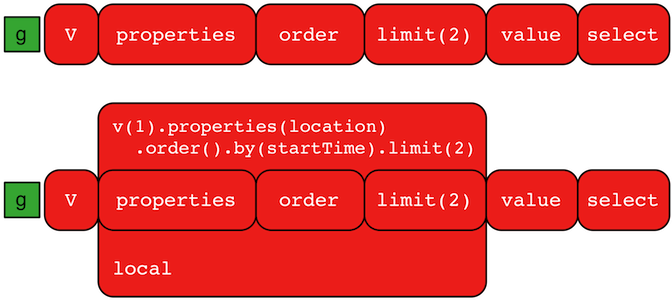A `GraphTraversal` operates on a continuous stream of objects. In many situations, it is important to operate on a single element within that stream. To do such object-local traversal computations, `local()`-step exists (branch). Note that the examples below use the The Crew toy data set.

``````gremlin> g.V().as('person').
properties('location').order().by('startTime',asc).limit(2).value().as('location').
select('person','location').by('name').by() //1\
==>[person:daniel,location:spremberg]
==>[person:stephen,location:centreville]
gremlin> g.V().as('person').
local(properties('location').order().by('startTime',asc).limit(2)).value().as('location').
select('person','location').by('name').by() //2\
==>[person:marko,location:san diego]
==>[person:marko,location:santa cruz]
==>[person:stephen,location:centreville]
==>[person:stephen,location:dulles]
==>[person:matthias,location:bremen]
==>[person:matthias,location:baltimore]
==>[person:daniel,location:spremberg]
==>[person:daniel,location:kaiserslautern]``````
``````g.V().as('person').
properties('location').order().by('startTime',asc).limit(2).value().as('location').
select('person','location').by('name').by() //1\
g.V().as('person').
local(properties('location').order().by('startTime',asc).limit(2)).value().as('location').
select('person','location').by('name').by() //2``````
1. Get the first two people and their respective location according to the most historic location start time.

2. For every person, get their two most historic locations.

The two traversals above look nearly identical save the inclusion of `local()` which wraps a section of the traversal in a object-local traversal. As such, the `order().by()` and the `limit()` refer to a particular object, not to the stream as a whole.

Local Step is quite similar in functionality to Flat Map Step where it can often be confused. `local()` propagates the traverser through the internal traversal as is without splitting/cloning it. Thus, its a “global traversal” with local processing. Its use is subtle and primarily finds application in compilation optimizations (i.e. when writing `TraversalStrategy` implementations. As another example consider:

``````gremlin> g.V().both().barrier().flatMap(groupCount().by("name"))
==>[lop:1]
==>[lop:1]
==>[lop:1]
==>[josh:1]
==>[josh:1]
==>[josh:1]
==>[marko:1]
==>[marko:1]
==>[marko:1]
==>[peter:1]
==>[ripple:1]
gremlin> g.V().both().barrier().local(groupCount().by("name"))
==>[lop:3]
==>[josh:3]
==>[marko:3]
==>[peter:1]
==>[ripple:1]``````
``````g.V().both().barrier().flatMap(groupCount().by("name"))
g.V().both().barrier().local(groupCount().by("name"))``````
 Warning The anonymous traversal of `local()` processes the current object "locally." In OLAP, where the atomic unit of computing is the vertex and its local "star graph," it is important that the anonymous traversal does not leave the confines of the vertex’s star graph. In other words, it can not traverse to an adjacent vertex’s properties or edges.

### Loops Step

The `loops()`-step (map) extracts the number of times the `Traverser` has gone through the current loop.

``````gremlin> g.V().emit(__.has("name", "marko").or().loops().is(2)).repeat(__.out()).values("name")
==>marko
==>ripple
==>lop``````
``g.V().emit(__.has("name", "marko").or().loops().is(2)).repeat(__.out()).values("name")``

### Match Step

The `match()`-step (map) provides a more declarative form of graph querying based on the notion of pattern matching. With `match()`, the user provides a collection of "traversal fragments," called patterns, that have variables defined that must hold true throughout the duration of the `match()`. When a traverser is in `match()`, a registered `MatchAlgorithm` analyzes the current state of the traverser (i.e. its history based on its path data), the runtime statistics of the traversal patterns, and returns a traversal-pattern that the traverser should try next. The default `MatchAlgorithm` provided is called `CountMatchAlgorithm` and it dynamically revises the pattern execution plan by sorting the patterns according to their filtering capabilities (i.e. largest set reduction patterns execute first). For very large graphs, where the developer is uncertain of the statistics of the graph (e.g. how many `knows`-edges vs. `worksFor`-edges exist in the graph), it is advantageous to use `match()`, as an optimal plan will be determined automatically. Furthermore, some queries are much easier to express via `match()` than with single-path traversals.

`"Who created a project named 'lop' that was also created by someone who is 29 years old? Return the two creators."`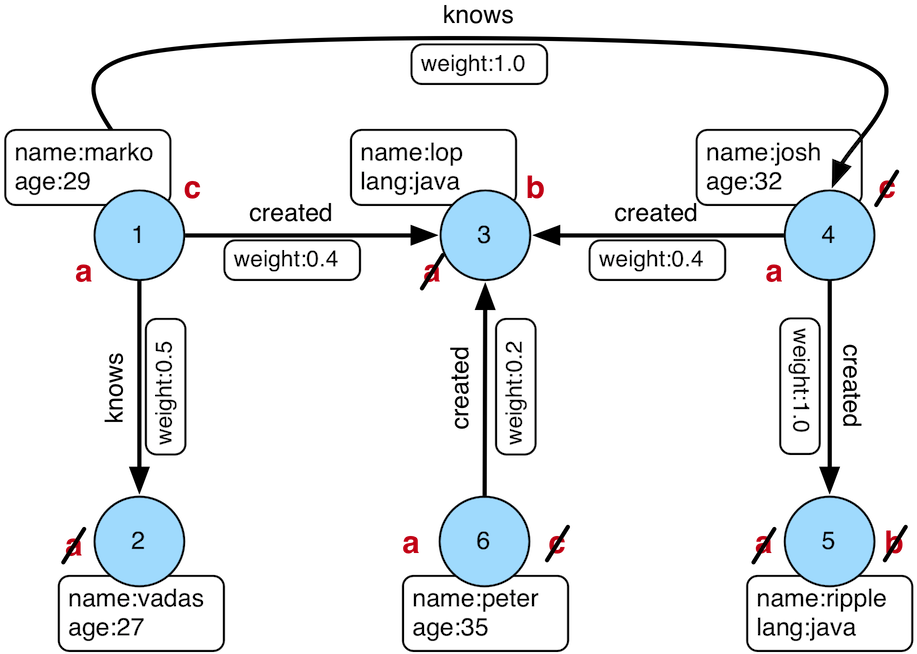``````gremlin> g.V().match(
__.as('a').out('created').as('b'),
__.as('b').has('name', 'lop'),
__.as('b').in('created').as('c'),
__.as('c').has('age', 29)).
select('a','c').by('name')
==>[a:marko,c:marko]
==>[a:josh,c:marko]
==>[a:peter,c:marko]``````
``````g.V().match(
__.as('a').out('created').as('b'),
__.as('b').has('name', 'lop'),
__.as('b').in('created').as('c'),
__.as('c').has('age', 29)).
select('a','c').by('name')``````

Note that the above can also be more concisely written as below which demonstrates that standard inner-traversals can be arbitrarily defined.

``````gremlin> g.V().match(
__.as('a').out('created').has('name', 'lop').as('b'),
__.as('b').in('created').has('age', 29).as('c')).
select('a','c').by('name')
==>[a:marko,c:marko]
==>[a:josh,c:marko]
==>[a:peter,c:marko]``````
``````g.V().match(
__.as('a').out('created').has('name', 'lop').as('b'),
__.as('b').in('created').has('age', 29).as('c')).
select('a','c').by('name')``````

In order to improve readability, `as()`-steps can be given meaningful labels which better reflect your domain. The previous query can thus be written in a more expressive way as shown below.

``````gremlin> g.V().match(
__.as('creators').out('created').has('name', 'lop').as('projects'), //1\
__.as('projects').in('created').has('age', 29).as('cocreators')). //2\
select('creators','cocreators').by('name') //3\
==>[creators:marko,cocreators:marko]
==>[creators:josh,cocreators:marko]
==>[creators:peter,cocreators:marko]``````
``````g.V().match(
__.as('creators').out('created').has('name', 'lop').as('projects'), //1\
__.as('projects').in('created').has('age', 29).as('cocreators')). //2\
select('creators','cocreators').by('name') //3``````
1. Find vertices that created something and match them as 'creators', then find out what they created which is named 'lop' and match these vertices as 'projects'.

2. Using these 'projects' vertices, find out their creators aged 29 and remember these as 'cocreators'.

3. Return the name of both 'creators' and 'cocreators'.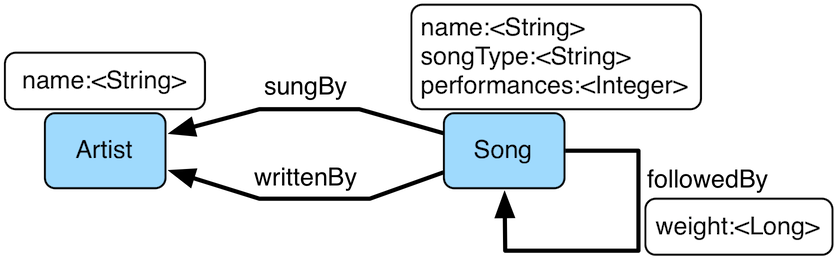`MatchStep` brings functionality similar to SPARQL to Gremlin. Like SPARQL, MatchStep conjoins a set of patterns applied to a graph. For example, the following traversal finds exactly those songs which Jerry Garcia has both sung and written (using the Grateful Dead graph distributed in the `data/` directory):

``````gremlin> g = graph.traversal()
==>graphtraversalsource[tinkergraph[vertices:0 edges:0], standard]
gremlin> g.V().match(
__.as('a').has('name', 'Garcia'),
__.as('a').in('writtenBy').as('b'),
__.as('a').in('sungBy').as('b')).
select('b').values('name')
==>CREAM PUFF WAR
==>CRYPTICAL ENVELOPMENT``````
``````g = graph.traversal()
g.V().match(
__.as('a').has('name', 'Garcia'),
__.as('a').in('writtenBy').as('b'),
__.as('a').in('sungBy').as('b')).
select('b').values('name')``````

Among the features which differentiate `match()` from SPARQL are:

``````gremlin> g.V().match(
__.as('a').out('created').has('name','lop').as('b'), //1\
__.as('b').in('created').has('age', 29).as('c'),
__.as('c').repeat(out()).times(2)). //2\
select('c').out('knows').dedup().values('name') //3\
==>josh``````
``````g.V().match(
__.as('a').out('created').has('name','lop').as('b'), //1\
__.as('b').in('created').has('age', 29).as('c'),
__.as('c').repeat(out()).times(2)). //2\
select('c').out('knows').dedup().values('name') //3``````
1. Patterns of arbitrary complexity: `match()` is not restricted to triple patterns or property paths.

2. Recursion support: `match()` supports the branch-based steps within a pattern, including `repeat()`.

3. Imperative/declarative hybrid: Before and after a `match()`, it is possible to leverage classic Gremlin traversals.

To extend point #3, it is possible to support going from imperative, to declarative, to imperative, ad infinitum.

``````gremlin> g.V().match(
__.as('a').out('knows').as('b'),
__.as('b').out('created').has('name','lop')).
select('b').out('created').
match(
__.as('x').in('created').as('y'),
__.as('y').out('knows').as('z')).
select('z').values('name')
==>josh``````
``````g.V().match(
__.as('a').out('knows').as('b'),
__.as('b').out('created').has('name','lop')).
select('b').out('created').
match(
__.as('x').in('created').as('y'),
__.as('y').out('knows').as('z')).
select('z').values('name')``````
 Important The `match()`-step is stateless. The variable bindings of the traversal patterns are stored in the path history of the traverser. As such, the variables used over all `match()`-steps within a traversal are globally unique. A benefit of this is that subsequent `where()`, `select()`, `match()`, etc. steps can leverage the same variables in their analysis.

Like all other steps in Gremlin, `match()` is a function and thus, `match()` within `match()` is a natural consequence of Gremlin’s functional foundation (i.e. recursive matching).

``````gremlin> g.V().match(
__.as('a').out('knows').as('b'),
__.as('b').out('created').has('name','lop'),
__.as('b').match(
__.as('b').out('created').as('c'),
__.as('c').has('name','ripple')).
select('c').as('c')).
select('a','c').by('name')
==>[a:marko,c:ripple]``````
``````g.V().match(
__.as('a').out('knows').as('b'),
__.as('b').out('created').has('name','lop'),
__.as('b').match(
__.as('b').out('created').as('c'),
__.as('c').has('name','ripple')).
select('c').as('c')).
select('a','c').by('name')``````

If a step-labeled traversal proceeds the `match()`-step and the traverser entering the `match()` is destined to bind to a particular variable, then the previous step should be labeled accordingly.

``````gremlin> g.V().as('a').out('knows').as('b').
match(
__.as('b').out('created').as('c'),
__.not(__.as('c').in('created').as('a'))).
select('a','b','c').by('name')
==>[a:marko,b:josh,c:ripple]``````
``````g.V().as('a').out('knows').as('b').
match(
__.as('b').out('created').as('c'),
__.not(__.as('c').in('created').as('a'))).
select('a','b','c').by('name')``````

There are three types of `match()` traversal patterns.

1. `as('a')…​as('b')`: both the start and end of the traversal have a declared variable.

2. `as('a')…​`: only the start of the traversal has a declared variable.

3. `…​`: there are no declared variables.

If a variable is at the start of a traversal pattern it must exist as a label in the path history of the traverser else the traverser can not go down that path. If a variable is at the end of a traversal pattern then if the variable exists in the path history of the traverser, the traverser’s current location must match (i.e. equal) its historic location at that same label. However, if the variable does not exist in the path history of the traverser, then the current location is labeled as the variable and thus, becomes a bound variable for subsequent traversal patterns. If a traversal pattern does not have an end label, then the traverser must simply "survive" the pattern (i.e. not be filtered) to continue to the next pattern. If a traversal pattern does not have a start label, then the traverser can go down that path at any point, but will only go down that pattern once as a traversal pattern is executed once and only once for the history of the traverser. Typically, traversal patterns that do not have a start and end label are used in conjunction with `and()`, `or()`, and `where()`. Once the traverser has "survived" all the patterns (or at least one for `or()`), `match()`-step analyzes the traverser’s path history and emits a `Map<String,Object>` of the variable bindings to the next step in the traversal.

``````gremlin> g.V().as('a').out().as('b'). //1\
match( //2\
__.as('a').out().count().as('c'), //3\
__.not(__.as('a').in().as('b')), //4\
or( //5\
__.as('a').out('knows').as('b'),
__.as('b').in().count().as('c').and().as('c').is(gt(2)))). //6\
dedup('a','c'). //7\
select('a','b','c').by('name').by('name').by() //8\
==>[a:marko,b:lop,c:3]``````
``````g.V().as('a').out().as('b'). //1\
match( //2\
__.as('a').out().count().as('c'), //3\
__.not(__.as('a').in().as('b')), //4\
or( //5\
__.as('a').out('knows').as('b'),
__.as('b').in().count().as('c').and().as('c').is(gt(2)))). //6\
dedup('a','c'). //7\
select('a','b','c').by('name').by('name').by() //8``````
1. A standard, step-labeled traversal can come prior to `match()`.

2. If the traverser’s path prior to entering `match()` has requisite label values, then those historic values are bound.

3. It is possible to use barrier steps though they are computed locally to the pattern (as one would expect).

4. It is possible to `not()` a pattern.

5. It is possible to nest `and()`- and `or()`-steps for conjunction matching.

6. Both infix and prefix conjunction notation is supported.

7. It is possible to "distinct" the specified label combination.

8. The bound values are of different types — vertex ("a"), vertex ("b"), long ("c").

#### Using Where with Match

Match is typically used in conjunction with both `select()` (demonstrated previously) and `where()` (presented here). A `where()`-step allows the user to further constrain the result set provided by `match()`.

``````gremlin> g.V().match(
__.as('a').out('created').as('b'),
__.as('b').in('created').as('c')).
where('a', neq('c')).
select('a','c').by('name')
==>[a:marko,c:josh]
==>[a:marko,c:peter]
==>[a:josh,c:marko]
==>[a:josh,c:peter]
==>[a:peter,c:marko]
==>[a:peter,c:josh]``````
``````g.V().match(
__.as('a').out('created').as('b'),
__.as('b').in('created').as('c')).
where('a', neq('c')).
select('a','c').by('name')``````

The `where()`-step can take either a `P`-predicate (example above) or a `Traversal` (example below). Using `MatchPredicateStrategy`, `where()`-clauses are automatically folded into `match()` and thus, subject to the query optimizer within `match()`-step.

``````gremlin> traversal = g.V().match(
__.as('a').has(label,'person'), //1\
__.as('a').out('created').as('b'),
__.as('b').in('created').as('c')).
where(__.as('a').out('knows').as('c')). //2\
select('a','c').by('name'); null //3\
gremlin> traversal.toString() //4\
==>[GraphStep(vertex,[]), MatchStep(AND,[[MatchStartStep(a), HasStep([~label.eq(person)]), MatchEndStep], [MatchStartStep(a), VertexStep(OUT,[created],vertex), MatchEndStep(b)], [MatchStartStep(b), VertexStep(IN,[created],vertex), MatchEndStep(c)]]), WhereTraversalStep([WhereStartStep(a), VertexStep(OUT,[knows],vertex), WhereEndStep(c)]), SelectStep(last,[a, c],[value(name)])]
gremlin> traversal // (5) (6)
==>[a:marko,c:josh]
gremlin> traversal.toString() //7\
==>[TinkerGraphStep(vertex,[~label.eq(person)])@[a], MatchStep(AND,[[MatchStartStep(a), VertexStep(OUT,[created],vertex), MatchEndStep(b)], [MatchStartStep(b), VertexStep(IN,[created],vertex), MatchEndStep(c)], [MatchStartStep(a), WhereTraversalStep([WhereStartStep, VertexStep(OUT,[knows],vertex), WhereEndStep(c)]), MatchEndStep]]), SelectStep(last,[a, c],[value(name)])]``````
``````traversal = g.V().match(
__.as('a').has(label,'person'), //1\
__.as('a').out('created').as('b'),
__.as('b').in('created').as('c')).
where(__.as('a').out('knows').as('c')). //2\
select('a','c').by('name'); null //3\
traversal.toString() //4\
traversal // (5) (6) (5)
traversal.toString() //7``````
1. Any `has()`-step traversal patterns that start with the match-key are pulled out of `match()` to enable the graph system to leverage the filter for index lookups.

2. A `where()`-step with a traversal containing variable bindings declared in `match()`.

3. A useful trick to ensure that the traversal is not iterated by Gremlin Console.

4. The string representation of the traversal prior to its strategies being applied.

5. The Gremlin Console will automatically iterate anything that is an iterator or is iterable.

6. Both marko and josh are co-developers and marko knows josh.

7. The string representation of the traversal after the strategies have been applied (and thus, `where()` is folded into `match()`)

 Important A `where()`-step is a filter and thus, variables within a `where()` clause are not globally bound to the path of the traverser in `match()`. As such, `where()`-steps in `match()` are used for filtering, not binding.

### Math Step

The `math()`-step (math) enables scientific calculator functionality within Gremlin. This step deviates from the common function composition and nesting formalisms to provide an easy to read string-based math processor. Variables within the equation map to scopes in Gremlin — e.g. path labels, side-effects, or incoming map keys. This step supports `by()`-modulation where the `by()`-modulators are applied in the order in which the variables are first referenced within the equation. Note that the reserved variable `_` refers to the current numeric traverser object incoming to the `math()`-step.

``````gremlin> g.V().as('a').out('knows').as('b').math('a + b').by('age')
==>56.0
==>61.0
gremlin> g.V().as('a').out('created').as('b').
math('b + a').
by(both().count().math('_ + 100')).
by('age')
==>132.0
==>133.0
==>135.0
==>138.0
gremlin> g.withSideEffect('x',10).V().values('age').math('_ / x')
==>2.9
==>2.7
==>3.2
==>3.5
gremlin> g.withSack(1).V(1).repeat(sack(sum).by(constant(1))).times(10).emit().sack().math('sin _')
==>0.9092974268256817
==>0.1411200080598672
==>-0.7568024953079282
==>-0.9589242746631385
==>-0.27941549819892586
==>0.6569865987187891
==>0.9893582466233818
==>0.4121184852417566
==>-0.5440211108893698
==>-0.9999902065507035``````
``````g.V().as('a').out('knows').as('b').math('a + b').by('age')
g.V().as('a').out('created').as('b').
math('b + a').
by(both().count().math('_ + 100')).
by('age')
g.withSideEffect('x',10).V().values('age').math('_ / x')
g.withSack(1).V(1).repeat(sack(sum).by(constant(1))).times(10).emit().sack().math('sin _')``````

The operators supported by the calculator include: `*`, `+`, `\`, `^`, and `%`. Furthermore, the following built in functions are provided:

• `abs`: absolute value

• `acos`: arc cosine

• `asin`: arc sine

• `atan`: arc tangent

• `cbrt`: cubic root

• `ceil`: nearest upper integer

• `cos`: cosine

• `cosh`: hyperbolic cosine

• `exp`: euler’s number raised to the power (`e^x`)

• `floor`: nearest lower integer

• `log`: logarithmus naturalis (base e)

• `log10`: logarithm (base 10)

• `log2`: logarithm (base 2)

• `sin`: sine

• `sinh`: hyperbolic sine

• `sqrt`: square root

• `tan`: tangent

• `tanh`: hyperbolic tangent

• `signum`: signum function

### Max Step

The `max()`-step (map) operates on a stream of comparable objects and determines which is the last object according to its natural order in the stream.

``````gremlin> g.V().values('age').max()
==>35
gremlin> g.V().repeat(both()).times(3).values('age').max()
==>35
gremlin> g.V().values('name').max()
``````g.V().values('age').max()
g.V().repeat(both()).times(3).values('age').max()
g.V().values('name').max()``````
 Important `max(local)` determines the max of the current, local object (not the objects in the traversal stream). This works for `Collection` and `Comparable`-type objects.

### Mean Step

The `mean()`-step (map) operates on a stream of numbers and determines the average of those numbers.

``````gremlin> g.V().values('age').mean()
==>30.75
gremlin> g.V().repeat(both()).times(3).values('age').mean() //1\
==>30.645833333333332
gremlin> g.V().repeat(both()).times(3).values('age').dedup().mean()
==>30.75``````
``````g.V().values('age').mean()
g.V().repeat(both()).times(3).values('age').mean() //1\
g.V().repeat(both()).times(3).values('age').dedup().mean()``````
1. Realize that traversers are being bulked by `repeat()`. There may be more of a particular number than another, thus altering the average.

 Important `mean(local)` determines the mean of the current, local object (not the objects in the traversal stream). This works for `Collection` and `Number`-type objects.

### Min Step

The `min()`-step (map) operates on a stream of comparable objects and determines which is the first object according to its natural order in the stream.

``````gremlin> g.V().values('age').min()
==>27
gremlin> g.V().repeat(both()).times(3).values('age').min()
==>27
gremlin> g.V().values('name').min()
==>josh``````
``````g.V().values('age').min()
g.V().repeat(both()).times(3).values('age').min()
g.V().values('name').min()``````
 Important `min(local)` determines the min of the current, local object (not the objects in the traversal stream). This works for `Collection` and `Comparable`-type objects.

### None Step

The `none()`-step (filter) filters all objects from a traversal stream. It is especially useful for to traversals that are executed remotely where returning results is not useful and the traversal is only meant to generate side-effects. Choosing not to return results saves in serialization and network costs as the objects are filtered on the remote end and not returned to the client side. Typically, this step does not need to be used directly and is quietly used by the `iterate()` terminal step which appends `none()` to the traversal before actually cycling through results.

### Not Step

The `not()`-step (filter) removes objects from the traversal stream when the traversal provided as an argument returns an object.

 Groovy The term `not` is a reserved word in Groovy, and when therefore used as part of an anonymous traversal must be referred to in Gremlin with the double underscore `__.not()`.
 Python The term `not` is a reserved word in Python, and therefore must be referred to in Gremlin with `not_()`.
``````gremlin> g.V().not(hasLabel('person')).valueMap().with(WithOptions.tokens)
==>[id:3,label:software,name:[lop],lang:[java]]
==>[id:5,label:software,name:[ripple],lang:[java]]
gremlin> g.V().hasLabel('person').
not(out('created').count().is(gt(1))).values('name') //1\
==>marko
==>peter``````
``````g.V().not(hasLabel('person')).valueMap().with(WithOptions.tokens)
g.V().hasLabel('person').
not(out('created').count().is(gt(1))).values('name')   //1``````
1. josh created two projects and vadas none

### Optional Step

The `optional()`-step (branch/flatMap) returns the result of the specified traversal if it yields a result else it returns the calling element, i.e. the `identity()`.

``````gremlin> g.V(2).optional(out('knows')) //1\
==>v
gremlin> g.V(2).optional(__.in('knows')) //2\
==>v``````
``````g.V(2).optional(out('knows')) //1\
g.V(2).optional(__.in('knows')) //2``````
1. vadas does not have an outgoing knows-edge so vadas is returned.

2. vadas does have an incoming knows-edge so marko is returned.

`optional` is particularly useful for lifting entire graphs when used in conjunction with `path` or `tree`.

``````gremlin> g.V().hasLabel('person').optional(out('knows').optional(out('created'))).path() //1\
==>[v,v]
==>[v,v,v]
==>[v,v,v]
==>[v]
==>[v]
==>[v]``````
``g.V().hasLabel('person').optional(out('knows').optional(out('created'))).path() //1``
1. Returns the paths of everybody followed by who they know followed by what they created.

### Or Step

The `or()`-step ensures that at least one of the provided traversals yield a result (filter). Please see `and()` for and-semantics.

 Python The term `or` is a reserved word in Python, and therefore must be referred to in Gremlin with `or_()`.
``````gremlin> g.V().or(
__.outE('created'),
__.inE('created').count().is(gt(1))).
values('name')
==>marko
==>lop
==>josh
==>peter``````
``````g.V().or(
__.outE('created'),
__.inE('created').count().is(gt(1))).
values('name')``````

The `or()`-step can take an arbitrary number of traversals. At least one of the traversals must produce at least one output for the original traverser to pass to the next step.

An infix notation can be used as well.

``````gremlin> g.V().where(outE('created').or().outE('knows')).values('name')
==>marko
==>josh
==>peter``````
``g.V().where(outE('created').or().outE('knows')).values('name')``

### Order Step

When the objects of the traversal stream need to be sorted, `order()`-step (map) can be leveraged.

``````gremlin> g.V().values('name').order()
==>josh
==>lop
==>marko
==>peter
==>ripple
gremlin> g.V().values('name').order().by(desc)
==>ripple
==>peter
==>marko
==>lop
==>josh
gremlin> g.V().hasLabel('person').order().by('age', asc).values('name')
==>marko
==>josh
==>peter``````
``````g.V().values('name').order()
g.V().values('name').order().by(desc)
g.V().hasLabel('person').order().by('age', asc).values('name')``````

One of the most traversed objects in a traversal is an `Element`. An element can have properties associated with it (i.e. key/value pairs). In many situations, it is desirable to sort an element traversal stream according to a comparison of their properties.

``````gremlin> g.V().values('name')
==>marko
==>lop
==>josh
==>ripple
==>peter
gremlin> g.V().order().by('name',asc).values('name')
==>josh
==>lop
==>marko
==>peter
==>ripple
gremlin> g.V().order().by('name',desc).values('name')
==>ripple
==>peter
==>marko
==>lop
==>josh``````
``````g.V().values('name')
g.V().order().by('name',asc).values('name')
g.V().order().by('name',desc).values('name')``````

The `order()`-step allows the user to provide an arbitrary number of comparators for primary, secondary, etc. sorting. In the example below, the primary ordering is based on the outgoing created-edge count. The secondary ordering is based on the age of the person.

``````gremlin> g.V().hasLabel('person').order().by(outE('created').count(), asc).
by('age', asc).values('name')
==>marko
==>peter
==>josh
gremlin> g.V().hasLabel('person').order().by(outE('created').count(), asc).
by('age', desc).values('name')
==>peter
==>marko
==>josh``````
``````g.V().hasLabel('person').order().by(outE('created').count(), asc).
by('age', asc).values('name')
g.V().hasLabel('person').order().by(outE('created').count(), asc).
by('age', desc).values('name')``````

Randomizing the order of the traversers at a particular point in the traversal is possible with `Order.shuffle`.

``````gremlin> g.V().hasLabel('person').order().by(shuffle)
==>v
==>v
==>v
==>v
gremlin> g.V().hasLabel('person').order().by(shuffle)
==>v
==>v
==>v
==>v``````
``````g.V().hasLabel('person').order().by(shuffle)
g.V().hasLabel('person').order().by(shuffle)``````

It is possible to use `order(local)` to order the current local object and not the entire traversal stream. This works for `Collection`- and `Map`-type objects. For any other object, the object is returned unchanged.

``````gremlin> g.V().values('age').fold().order(local).by(desc) //1\
==>[35,32,29,27]
gremlin> g.V().values('age').order(local).by(desc) //2\
==>29
==>27
==>32
==>35
gremlin> g.V().groupCount().by(inE().count()).order(local).by(values, desc) //3\
==>[1:3,0:2,3:1]
gremlin> g.V().groupCount().by(inE().count()).order(local).by(keys, asc) //4\
==>[0:2,1:3,3:1]``````
``````g.V().values('age').fold().order(local).by(desc) //1\
g.V().values('age').order(local).by(desc) //2\
g.V().groupCount().by(inE().count()).order(local).by(values, desc) //3\
g.V().groupCount().by(inE().count()).order(local).by(keys, asc) //4``````
1. The ages are gathered into a list and then that list is sorted in decreasing order.

2. The ages are not gathered and thus `order(local)` is "ordering" single integers and thus, does nothing.

3. The `groupCount()` map is ordered by its values in decreasing order.

4. The `groupCount()` map is ordered by its keys in increasing order.

 Note The `values` and `keys` enums are from `Column` which is used to select "columns" from a `Map`, `Map.Entry`, or `Path`.
 Note Prior to version 3.3.4, ordering was defined by `Order.incr` for ascending order and `Order.decr` for descending order. That approach is now deprecated with the preferred method shown in the examples which uses the more common forms for query languages in `Order.asc` and Order.desc.

### PageRank Step

The `pageRank()`-step (map/sideEffect) calculates PageRank using `PageRankVertexProgram`.

 Important The `pageRank()`-step is a `VertexComputing`-step and as such, can only be used against a graph that supports `GraphComputer` (OLAP).
``````gremlin> g = graph.traversal().withComputer()
==>graphtraversalsource[tinkergraph[vertices:6 edges:6], graphcomputer]
gremlin> g.V().pageRank().by('pageRank').values('pageRank')
==>0.11375510357865536
==>0.11375510357865536
==>0.145985401527191
==>0.145985401527191
==>0.3047200907912248
==>0.17579889899708226
gremlin> g.V().hasLabel('person').
pageRank().
with(PageRank.edges, __.outE('knows')).
with(PageRank.propertyName, 'friendRank').
order().by('friendRank',desc).valueMap('name','friendRank')
==>[friendRank:[0.8321166533236799],name:[josh]]
==>[friendRank:[0.5839416733381598],name:[marko]]
==>[friendRank:[0.5839416733381598],name:[peter]]``````
``````g = graph.traversal().withComputer()
g.V().pageRank().by('pageRank').values('pageRank')
g.V().hasLabel('person').
pageRank().
with(PageRank.edges, __.outE('knows')).
with(PageRank.propertyName, 'friendRank').
order().by('friendRank',desc).valueMap('name','friendRank')``````

Note the use of the `with()` modulating step which provides configuration options to the algorithm. It takes configuration keys from the `PageRank` and is automatically imported to the Gremlin Console.

The `explain()`-step can be used to understand how the traversal is compiled into multiple `GraphComputer` jobs.

``````gremlin> g = graph.traversal().withComputer()
==>graphtraversalsource[tinkergraph[vertices:6 edges:6], graphcomputer]
gremlin> g.V().hasLabel('person').
pageRank().
with(PageRank.edges, __.outE('knows')).
with(PageRank.propertyName, 'friendRank').
order().by('friendRank',desc).valueMap('name','friendRank').explain()
==>Traversal Explanation
=============================================================================================================================================================================================================================================
Original Traversal                    [GraphStep(vertex,[]), HasStep([~label.eq(person)]), PageRankVertexProgramStep([VertexStep(OUT,[knows],edge)],friendRank,20,graphfilter[none]), OrderGlobalStep([[value(friendRank), desc]]), PropertyM
apStep([name, friendRank],value)]

ConnectiveStrategy              [D]   [GraphStep(vertex,[]), HasStep([~label.eq(person)]), PageRankVertexProgramStep([VertexStep(OUT,[knows],edge)],friendRank,20,graphfilter[none]), OrderGlobalStep([[value(friendRank), desc]]), PropertyM
apStep([name, friendRank],value)]
VertexProgramStrategy           [D]   [TraversalVertexProgramStep([GraphStep(vertex,[]), HasStep([~label.eq(person)])],graphfilter[none]), PageRankVertexProgramStep([VertexStep(OUT,[knows],edge)],friendRank,20,graphfilter[none]), Travers
alVertexProgramStep([OrderGlobalStep([[value(friendRank), desc]]), PropertyMapStep([name, friendRank],value)],graphfilter[none]), ComputerResultStep]
EarlyLimitStrategy              [O]   [TraversalVertexProgramStep([GraphStep(vertex,[]), HasStep([~label.eq(person)])],graphfilter[none]), PageRankVertexProgramStep([VertexStep(OUT,[knows],edge)],friendRank,20,graphfilter[none]), Travers
alVertexProgramStep([OrderGlobalStep([[value(friendRank), desc]]), PropertyMapStep([name, friendRank],value)],graphfilter[none]), ComputerResultStep]
MatchPredicateStrategy          [O]   [TraversalVertexProgramStep([GraphStep(vertex,[]), HasStep([~label.eq(person)])],graphfilter[none]), PageRankVertexProgramStep([VertexStep(OUT,[knows],edge)],friendRank,20,graphfilter[none]), Travers
alVertexProgramStep([OrderGlobalStep([[value(friendRank), desc]]), PropertyMapStep([name, friendRank],value)],graphfilter[none]), ComputerResultStep]
FilterRankingStrategy           [O]   [TraversalVertexProgramStep([GraphStep(vertex,[]), HasStep([~label.eq(person)])],graphfilter[none]), PageRankVertexProgramStep([VertexStep(OUT,[knows],edge)],friendRank,20,graphfilter[none]), Travers
alVertexProgramStep([OrderGlobalStep([[value(friendRank), desc]]), PropertyMapStep([name, friendRank],value)],graphfilter[none]), ComputerResultStep]
PathProcessorStrategy           [O]   [TraversalVertexProgramStep([GraphStep(vertex,[]), HasStep([~label.eq(person)])],graphfilter[none]), PageRankVertexProgramStep([VertexStep(OUT,[knows],edge)],friendRank,20,graphfilter[none]), Travers
alVertexProgramStep([OrderGlobalStep([[value(friendRank), desc]]), PropertyMapStep([name, friendRank],value)],graphfilter[none]), ComputerResultStep]
InlineFilterStrategy            [O]   [TraversalVertexProgramStep([GraphStep(vertex,[]), HasStep([~label.eq(person)])],graphfilter[none]), PageRankVertexProgramStep([VertexStep(OUT,[knows],edge)],friendRank,20,graphfilter[none]), Travers
alVertexProgramStep([OrderGlobalStep([[value(friendRank), desc]]), PropertyMapStep([name, friendRank],value)],graphfilter[none]), ComputerResultStep]
IncidentToAdjacentStrategy      [O]   [TraversalVertexProgramStep([GraphStep(vertex,[]), HasStep([~label.eq(person)])],graphfilter[none]), PageRankVertexProgramStep([VertexStep(OUT,[knows],edge)],friendRank,20,graphfilter[none]), Travers
alVertexProgramStep([OrderGlobalStep([[value(friendRank), desc]]), PropertyMapStep([name, friendRank],value)],graphfilter[none]), ComputerResultStep]
AdjacentToIncidentStrategy      [O]   [TraversalVertexProgramStep([GraphStep(vertex,[]), HasStep([~label.eq(person)])],graphfilter[none]), PageRankVertexProgramStep([VertexStep(OUT,[knows],edge)],friendRank,20,graphfilter[none]), Travers
alVertexProgramStep([OrderGlobalStep([[value(friendRank), desc]]), PropertyMapStep([name, friendRank],value)],graphfilter[none]), ComputerResultStep]
RepeatUnrollStrategy            [O]   [TraversalVertexProgramStep([GraphStep(vertex,[]), HasStep([~label.eq(person)])],graphfilter[none]), PageRankVertexProgramStep([VertexStep(OUT,[knows],edge)],friendRank,20,graphfilter[none]), Travers
alVertexProgramStep([OrderGlobalStep([[value(friendRank), desc]]), PropertyMapStep([name, friendRank],value)],graphfilter[none]), ComputerResultStep]
CountStrategy                   [O]   [TraversalVertexProgramStep([GraphStep(vertex,[]), HasStep([~label.eq(person)])],graphfilter[none]), PageRankVertexProgramStep([VertexStep(OUT,[knows],edge)],friendRank,20,graphfilter[none]), Travers
alVertexProgramStep([OrderGlobalStep([[value(friendRank), desc]]), PropertyMapStep([name, friendRank],value)],graphfilter[none]), ComputerResultStep]
PathRetractionStrategy          [O]   [TraversalVertexProgramStep([GraphStep(vertex,[]), HasStep([~label.eq(person)])],graphfilter[none]), PageRankVertexProgramStep([VertexStep(OUT,[knows],edge)],friendRank,20,graphfilter[none]), Travers
alVertexProgramStep([OrderGlobalStep([[value(friendRank), desc]]), PropertyMapStep([name, friendRank],value)],graphfilter[none]), ComputerResultStep]
LazyBarrierStrategy             [O]   [TraversalVertexProgramStep([GraphStep(vertex,[]), HasStep([~label.eq(person)])],graphfilter[none]), PageRankVertexProgramStep([VertexStep(OUT,[knows],edge)],friendRank,20,graphfilter[none]), Travers
alVertexProgramStep([OrderGlobalStep([[value(friendRank), desc]]), PropertyMapStep([name, friendRank],value)],graphfilter[none]), ComputerResultStep]
OrderLimitStrategy              [O]   [TraversalVertexProgramStep([GraphStep(vertex,[]), HasStep([~label.eq(person)])],graphfilter[none]), PageRankVertexProgramStep([VertexStep(OUT,[knows],edge)],friendRank,20,graphfilter[none]), Travers
alVertexProgramStep([OrderGlobalStep([[value(friendRank), desc]]), PropertyMapStep([name, friendRank],value)],graphfilter[none]), ComputerResultStep]
MessagePassingReductionStrategy [O]   [TraversalVertexProgramStep([GraphStep(vertex,[]), HasStep([~label.eq(person)])],graphfilter[none]), PageRankVertexProgramStep([VertexStep(OUT,[knows],edge)],friendRank,20,graphfilter[none]), Travers
alVertexProgramStep([OrderGlobalStep([[value(friendRank), desc]]), PropertyMapStep([name, friendRank],value)],graphfilter[none]), ComputerResultStep]
TinkerGraphCountStrategy        [P]   [TraversalVertexProgramStep([GraphStep(vertex,[]), HasStep([~label.eq(person)])],graphfilter[none]), PageRankVertexProgramStep([VertexStep(OUT,[knows],edge)],friendRank,20,graphfilter[none]), Travers
alVertexProgramStep([OrderGlobalStep([[value(friendRank), desc]]), PropertyMapStep([name, friendRank],value)],graphfilter[none]), ComputerResultStep]
TinkerGraphStepStrategy         [P]   [TraversalVertexProgramStep([GraphStep(vertex,[]), HasStep([~label.eq(person)])],graphfilter[none]), PageRankVertexProgramStep([VertexStep(OUT,[knows],edge)],friendRank,20,graphfilter[none]), Travers
alVertexProgramStep([OrderGlobalStep([[value(friendRank), desc]]), PropertyMapStep([name, friendRank],value)],graphfilter[none]), ComputerResultStep]
ProfileStrategy                 [F]   [TraversalVertexProgramStep([GraphStep(vertex,[]), HasStep([~label.eq(person)])],graphfilter[none]), PageRankVertexProgramStep([VertexStep(OUT,[knows],edge)],friendRank,20,graphfilter[none]), Travers
alVertexProgramStep([OrderGlobalStep([[value(friendRank), desc]]), PropertyMapStep([name, friendRank],value)],graphfilter[none]), ComputerResultStep]
ComputerVerificationStrategy    [V]   [TraversalVertexProgramStep([GraphStep(vertex,[]), HasStep([~label.eq(person)])],graphfilter[none]), PageRankVertexProgramStep([VertexStep(OUT,[knows],edge)],friendRank,20,graphfilter[none]), Travers
alVertexProgramStep([OrderGlobalStep([[value(friendRank), desc]]), PropertyMapStep([name, friendRank],value)],graphfilter[none]), ComputerResultStep]
StandardVerificationStrategy    [V]   [TraversalVertexProgramStep([GraphStep(vertex,[]), HasStep([~label.eq(person)])],graphfilter[none]), PageRankVertexProgramStep([VertexStep(OUT,[knows],edge)],friendRank,20,graphfilter[none]), Travers
alVertexProgramStep([OrderGlobalStep([[value(friendRank), desc]]), PropertyMapStep([name, friendRank],value)],graphfilter[none]), ComputerResultStep]
ComputerFinalizationStrategy    [T]   [TraversalVertexProgramStep([GraphStep(vertex,[]), HasStep([~label.eq(person)])],graphfilter[none]), PageRankVertexProgramStep([VertexStep(OUT,[knows],edge)],friendRank,20,graphfilter[none]), Travers
alVertexProgramStep([OrderGlobalStep([[value(friendRank), desc]]), PropertyMapStep([name, friendRank],value)],graphfilter[none]), ComputerResultStep]

Final Traversal                       [TraversalVertexProgramStep([GraphStep(vertex,[]), HasStep([~label.eq(person)])],graphfilter[none]), PageRankVertexProgramStep([VertexStep(OUT,[knows],edge)],friendRank,20,graphfilter[none]), Travers
alVertexProgramStep([OrderGlobalStep([[value(friendRank), desc]]), PropertyMapStep([name, friendRank],value)],graphfilter[none]), ComputerResultStep]``````
``````g = graph.traversal().withComputer()
g.V().hasLabel('person').
pageRank().
with(PageRank.edges, __.outE('knows')).
with(PageRank.propertyName, 'friendRank').
order().by('friendRank',desc).valueMap('name','friendRank').explain()``````

### Path Step

A traverser is transformed as it moves through a series of steps within a traversal. The history of the traverser is realized by examining its path with `path()`-step (map).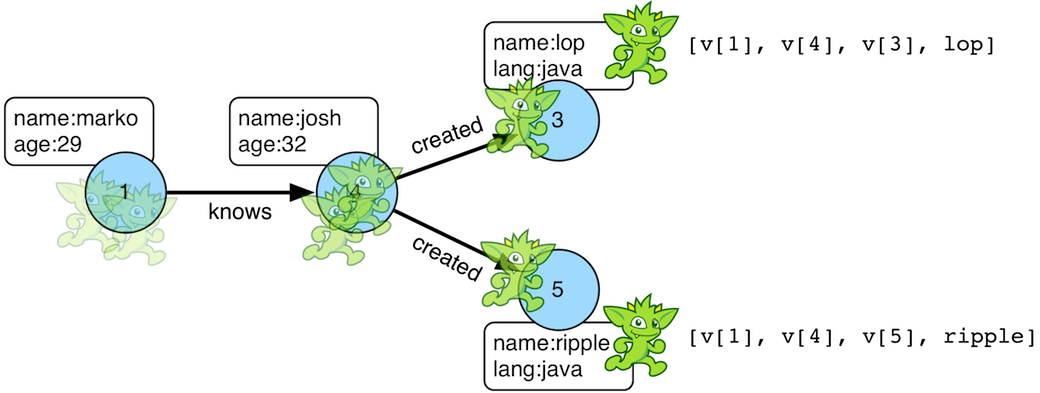``````gremlin> g.V().out().out().values('name')
==>ripple
==>lop
gremlin> g.V().out().out().values('name').path()
==>[v,v,v,ripple]
==>[v,v,v,lop]``````
``````g.V().out().out().values('name')
g.V().out().out().values('name').path()``````

If edges are required in the path, then be sure to traverser those edges explicitly.

``````gremlin> g.V().outE().inV().outE().inV().path()
==>[v,e[1-knows->4],v,e[4-created->5],v]
==>[v,e[1-knows->4],v,e[4-created->3],v]``````
``g.V().outE().inV().outE().inV().path()``

It is possible to post-process the elements of the path in a round-robin fashion via `by()`.

``````gremlin> g.V().out().out().path().by('name').by('age')
==>[marko,32,ripple]
==>[marko,32,lop]``````
``g.V().out().out().path().by('name').by('age')``

Finally, because `by()`-based post-processing, nothing prevents triggering yet another traversal. In the traversal below, for each element of the path traversed thus far, if its a person (as determined by having an `age`-property), then get all of their creations, else if its a creation, get all the people that created it.

``````gremlin> g.V().out().out().path().by(
choose(hasLabel('person'),
out('created').values('name'),
__.in('created').values('name')).fold())
==>[[lop],[ripple,lop],[josh]]
==>[[lop],[ripple,lop],[marko,josh,peter]]``````
``````g.V().out().out().path().by(
choose(hasLabel('person'),
out('created').values('name'),
__.in('created').values('name')).fold())``````
 Warning Generating path information is expensive as the history of the traverser is stored into a Java list. With numerous traversers, there are numerous lists. Moreover, in an OLAP `GraphComputer` environment this becomes exceedingly prohibitive as there are traversers emanating from all vertices in the graph in parallel. In OLAP there are optimizations provided for traverser populations, but when paths are calculated (and each traverser is unique due to its history), then these optimizations are no longer possible.

#### Path Data Structure

The `Path` data structure is an ordered list of objects, where each object is associated to a `Set<String>` of labels. An example is presented below to demonstrate both the `Path` API as well as how a traversal yields labeled paths.``````gremlin> path = g.V(1).as('a').has('name').as('b').
out('knows').out('created').as('c').
has('name','ripple').values('name').as('d').
identity().as('e').path().next()
==>v
==>v
==>v
==>ripple
gremlin> path.size()
==>4
gremlin> path.objects()
==>v
==>v
==>v
==>ripple
gremlin> path.labels()
==>[b,a]
==>[]
==>[c]
==>[d,e]
gremlin> path.a
==>v
gremlin> path.b
==>v
gremlin> path.c
==>v
gremlin> path.d == path.e
==>true``````
``````path = g.V(1).as('a').has('name').as('b').
out('knows').out('created').as('c').
has('name','ripple').values('name').as('d').
identity().as('e').path().next()
path.size()
path.objects()
path.labels()
path.a
path.b
path.c
path.d == path.e``````

### PeerPressure Step

The `peerPressure()`-step (map/sideEffect) clusters vertices using `PeerPressureVertexProgram`.

 Important The `peerPressure()`-step is a `VertexComputing`-step and as such, can only be used against a graph that supports `GraphComputer` (OLAP).
``````gremlin> g = graph.traversal().withComputer()
==>graphtraversalsource[tinkergraph[vertices:6 edges:6], graphcomputer]
gremlin> g.V().peerPressure().by('cluster').values('cluster')
==>1
==>1
==>1
==>1
==>1
==>6
gremlin> g.V().hasLabel('person').
peerPressure().
with(PeerPressure.propertyName, 'cluster').
group().
by('cluster').
by('name')
``````g = graph.traversal().withComputer()
g.V().peerPressure().by('cluster').values('cluster')
g.V().hasLabel('person').
peerPressure().
with(PeerPressure.propertyName, 'cluster').
group().
by('cluster').
by('name')``````

Note the use of the `with()` modulating step which provides configuration options to the algorithm. It takes configuration keys from the `PeerPressure` class and is automatically imported to the Gremlin Console.

### Profile Step

The `profile()`-step (sideEffect) exists to allow developers to profile their traversals to determine statistical information like step runtime, counts, etc.

 Warning Profiling a Traversal will impede the Traversal’s performance. This overhead is mostly excluded from the profile results, but durations are not exact. Thus, durations are best considered in relation to each other.
``````gremlin> g.V().out('created').repeat(both()).times(3).hasLabel('person').values('age').sum().profile()
==>Traversal Metrics
Step                                                               Count  Traversers       Time (ms)    % Dur
=============================================================================================================
TinkerGraphStep(vertex,[])                                             6           6           0.169    16.20
VertexStep(OUT,[created],vertex)                                       4           4           0.251    23.96
NoOpBarrierStep(2500)                                                  4           2           0.071     6.87
VertexStep(BOTH,vertex)                                               10           4           0.030     2.93
NoOpBarrierStep(2500)                                                 10           3           0.028     2.70
VertexStep(BOTH,vertex)                                               24           7           0.034     3.31
NoOpBarrierStep(2500)                                                 24           5           0.034     3.33
VertexStep(BOTH,vertex)                                               58          11           0.052     5.02
NoOpBarrierStep(2500)                                                 58           6           0.066     6.39
HasStep([~label.eq(person)])                                          48           4           0.037     3.59
PropertiesStep([age],value)                                           48           4           0.046     4.44
SumGlobalStep                                                          1           1           0.222    21.25
>TOTAL                     -           -           1.047        -``````
``g.V().out('created').repeat(both()).times(3).hasLabel('person').values('age').sum().profile()``

The `profile()`-step generates a `TraversalMetrics` sideEffect object that contains the following information:

• `Step`: A step within the traversal being profiled.

• `Count`: The number of represented traversers that passed through the step.

• `Traversers`: The number of traversers that passed through the step.

• `Time (ms)`: The total time the step was actively executing its behavior.

• `% Dur`: The percentage of total time spent in the step.It is important to understand the difference between `Count` and `Traversers`. Traversers can be merged and as such, when two traversers are "the same" they may be aggregated into a single traverser. That new traverser has a `Traverser.bulk()` that is the sum of the two merged traverser bulks. On the other hand, the `Count` represents the sum of all `Traverser.bulk()` results and thus, expresses the number of "represented" (not enumerated) traversers. `Traversers` will always be less than or equal to `Count`.

A side effect key can also be passed to the `profile()`-step for situations when it is important to iterate the normal results of the Traversal and retrieve the `TraversalMetrics` afterwards, as shown here:

``````gremlin> t = g.V().out('created').profile('metrics')
==>v
==>v
==>v
==>v
gremlin> t.iterate()
gremlin> metrics = t.getSideEffects().get('metrics')
==>Traversal Metrics
Step                                                               Count  Traversers       Time (ms)    % Dur
=============================================================================================================
TinkerGraphStep(vertex,[])                                             6           6           0.055    33.53
VertexStep(OUT,[created],vertex)                                       4           4           0.056    34.33
NoOpBarrierStep(2500)                                                  4           2           0.053    32.14
>TOTAL                     -           -           0.166        -``````
``````t = g.V().out('created').profile('metrics')
t.iterate()
metrics = t.getSideEffects().get('metrics')``````

For traversal compilation information, please see `explain()`-step.

### Project Step

The `project()`-step (map) projects the current object into a `Map<String,Object>` keyed by provided labels. It is similar to `select()`-step, save that instead of retrieving and modulating historic traverser state, it modulates the current state of the traverser.

``````gremlin> g.V().out('created').
project('a','b').
by('name').
by(__.in('created').count()).
order().by(select('b'),desc).
select('a')
==>lop
==>lop
==>lop
==>ripple
gremlin> g.V().has('name','marko').
project('out','in').
by(outE().count()).
by(inE().count())
==>[out:3,in:0]``````
``````g.V().out('created').
project('a','b').
by('name').
by(__.in('created').count()).
order().by(select('b'),desc).
select('a')
g.V().has('name','marko').
project('out','in').
by(outE().count()).
by(inE().count())``````

### Program Step

The `program()`-step (map/sideEffect) is the "lambda" step for `GraphComputer` jobs. The step takes a `VertexProgram` as an argument and will process the incoming graph accordingly. Thus, the user can create their own `VertexProgram` and have it execute within a traversal. The configuration provided to the vertex program includes:

• `gremlin.vertexProgramStep.rootTraversal` is a serialization of a `PureTraversal` form of the root traversal.

• `gremlin.vertexProgramStep.stepId` is the step string id of the `program()`-step being executed.

The user supplied `VertexProgram` can leverage that information accordingly within their vertex program. Example uses are provided below.

 Warning Developing a `VertexProgram` is for expert users. Moreover, developing one that can be used effectively within a traversal requires yet more expertise. This information is recommended to advanced users with a deep understanding of the mechanics of Gremlin OLAP (`GraphComputer`).
``````private TraverserSet<Object> haltedTraversers;

public void loadState(Graph graph, Configuration configuration) {
this.programStep = new TraversalMatrix<>(this.traversal.get()).getStepById(configuration.getString(ProgramVertexProgramStep.STEP_ID));
// if the traversal sideEffects will be used in the computation, add them as memory compute keys
// if master-traversal traversers may be propagated, create a memory compute key
// returns an empty traverser set if there are no halted traversers
}

public void storeState(Configuration configuration) {
VertexProgram.super.storeState(configuration);
// if halted traversers is null or empty, it does nothing
TraversalVertexProgram.storeHaltedTraversers(configuration, this.haltedTraversers);
}

public void setup(Memory memory) {
if(!this.haltedTraversers.isEmpty()) {
// do what you like with the halted master traversal traversers
}
// once used, no need to keep that information around (master)
this.haltedTraversers = null;
}

public void execute(Vertex vertex, Messenger messenger, Memory memory) {
// once used, no need to keep that information around (workers)
if(null != this.haltedTraversers)
this.haltedTraversers = null;
if(vertex.property(TraversalVertexProgram.HALTED_TRAVERSERS).isPresent()) {
// haltedTraversers in execute() represent worker-traversal traversers
// for example, from a traversal of the form g.V().out().program(...)
TraverserSet<Object> haltedTraversers = vertex.value(TraversalVertexProgram.HALTED_TRAVERSERS);
// create a new halted traverser set that can be used by the next OLAP job in the chain
// these are worker-traversers that are distributed throughout the graph
TraverserSet<Object> newHaltedTraversers = new TraverserSet<>();
haltedTraversers.forEach(traverser -> {
});
vertex.property(VertexProperty.Cardinality.single, TraversalVertexProgram.HALTED_TRAVERSERS, newHaltedTraversers);
// it is possible to create master-traversers that are localized to the master traversal (this is how results are ultimately delivered back to the user)
new TraverserSet<>(this.traversal().get().getTraverserGenerator().generate("an example", this.programStep, 1l)));
}

public boolean terminate(Memory memory) {
// the master-traversal will have halted traversers
assert memory.exists(TraversalVertexProgram.HALTED_TRAVERSERS);
TraverserSet<String> haltedTraversers = memory.get(TraversalVertexProgram.HALTED_TRAVERSERS);
// it will only have the traversers sent to the master traversal via memory
assert haltedTraversers.stream().map(Traverser::get).filter(s -> s.equals("an example")).findAny().isPresent();
// it will not contain the worker traversers distributed throughout the vertices
assert !haltedTraversers.stream().map(Traverser::get).filter(s -> !s.equals("an example")).findAny().isPresent();
return true;
}``````
 Note The test case `ProgramTest` in `gremlin-test` has an example vertex program called `TestProgram` that demonstrates all the various ways in which traversal and traverser information is propagated within a vertex program and ultimately usable by other vertex programs (including `TraversalVertexProgram`) down the line in an OLAP compute chain.

Finally, an example is provided using `PageRankVertexProgram` which doesn’t use `pageRank()`-step.

``````gremlin> g = graph.traversal().withComputer()
==>graphtraversalsource[tinkergraph[vertices:6 edges:6], graphcomputer]
gremlin> g.V().hasLabel('person').
program(PageRankVertexProgram.build().property('rank').create(graph)).
order().by('rank', asc).
valueMap('name', 'rank')
==>[name:[marko],rank:[0.11375510357865537]]
==>[name:[peter],rank:[0.11375510357865537]]
==>[name:[josh],rank:[0.14598540152719103]]``````
``````g = graph.traversal().withComputer()
g.V().hasLabel('person').
program(PageRankVertexProgram.build().property('rank').create(graph)).
order().by('rank', asc).
valueMap('name', 'rank')``````

### Properties Step

The `properties()`-step (map) extracts properties from an `Element` in the traversal stream.

``````gremlin> g.V(1).properties()
==>vp[name->marko]
==>vp[location->san diego]
==>vp[location->santa cruz]
==>vp[location->brussels]
==>vp[location->santa fe]
gremlin> g.V(1).properties('location').valueMap()
==>[startTime:1997,endTime:2001]
==>[startTime:2001,endTime:2004]
==>[startTime:2004,endTime:2005]
==>[startTime:2005]
gremlin> g.V(1).properties('location').has('endTime').valueMap()
==>[startTime:1997,endTime:2001]
==>[startTime:2001,endTime:2004]
==>[startTime:2004,endTime:2005]``````
``````g.V(1).properties()
g.V(1).properties('location').valueMap()
g.V(1).properties('location').has('endTime').valueMap()``````

### PropertyMap Step

The `propertiesMap()`-step yields a Map representation of the properties of an element.

``````gremlin> g.V().propertyMap()
==>[name:[vp[name->marko]],age:[vp[age->29]]]
==>[name:[vp[name->lop]],lang:[vp[lang->java]]]
==>[name:[vp[name->josh]],age:[vp[age->32]]]
==>[name:[vp[name->ripple]],lang:[vp[lang->java]]]
==>[name:[vp[name->peter]],age:[vp[age->35]]]
gremlin> g.V().propertyMap('age')
==>[age:[vp[age->29]]]
==>[age:[vp[age->27]]]
==>[]
==>[age:[vp[age->32]]]
==>[]
==>[age:[vp[age->35]]]
gremlin> g.V().propertyMap('age','blah')
==>[age:[vp[age->29]]]
==>[age:[vp[age->27]]]
==>[]
==>[age:[vp[age->32]]]
==>[]
==>[age:[vp[age->35]]]
gremlin> g.E().propertyMap()
==>[weight:p[weight->0.5]]
==>[weight:p[weight->1.0]]
==>[weight:p[weight->0.4]]
==>[weight:p[weight->1.0]]
==>[weight:p[weight->0.4]]
==>[weight:p[weight->0.2]]``````
``````g.V().propertyMap()
g.V().propertyMap('age')
g.V().propertyMap('age','blah')
g.E().propertyMap()``````

### Range Step

As traversers propagate through the traversal, it is possible to only allow a certain number of them to pass through with `range()`-step (filter). When the low-end of the range is not met, objects are continued to be iterated. When within the low (inclusive) and high (exclusive) range, traversers are emitted. When above the high range, the traversal breaks out of iteration. Finally, the use of `-1` on the high range will emit remaining traversers after the low range begins.

``````gremlin> g.V().range(0,3)
==>v
==>v
==>v
gremlin> g.V().range(1,3)
==>v
==>v
gremlin> g.V().range(1, -1)
==>v
==>v
==>v
==>v
==>v
gremlin> g.V().repeat(both()).times(1000000).emit().range(6,10)
==>v
==>v
==>v
==>v``````
``````g.V().range(0,3)
g.V().range(1,3)
g.V().range(1, -1)
g.V().repeat(both()).times(1000000).emit().range(6,10)``````

The `range()`-step can also be applied with `Scope.local`, in which case it operates on the incoming collection. For example, it is possible to produce a `Map<String, String>` for each traversed path, but containing only the second property value (the "b" step).

``````gremlin> g.V().as('a').out().as('b').in().as('c').select('a','b','c').by('name').range(local,1,2)
==>[b:lop]
==>[b:lop]
==>[b:lop]
==>[b:josh]
==>[b:ripple]
==>[b:lop]
==>[b:lop]
==>[b:lop]
==>[b:lop]
==>[b:lop]
==>[b:lop]``````
``g.V().as('a').out().as('b').in().as('c').select('a','b','c').by('name').range(local,1,2)``

The next example uses the The Crew toy data set. It produces a `List<String>` containing the second and third location for each vertex.

``````gremlin> g.V().valueMap().select('location').range(local, 1, 3)
==>[santa cruz,brussels]
==>[dulles,purcellville]
==>[baltimore,oakland]
==>[kaiserslautern,aachen]``````
``g.V().valueMap().select('location').range(local, 1, 3)``

### Repeat StepThe `repeat()`-step (branch) is used for looping over a traversal given some break predicate. Below are some examples of `repeat()`-step in action.

``````gremlin> g.V(1).repeat(out()).times(2).path().by('name') //1\
==>[marko,josh,ripple]
==>[marko,josh,lop]
gremlin> g.V().until(has('name','ripple')).
repeat(out()).path().by('name') //2\
==>[marko,josh,ripple]
==>[josh,ripple]
==>[ripple]``````
``````g.V(1).repeat(out()).times(2).path().by('name') //1\
g.V().until(has('name','ripple')).
repeat(out()).path().by('name') //2``````
1. do-while semantics stating to do `out()` 2 times.

2. while-do semantics stating to break if the traverser is at a vertex named "ripple".

 Important There are two modulators for `repeat()`: `until()` and `emit()`. If `until()` comes after `repeat()` it is do/while looping. If `until()` comes before `repeat()` it is while/do looping. If `emit()` is placed after `repeat()`, it is evaluated on the traversers leaving the repeat-traversal. If `emit()` is placed before `repeat()`, it is evaluated on the traversers prior to entering the repeat-traversal.

The `repeat()`-step also supports an "emit predicate", where the predicate for an empty argument `emit()` is `true` (i.e. `emit() == emit{true}`). With `emit()`, the traverser is split in two — the traverser exits the code block as well as continues back within the code block (assuming `until()` holds true).

``````gremlin> g.V(1).repeat(out()).times(2).emit().path().by('name') //1\
==>[marko,lop]
==>[marko,josh]
==>[marko,josh,ripple]
==>[marko,josh,lop]
gremlin> g.V(1).emit().repeat(out()).times(2).path().by('name') //2\
==>[marko]
==>[marko,lop]
==>[marko,josh]
==>[marko,josh,ripple]
==>[marko,josh,lop]``````
``````g.V(1).repeat(out()).times(2).emit().path().by('name') //1\
g.V(1).emit().repeat(out()).times(2).path().by('name') //2``````
1. The `emit()` comes after `repeat()` and thus, emission happens after the `repeat()` traversal is executed. Thus, no one vertex paths exist.

2. The `emit()` comes before `repeat()` and thus, emission happens prior to the `repeat()` traversal being executed. Thus, one vertex paths exist.

The `emit()`-modulator can take an arbitrary predicate.

``````gremlin> g.V(1).repeat(out()).times(2).emit(has('lang')).path().by('name')
==>[marko,lop]
==>[marko,josh,ripple]
==>[marko,josh,lop]``````
``g.V(1).repeat(out()).times(2).emit(has('lang')).path().by('name')``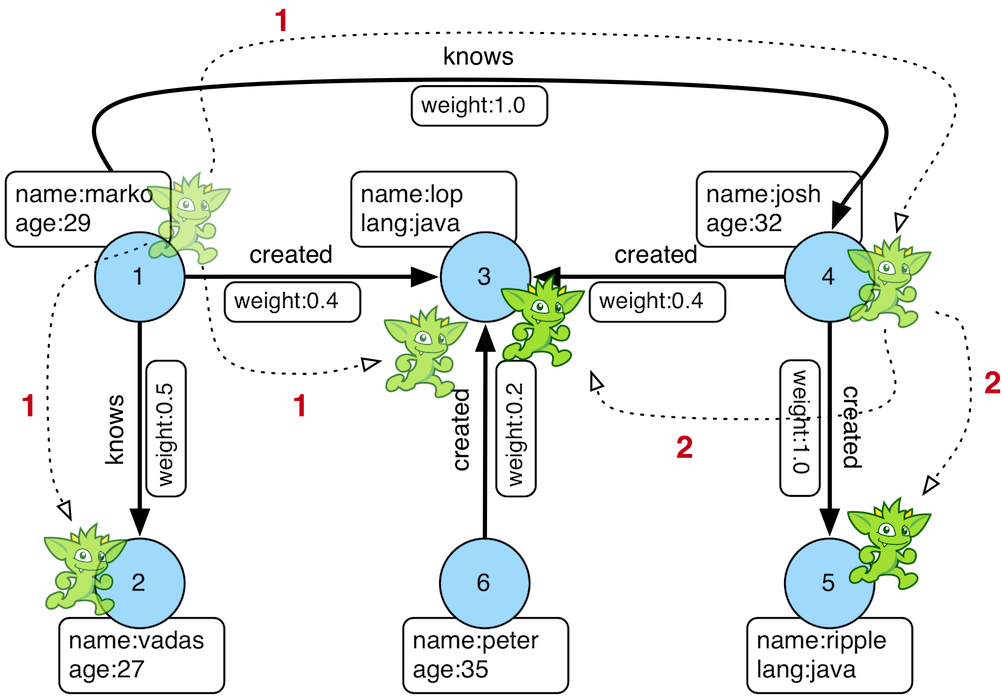``````gremlin> g.V(1).repeat(out()).times(2).emit().path().by('name')
==>[marko,lop]
==>[marko,josh]
==>[marko,josh,ripple]
==>[marko,josh,lop]``````
``g.V(1).repeat(out()).times(2).emit().path().by('name')``

The first time through the `repeat()`, the vertices lop, vadas, and josh are seen. Given that `loops==1`, the traverser repeats. However, because the emit-predicate is declared true, those vertices are emitted. The next time through `repeat()`, the vertices traversed are ripple and lop (Josh’s created projects, as lop and vadas have no out edges). Given that `loops==2`, the until-predicate fails and ripple and lop are emitted. Therefore, the traverser has seen the vertices: lop, vadas, josh, ripple, and lop.

`repeat()`-steps may be nested inside each other or inside the `emit()` or `until()` predicates and they can also be 'named' by passing a string as the first parameter to `repeat()`. The loop counter of a named repeat step can be accessed within the looped context with `loops(loopName)` where `loopName` is the name set whe creating the `repeat()`-step.

``````gremlin> g.V(1).
repeat(out("knows")).
until(repeat(out("created")).emit(has("name", "lop"))) //1\
==>v
gremlin> g.V(6).
repeat('a', both('created').simplePath()).
emit(repeat('b', both('knows')).
until(loops('b').as('b').where(loops('a').as('b'))).
hasId(2)).dedup() //2\
==>v``````
``````g.V(1).
repeat(out("knows")).
until(repeat(out("created")).emit(has("name", "lop"))) //1\
g.V(6).
repeat('a', both('created').simplePath()).
emit(repeat('b', both('knows')).
until(loops('b').as('b').where(loops('a').as('b'))).
hasId(2)).dedup() //2``````
1. Starting from vertex 1, keep going taking outgoing 'knows' edges until the vertex was created by 'lop'.

2. Starting from vertex 6, keep taking created edges in either direction until the vertex is same distance from vertex 2 over knows edges as it is from vertex 6 over created edges.

Finally, note that both `emit()` and `until()` can take a traversal and in such, situations, the predicate is determined by `traversal.hasNext()`. A few examples are provided below.

``````gremlin> g.V(1).repeat(out()).until(hasLabel('software')).path().by('name') //1\
==>[marko,lop]
==>[marko,josh,ripple]
==>[marko,josh,lop]
gremlin> g.V(1).emit(hasLabel('person')).repeat(out()).path().by('name') //2\
==>[marko]
==>[marko,josh]
gremlin> g.V(1).repeat(out()).until(outE().count().is(0)).path().by('name') //3\
==>[marko,lop]
==>[marko,josh,ripple]
==>[marko,josh,lop]``````
``````g.V(1).repeat(out()).until(hasLabel('software')).path().by('name') //1\
g.V(1).emit(hasLabel('person')).repeat(out()).path().by('name') //2\
g.V(1).repeat(out()).until(outE().count().is(0)).path().by('name') //3``````
1. Starting from vertex 1, keep taking outgoing edges until a software vertex is reached.

2. Starting from vertex 1, and in an infinite loop, emit the vertex if it is a person and then traverser the outgoing edges.

3. Starting from vertex 1, keep taking outgoing edges until a vertex is reached that has no more outgoing edges.

 Warning The anonymous traversal of `emit()` and `until()` (not `repeat()`) process their current objects "locally." In OLAP, where the atomic unit of computing is the vertex and its local "star graph," it is important that the anonymous traversals do not leave the confines of the vertex’s star graph. In other words, they can not traverse to an adjacent vertex’s properties or edges.

### Sack StepA traverser can contain a local data structure called a "sack". The `sack()`-step is used to read and write sacks (sideEffect or map). Each sack of each traverser is created when using `GraphTraversal.withSack(initialValueSupplier,splitOperator?,mergeOperator?)`.

• Initial value supplier: A `Supplier` providing the initial value of each traverser’s sack.

• Split operator: a `UnaryOperator` that clones the traverser’s sack when the traverser splits. If no split operator is provided, then `UnaryOperator.identity()` is assumed.

• Merge operator: A `BinaryOperator` that unites two traverser’s sack when they are merged. If no merge operator is provided, then traversers with sacks can not be merged.

Two trivial examples are presented below to demonstrate the initial value supplier. In the first example below, a traverser is created at each vertex in the graph (`g.V()`), with a 1.0 sack (`withSack(1.0f)`), and then the sack value is accessed (`sack()`). In the second example, a random float supplier is used to generate sack values.

``````gremlin> g.withSack(1.0f).V().sack()
==>1.0
==>1.0
==>1.0
==>1.0
==>1.0
==>1.0
gremlin> rand = new Random()
==>java.util.Random@751831f4
gremlin> g.withSack {rand.nextFloat()}.V().sack()
==>0.7704409
==>0.04574257
==>0.60025656
==>0.14623755
==>0.9399091
==>0.157359``````
``````g.withSack(1.0f).V().sack()
rand = new Random()
g.withSack {rand.nextFloat()}.V().sack()``````

A more complicated initial value supplier example is presented below where the sack values are used in a running computation and then emitted at the end of the traversal. When an edge is traversed, the edge weight is multiplied by the sack value (`sack(mult).by('weight')`). Note that the `by()`-modulator can be any arbitrary traversal.

``````gremlin> g.withSack(1.0f).V().repeat(outE().sack(mult).by('weight').inV()).times(2)
==>v
==>v
gremlin> g.withSack(1.0f).V().repeat(outE().sack(mult).by('weight').inV()).times(2).sack()
==>1.0
==>0.4
gremlin> g.withSack(1.0f).V().repeat(outE().sack(mult).by('weight').inV()).times(2).path().
by().by('weight')
==>[v,1.0,v,1.0,v]
==>[v,1.0,v,0.4,v]``````
``````g.withSack(1.0f).V().repeat(outE().sack(mult).by('weight').inV()).times(2)
g.withSack(1.0f).V().repeat(outE().sack(mult).by('weight').inV()).times(2).sack()
g.withSack(1.0f).V().repeat(outE().sack(mult).by('weight').inV()).times(2).path().
by().by('weight')``````When complex objects are used (i.e. non-primitives), then a split operator should be defined to ensure that each traverser gets a clone of its parent’s sack. The first example does not use a split operator and as such, the same map is propagated to all traversers (a global data structure). The second example, demonstrates how `Map.clone()` ensures that each traverser’s sack contains a unique, local sack.

``````gremlin> g.withSack {[:]}.V().out().out().
sack {m,v -> m[v.value('name')] = v.value('lang'); m}.sack() // BAD: single map
==>[ripple:java]
==>[ripple:java,lop:java]
gremlin> g.withSack {[:]}{it.clone()}.V().out().out().
sack {m,v -> m[v.value('name')] = v.value('lang'); m}.sack() // GOOD: cloned map
==>[ripple:java]
==>[lop:java]``````
``````g.withSack {[:]}.V().out().out().
sack {m,v -> m[v.value('name')] = v.value('lang'); m}.sack() // BAD: single map
g.withSack {[:]}{it.clone()}.V().out().out().
sack {m,v -> m[v.value('name')] = v.value('lang'); m}.sack() // GOOD: cloned map``````
 Note For primitives (i.e. integers, longs, floats, etc.), a split operator is not required as a primitives are encoded in the memory address of the sack, not as a reference to an object.

If a merge operator is not provided, then traversers with sacks can not be bulked. However, in many situations, merging the sacks of two traversers at the same location is algorithmically sound and good to provide so as to gain the bulking optimization. In the examples below, the binary merge operator is `Operator.sum`. Thus, when two traverser merge, their respective sacks are added together.

``````gremlin> g.withSack(1.0d).V(1).out('knows').in('knows') //1\
==>v
==>v
gremlin> g.withSack(1.0d).V(1).out('knows').in('knows').sack() //2\
==>1.0
==>1.0
gremlin> g.withSack(1.0d, sum).V(1).out('knows').in('knows').sack() //3\
==>2.0
==>2.0
gremlin> g.withSack(1.0d).V(1).local(outE('knows').barrier(normSack).inV()).in('knows').barrier() //4\
==>v
==>v
gremlin> g.withSack(1.0d).V(1).local(outE('knows').barrier(normSack).inV()).in('knows').barrier().sack() //5\
==>0.5
==>0.5
gremlin> g.withSack(1.0d,sum).V(1).local(outE('knows').barrier(normSack).inV()).in('knows').barrier().sack() //6\
==>1.0
==>1.0
gremlin> g.withBulk(false).withSack(1.0f,sum).V(1).local(outE('knows').barrier(normSack).inV()).in('knows').barrier().sack() //7\
==>1.0
gremlin> g.withBulk(false).withSack(1.0f).V(1).local(outE('knows').barrier(normSack).inV()).in('knows').barrier().sack() //8\
==>0.5
==>0.5
gremlin>``````
``````g.withSack(1.0d).V(1).out('knows').in('knows') //1\
g.withSack(1.0d).V(1).out('knows').in('knows').sack() //2\
g.withSack(1.0d, sum).V(1).out('knows').in('knows').sack() //3\
g.withSack(1.0d).V(1).local(outE('knows').barrier(normSack).inV()).in('knows').barrier() //4\
g.withSack(1.0d).V(1).local(outE('knows').barrier(normSack).inV()).in('knows').barrier().sack() //5\
g.withSack(1.0d,sum).V(1).local(outE('knows').barrier(normSack).inV()).in('knows').barrier().sack() //6\
g.withBulk(false).withSack(1.0f,sum).V(1).local(outE('knows').barrier(normSack).inV()).in('knows').barrier().sack() //7\
g.withBulk(false).withSack(1.0f).V(1).local(outE('knows').barrier(normSack).inV()).in('knows').barrier().sack() //8\``````
1. We find vertex 1 twice because he knows two other people

2. Without a merge operation the sack values are 1.0.

3. When specifying `sum` as the merge operation, the sack values are 2.0 because of bulking

4. Like 1, but using barrier internally

5. The `local(…​barrier(normSack)…​)` ensures that all traversers leaving vertex 1 have an evenly distributed amount of the initial 1.0 "energy" (50-50), i.e. the sack is 0.5 on each result

6. Like 3, but using `sum` as merge operator leads to the expected 1.0

7. There is now a single traverser with bulk of 2 and sack of 1.0 and thus, setting withBulk(false) yields the expected 1.0

8. Like 7, but without the sum operator

### Sample Step

The `sample()`-step is useful for sampling some number of traversers previous in the traversal.

``````gremlin> g.V().outE().sample(1).values('weight')
==>0.5
gremlin> g.V().outE().sample(1).by('weight').values('weight')
==>1.0
gremlin> g.V().outE().sample(2).by('weight').values('weight')
==>1.0
==>1.0``````
``````g.V().outE().sample(1).values('weight')
g.V().outE().sample(1).by('weight').values('weight')
g.V().outE().sample(2).by('weight').values('weight')``````

One of the more interesting use cases for `sample()` is when it is used in conjunction with `local()`. The combination of the two steps supports the execution of random walks. In the example below, the traversal starts are vertex 1 and selects one edge to traverse based on a probability distribution generated by the weights of the edges. The output is always a single path as by selecting a single edge, the traverser never splits and continues down a single path in the graph.

``````gremlin> g.V(1).repeat(local(
bothE().sample(1).by('weight').otherV()
)).times(5)
==>v
gremlin> g.V(1).repeat(local(
bothE().sample(1).by('weight').otherV()
)).times(5).path()
==>[v,e[1-knows->4],v,e[4-created->3],v,e[1-created->3],v,e[1-knows->4],v,e[4-created->5],v]
gremlin> g.V(1).repeat(local(
bothE().sample(1).by('weight').otherV()
)).times(10).path()
==>[v,e[1-created->3],v,e[1-created->3],v,e[1-knows->2],v,e[1-knows->2],v,e[1-knows->4],v,e[4-created->5],v,e[4-created->5],v,e[4-created->5],v,e[4-created->5],v,e[1-knows->4],v]``````
``````g.V(1).repeat(local(
bothE().sample(1).by('weight').otherV()
)).times(5)
g.V(1).repeat(local(
bothE().sample(1).by('weight').otherV()
)).times(5).path()
g.V(1).repeat(local(
bothE().sample(1).by('weight').otherV()
)).times(10).path()``````

As a clarification, note that in the above example `local()` is not strictly required as it only does the random walk over a single vertex, but note what happens without it if multiple vertices are traversed:

``````gremlin> g.V().repeat(bothE().sample(1).by('weight').otherV()).times(5).path()
==>[v,e[1-created->3],v,e[1-knows->2],v,e[1-knows->2],v,e[1-knows->4],v,e[4-created->3],v]``````
``g.V().repeat(bothE().sample(1).by('weight').otherV()).times(5).path()``

The use of `local()` ensures that the traversal over `bothE()` occurs once per vertex traverser that passes through, thus allowing one random walk per vertex.

``````gremlin> g.V().repeat(local(bothE().sample(1).by('weight').otherV())).times(5).path()
==>[v,e[1-created->3],v,e[4-created->3],v,e[4-created->3],v,e[4-created->3],v,e[4-created->3],v]
==>[v,e[1-knows->2],v,e[1-knows->2],v,e[1-knows->2],v,e[1-knows->4],v,e[4-created->5],v]
==>[v,e[4-created->3],v,e[4-created->3],v,e[1-created->3],v,e[1-created->3],v,e[4-created->3],v]
==>[v,e[4-created->3],v,e[4-created->3],v,e[4-created->3],v,e[4-created->3],v,e[4-created->5],v]
==>[v,e[4-created->5],v,e[4-created->3],v,e[1-created->3],v,e[1-created->3],v,e[4-created->3],v]
==>[v,e[6-created->3],v,e[4-created->3],v,e[4-created->5],v,e[4-created->5],v,e[4-created->3],v]``````
``g.V().repeat(local(bothE().sample(1).by('weight').otherV())).times(5).path()``

So, while not strictly required, it is likely better to be explicit with the use of `local()` so that the proper intent of the traversal is expressed.

### Select Step

Functional languages make use of function composition and lazy evaluation to create complex computations from primitive operations. This is exactly what `Traversal` does. One of the differentiating aspects of Gremlin’s data flow approach to graph processing is that the flow need not always go "forward," but in fact, can go back to a previously seen area of computation. Examples include `path()` as well as the `select()`-step (map). There are two general ways to use `select()`-step.

1. Select labeled steps within a path (as defined by `as()` in a traversal).

2. Select objects out of a `Map<String,Object>` flow (i.e. a sub-map).

The first use case is demonstrated via example below.

``````gremlin> g.V().as('a').out().as('b').out().as('c') // no select
==>v
==>v
gremlin> g.V().as('a').out().as('b').out().as('c').select('a','b','c')
==>[a:v,b:v,c:v]
==>[a:v,b:v,c:v]
gremlin> g.V().as('a').out().as('b').out().as('c').select('a','b')
==>[a:v,b:v]
==>[a:v,b:v]
gremlin> g.V().as('a').out().as('b').out().as('c').select('a','b').by('name')
==>[a:marko,b:josh]
==>[a:marko,b:josh]
gremlin> g.V().as('a').out().as('b').out().as('c').select('a') //1\
==>v
==>v``````
``````g.V().as('a').out().as('b').out().as('c') // no select
g.V().as('a').out().as('b').out().as('c').select('a','b','c')
g.V().as('a').out().as('b').out().as('c').select('a','b')
g.V().as('a').out().as('b').out().as('c').select('a','b').by('name')
g.V().as('a').out().as('b').out().as('c').select('a') //1``````
1. If the selection is one step, no map is returned.

When there is only one label selected, then a single object is returned. This is useful for stepping back in a computation and easily moving forward again on the object reverted to.

``````gremlin> g.V().out().out()
==>v
==>v
gremlin> g.V().out().out().path()
==>[v,v,v]
==>[v,v,v]
gremlin> g.V().as('x').out().out().select('x')
==>v
==>v
gremlin> g.V().out().as('x').out().select('x')
==>v
==>v
gremlin> g.V().out().out().as('x').select('x') // pointless
==>v
==>v``````
``````g.V().out().out()
g.V().out().out().path()
g.V().as('x').out().out().select('x')
g.V().out().as('x').out().select('x')
g.V().out().out().as('x').select('x') // pointless``````
 Note When executing a traversal with `select()` on a standard traversal engine (i.e. OLTP), `select()` will do its best to avoid calculating the path history and instead, will rely on a global data structure for storing the currently selected object. As such, if only a subset of the path walked is required, `select()` should be used over the more resource intensive `path()`-step.

When the set of keys or values (i.e. columns) of a path or map are needed, use `select(keys)` and `select(values)`, respectively. This is especially useful when one is only interested in the top N elements in a `groupCount()` ranking.

``````gremlin> g = graph.traversal()
==>graphtraversalsource[tinkergraph[vertices:0 edges:0], standard]
gremlin> g.V().hasLabel('song').out('followedBy').groupCount().by('name').
order(local).by(values,desc).limit(local, 5)
==>[PLAYING IN THE BAND:107,JACK STRAW:99,TRUCKING:94,DRUMS:92,ME AND MY UNCLE:86]
gremlin> g.V().hasLabel('song').out('followedBy').groupCount().by('name').
order(local).by(values,desc).limit(local, 5).select(keys)
==>[PLAYING IN THE BAND,JACK STRAW,TRUCKING,DRUMS,ME AND MY UNCLE]
gremlin> g.V().hasLabel('song').out('followedBy').groupCount().by('name').
order(local).by(values,desc).limit(local, 5).select(keys).unfold()
==>PLAYING IN THE BAND
==>JACK STRAW
==>TRUCKING
==>DRUMS
==>ME AND MY UNCLE``````
``````g = graph.traversal()
g.V().hasLabel('song').out('followedBy').groupCount().by('name').
order(local).by(values,desc).limit(local, 5)
g.V().hasLabel('song').out('followedBy').groupCount().by('name').
order(local).by(values,desc).limit(local, 5).select(keys)
g.V().hasLabel('song').out('followedBy').groupCount().by('name').
order(local).by(values,desc).limit(local, 5).select(keys).unfold()``````

Similarly, for extracting the values from a path or map.

``````gremlin> g = graph.traversal()
==>graphtraversalsource[tinkergraph[vertices:0 edges:0], standard]
gremlin> g.V().hasLabel('song').out('sungBy').groupCount().by('name') //1\
gremlin> g.V().hasLabel('song').out('sungBy').groupCount().by('name').select(values) //2\
==>[9,1,19,1,1,36,6,1,10,2,10,1,4,1,1,3,4,2,3,1,1,1,146,2,1,99,1,2,4,2,1,3,1,1,1,1,1,2,17,2,3,3,4,7,22,2,3,1,2,18,2,1,1,3,1,5,2,8,2,6]
gremlin> g.V().hasLabel('song').out('sungBy').groupCount().by('name').select(values).unfold().
groupCount().order(local).by(values,desc).limit(local, 5) //3\
==>[1:22,2:12,3:7,4:4,6:2]``````
``````g = graph.traversal()
g.V().hasLabel('song').out('sungBy').groupCount().by('name') //1\
g.V().hasLabel('song').out('sungBy').groupCount().by('name').select(values) //2\
g.V().hasLabel('song').out('sungBy').groupCount().by('name').select(values).unfold().
groupCount().order(local).by(values,desc).limit(local, 5) //3``````
1. Which artist sung how many songs?

2. Get an anonymized set of song repertoire sizes.

3. What are the 5 most common song repertoire sizes?

 Warning Note that `by()`-modulation is not supported with `select(keys)` and `select(values)`.

There is also an option to supply a `Pop` operation to `select()` to manipulate `List` objects in the `Traverser`:

``````gremlin> g.V(1).as("a").repeat(out().as("a")).times(2).select(first, "a")
==>v
==>v
gremlin> g.V(1).as("a").repeat(out().as("a")).times(2).select(last, "a")
==>v
==>v
gremlin> g.V(1).as("a").repeat(out().as("a")).times(2).select(all, "a")
==>[v,v,v]
==>[v,v,v]``````
``````g.V(1).as("a").repeat(out().as("a")).times(2).select(first, "a")
g.V(1).as("a").repeat(out().as("a")).times(2).select(last, "a")
g.V(1).as("a").repeat(out().as("a")).times(2).select(all, "a")``````

In addition to the previously shown examples, where `select()` was used to select an element based on a static key, `select()` can also accept a traversal that emits a key.

 Warning Since the key used by `select()` cannot be determined at compile time, the `TraversalSelectStep` enables full path tracking.
``````gremlin> g.withSideEffect("alias", ["marko":"okram"]).V(). //1\
values("name").sack(assign). //2\
optional(select("alias").select(sack())) //3\
==>okram
==>lop
==>josh
==>ripple
==>peter``````
``````g.withSideEffect("alias", ["marko":"okram"]).V(). //1\
values("name").sack(assign). //2\
optional(select("alias").select(sack()))         //3``````
1. Inject a name alias map and start the traversal from all vertices.

2. Select all `name` values and store them as the current traverser’s sack value.

3. Optionally select the alias for the current name from the injected map.

#### Using Where with Select

Like `match()`-step, it is possible to use `where()`, as where is a filter that processes `Map<String,Object>` streams.

``````gremlin> g.V().as('a').out('created').in('created').as('b').select('a','b').by('name') //1\
==>[a:marko,b:marko]
==>[a:marko,b:josh]
==>[a:marko,b:peter]
==>[a:josh,b:josh]
==>[a:josh,b:marko]
==>[a:josh,b:josh]
==>[a:josh,b:peter]
==>[a:peter,b:marko]
==>[a:peter,b:josh]
==>[a:peter,b:peter]
gremlin> g.V().as('a').out('created').in('created').as('b').
select('a','b').by('name').where('a',neq('b')) //2\
==>[a:marko,b:josh]
==>[a:marko,b:peter]
==>[a:josh,b:marko]
==>[a:josh,b:peter]
==>[a:peter,b:marko]
==>[a:peter,b:josh]
gremlin> g.V().as('a').out('created').in('created').as('b').
select('a','b'). //3\
where('a',neq('b')).
where(__.as('a').out('knows').as('b')).
select('a','b').by('name')
==>[a:marko,b:josh]``````
``````g.V().as('a').out('created').in('created').as('b').select('a','b').by('name') //1\
g.V().as('a').out('created').in('created').as('b').
select('a','b').by('name').where('a',neq('b')) //2\
g.V().as('a').out('created').in('created').as('b').
select('a','b'). //3\
where('a',neq('b')).
where(__.as('a').out('knows').as('b')).
select('a','b').by('name')``````
1. A standard `select()` that generates a `Map<String,Object>` of variables bindings in the path (i.e. `a` and `b`) for the sake of a running example.

2. The `select().by('name')` projects each binding vertex to their name property value and `where()` operates to ensure respective `a` and `b` strings are not the same.

3. The first `select()` projects a vertex binding set. A binding is filtered if `a` vertex equals `b` vertex. A binding is filtered if `a` doesn’t know `b`. The second and final `select()` projects the name of the vertices.

### ShortestPath step

The `shortestPath()`-step provides an easy way to find shortest non-cyclic paths in a graph. It is configurable using the `with()`-modulator with the options given below.

Key Type Description Default

`target`

`Traversal`

Sets a filter traversal for the end vertices (e.g. `__.has('name','marko')`).

all vertices (`__.identity()`)

`edges`

`Traversal` or `Direction`

Sets a `Traversal` that emits the edges to traverse from the current vertex or the `Direction` to traverse during the shortest path discovery.

`Direction.BOTH`

`distance`

`Traversal` or `String`

Sets the `Traversal` that calculates the distance for the current edge or the name of an edge property to use for the distance calculations.

`__.constant(1)`

`maxDistance`

`Number`

Sets the distance limit for all shortest paths.

none

`includeEdges`

`Boolean`

Whether to include edges in the result or not.

`false`

``````gremlin> g = g.withComputer()
==>graphtraversalsource[tinkergraph[vertices:6 edges:6], graphcomputer]
gremlin> g.V().shortestPath() //1\
==>[v,v]
==>[v,v,v]
==>[v,v,v]
==>[v,v]
==>[v,v]
==>[v]
==>[v,v,v]
==>[v,v,v,v]
==>[v,v,v,v]
==>[v]
==>[v,v,v]
==>[v,v]
==>[v,v]
==>[v,v,v]
==>[v,v]
==>[v,v,v]
==>[v]
==>[v,v]
==>[v]
==>[v,v]
==>[v,v,v]
==>[v,v,v]
==>[v,v]
==>[v,v]
==>[v,v]
==>[v]
==>[v,v,v,v]
==>[v,v,v,v]
==>[v,v,v]
==>[v,v,v]
==>[v,v,v]
==>[v,v,v,v]
==>[v]
==>[v,v,v,v]
==>[v,v]
==>[v,v,v]
gremlin> g.V().has('person','name','marko').shortestPath() //2\
==>[v,v]
==>[v]
==>[v,v]
==>[v,v]
==>[v,v,v]
==>[v,v,v]
gremlin> g.V().shortestPath().with(ShortestPath.target, __.has('name','peter')) //3\
==>[v,v,v]
==>[v,v,v,v]
==>[v,v]
==>[v,v,v]
==>[v,v,v,v]
==>[v]
gremlin> g.V().shortestPath().
with(ShortestPath.edges, Direction.IN).
with(ShortestPath.target, __.has('name','josh')) //4\
==>[v,v]
==>[v]
==>[v,v]
gremlin> g.V().has('person','name','marko').
shortestPath().
with(ShortestPath.target, __.has('name','josh')) //5\
==>[v,v]
gremlin> g.V().has('person','name','marko').
shortestPath().
with(ShortestPath.target, __.has('name','josh')).
with(ShortestPath.distance, 'weight') //6\
==>[v,v,v]
gremlin> g.V().has('person','name','marko').
shortestPath().
with(ShortestPath.target, __.has('name','josh')).
with(ShortestPath.includeEdges, true) //7\
==>[v,e[1-knows->4],v]``````
``````g = g.withComputer()
g.V().shortestPath() //1\
g.V().has('person','name','marko').shortestPath() //2\
g.V().shortestPath().with(ShortestPath.target, __.has('name','peter')) //3\
g.V().shortestPath().
with(ShortestPath.edges, Direction.IN).
with(ShortestPath.target, __.has('name','josh')) //4\
g.V().has('person','name','marko').
shortestPath().
with(ShortestPath.target, __.has('name','josh')) //5\
g.V().has('person','name','marko').
shortestPath().
with(ShortestPath.target, __.has('name','josh')).
with(ShortestPath.distance, 'weight') //6\
g.V().has('person','name','marko').
shortestPath().
with(ShortestPath.target, __.has('name','josh')).
with(ShortestPath.includeEdges, true) //7``````
1. Find all shortest paths.

2. Find all shortest paths from `marko`.

3. Find all shortest paths to `peter`.

4. Find all in-directed paths to `josh`.

5. Find all shortest paths from `marko` to `josh`.

6. Find all shortest paths from `marko` to `josh` using a custom distance property.

7. Find all shortest paths from `marko` to `josh` and include edges in the result.

``````gremlin> g.inject(g.withComputer().V().shortestPath().
with(ShortestPath.distance, 'weight').
with(ShortestPath.includeEdges, true).
with(ShortestPath.maxDistance, 1).toList().toArray()).
map(unfold().values('name','weight').fold()) //1\
==>[marko]
==>[marko,0.4,lop]
==>[marko,0.4,lop,0.4,josh]
==>[marko,0.4,lop,0.2,peter]
==>[josh,0.4,lop,0.4,marko]
==>[josh,0.4,lop]
==>[josh]
==>[josh,1.0,ripple]
==>[josh,0.4,lop,0.2,peter]
==>[ripple,1.0,josh]
==>[ripple]
==>[peter,0.2,lop,0.4,marko]
==>[peter,0.2,lop]
==>[peter,0.2,lop,0.4,josh]
==>[peter]
==>[lop,0.4,marko]
==>[lop]
==>[lop,0.4,josh]
==>[lop,0.2,peter]``````
``````g.inject(g.withComputer().V().shortestPath().
with(ShortestPath.distance, 'weight').
with(ShortestPath.includeEdges, true).
with(ShortestPath.maxDistance, 1).toList().toArray()).
map(unfold().values('name','weight').fold()) //1``````
1. Find all shortest paths using a custom distance property and limit the distance to 1. Inject the result into a OLTP `GraphTraversal` in order to be able to select properties from all elements in all paths.

### SimplePath Step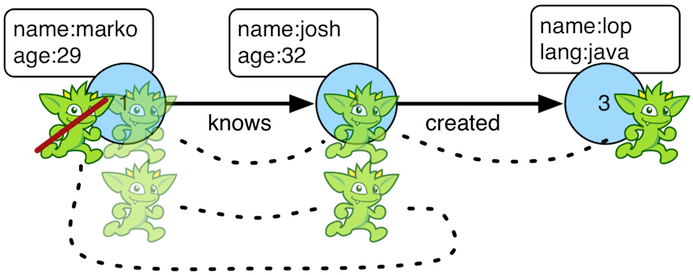When it is important that a traverser not repeat its path through the graph, `simplePath()`-step should be used (filter). The path information of the traverser is analyzed and if the path has repeated objects in it, the traverser is filtered. If cyclic behavior is desired, see `cyclicPath()`.

``````gremlin> g.V(1).both().both()
==>v
==>v
==>v
==>v
==>v
==>v
==>v
gremlin> g.V(1).both().both().simplePath()
==>v
==>v
==>v
==>v
gremlin> g.V(1).both().both().simplePath().path()
==>[v,v,v]
==>[v,v,v]
==>[v,v,v]
==>[v,v,v]
gremlin> g.V().out().as('a').out().as('b').out().as('c').
simplePath().by(label).
path()
gremlin> g.V().out().as('a').out().as('b').out().as('c').
simplePath().
by(label).
from('b').
to('c').
path().
by('name')``````
``````g.V(1).both().both()
g.V(1).both().both().simplePath()
g.V(1).both().both().simplePath().path()
g.V().out().as('a').out().as('b').out().as('c').
simplePath().by(label).
path()
g.V().out().as('a').out().as('b').out().as('c').
simplePath().
by(label).
from('b').
to('c').
path().
by('name')``````

By using the `from()` and `to()` modulators traversers can ensure that only certain sections of the path are acyclic.

``````gremlin> g.addV().property(id, 'A').as('a').
gremlin> g.V('A').repeat(both().simplePath()).times(3).path() //1\
==>[v[A],v[B],v[C],v[D]]
gremlin> g.V('D').repeat(both().simplePath()).times(3).path() //2\
==>[v[D],v[C],v[B],v[A]]
gremlin> g.V('A').as('a').
repeat(both().simplePath().from('a')).times(3).as('b').
repeat(both().simplePath().from('b')).times(3).path() //3\
==>[v[A],v[B],v[C],v[D],v[C],v[B],v[A]]``````
``````g.addV().property(id, 'A').as('a').
g.V('A').repeat(both().simplePath()).times(3).path() //1\
g.V('D').repeat(both().simplePath()).times(3).path() //2\
g.V('A').as('a').
repeat(both().simplePath().from('a')).times(3).as('b').
repeat(both().simplePath().from('b')).times(3).path()  //3``````
1. Traverse all acyclic 3-hop paths starting from vertex `A`

2. Traverse all acyclic 3-hop paths starting from vertex `D`

3. Traverse all acyclic 3-hop paths starting from vertex `A` and from there again all 3-hop paths. The second path may cross the vertices from the first path.

### Skip Step

The `skip()`-step is analogous to `range()`-step save that the higher end range is set to -1.

``````gremlin> g.V().values('age').order()
==>27
==>29
==>32
==>35
gremlin> g.V().values('age').order().skip(2)
==>32
==>35
gremlin> g.V().values('age').order().range(2, -1)
==>32
==>35``````
``````g.V().values('age').order()
g.V().values('age').order().skip(2)
g.V().values('age').order().range(2, -1)``````

The `skip()`-step can also be applied with `Scope.local`, in which case it operates on the incoming collection.

``````gremlin> g.V().hasLabel('person').filter(outE('created')).as('p'). //1\
map(out('created').values('name').fold()).
project('person','primary','other').
by(select('p').by('name')).
by(limit(local, 1)). //2\
by(skip(local, 1)) //3\
==>[person:marko,primary:lop,other:[]]
==>[person:josh,primary:ripple,other:[lop]]
==>[person:peter,primary:lop,other:[]]``````
``````g.V().hasLabel('person').filter(outE('created')).as('p'). //1\
map(out('created').values('name').fold()).
project('person','primary','other').
by(select('p').by('name')).
by(limit(local, 1)). //2\
by(skip(local, 1)) //3``````
1. For each person who created something…​

2. …​select the first project (random order) as `primary` and…​

3. …​select all other projects as `other`.

### Store Step

When lazy aggregation is needed, `store()`-step (sideEffect) should be used over `aggregate()`. The two steps differ in that `store()` does not block and only stores objects in its side-effect collection as they pass through.

``````gremlin> g.V().aggregate('x').limit(1).cap('x')
==>[v,v,v,v,v,v]
gremlin> g.V().store('x').limit(1).cap('x')
==>[v]
gremlin> g.withoutStrategies(EarlyLimitStrategy).V().store('x').limit(1).cap('x')
==>[v,v]``````
``````g.V().aggregate('x').limit(1).cap('x')
g.V().store('x').limit(1).cap('x')
g.withoutStrategies(EarlyLimitStrategy).V().store('x').limit(1).cap('x')``````

It is important to note that `EarlyLimitStrategy` introduced in 3.3.5 alters the behavior of `store()`. Without that strategy (which is installed by default), there are two results in the `store()` side-effect even though the interval selection is for 1 object. Realize that when the second object is on its way to the `range()` filter (i.e. `[0..1]`), it passes through `store()` and thus, stored before filtered.

``````gremlin> g.E().store('x').by('weight').cap('x')
==>[0.5,1.0,1.0,0.4,0.4,0.2]``````
``g.E().store('x').by('weight').cap('x')``

### Subgraph Step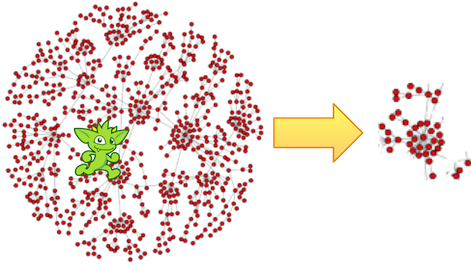Extracting a portion of a graph from a larger one for analysis, visualization or other purposes is a fairly common use case for graph analysts and developers. The `subgraph()`-step (sideEffect) provides a way to produce an edge-induced subgraph from virtually any traversal. The following example demonstrates how to produce the "knows" subgraph:

``````gremlin> subGraph = g.E().hasLabel('knows').subgraph('subGraph').cap('subGraph').next() //1\
==>tinkergraph[vertices:3 edges:2]
gremlin> sg = subGraph.traversal()
==>graphtraversalsource[tinkergraph[vertices:3 edges:2], standard]
gremlin> sg.E() //2\
==>e[1-knows->2]
==>e[1-knows->4]``````
``````subGraph = g.E().hasLabel('knows').subgraph('subGraph').cap('subGraph').next() //1\
sg = subGraph.traversal()
sg.E() //2``````
1. As this function produces "edge-induced" subgraphs, `subgraph()` must be called at edge steps.

2. The subgraph contains only "knows" edges.

A more common subgraphing use case is to get all of the graph structure surrounding a single vertex:

``````gremlin> subGraph = g.V(3).repeat(__.inE().subgraph('subGraph').outV()).times(3).cap('subGraph').next() //1\
==>tinkergraph[vertices:4 edges:4]
gremlin> sg = subGraph.traversal()
==>graphtraversalsource[tinkergraph[vertices:4 edges:4], standard]
gremlin> sg.E()
==>e[1-knows->4]
==>e[1-created->3]
==>e[4-created->3]
==>e[6-created->3]``````
``````subGraph = g.V(3).repeat(__.inE().subgraph('subGraph').outV()).times(3).cap('subGraph').next() //1\
sg = subGraph.traversal()
sg.E()``````
1. Starting at vertex `3`, traverse 3 steps away on in-edges, outputting all of that into the subgraph.

There can be multiple `subgraph()` calls within the same traversal. Each operating against either the same graph (i.e. same side-effect key) or different graphs (i.e. different side-effect keys).

``````gremlin> t = g.V().outE('knows').subgraph('knowsG').inV().outE('created').subgraph('createdG').
inV().inE('created').subgraph('createdG').iterate()
gremlin> t.sideEffects.get('knowsG').traversal().E()
==>e[1-knows->2]
==>e[1-knows->4]
gremlin> t.sideEffects.get('createdG').traversal().E()
==>e[1-created->3]
==>e[4-created->5]
==>e[4-created->3]
==>e[6-created->3]``````
``````t = g.V().outE('knows').subgraph('knowsG').inV().outE('created').subgraph('createdG').
inV().inE('created').subgraph('createdG').iterate()
t.sideEffects.get('knowsG').traversal().E()
t.sideEffects.get('createdG').traversal().E()``````

TinkerGraph is the ideal (and default) `Graph` into which a subgraph is extracted as it’s fast, in-memory, and supports user-supplied identifiers which can be any Java object. It is this last feature that needs some focus as many TinkerPop-enabled graphs have complex identifier types and TinkerGraph’s ability to consume those makes it a perfect host for an incoming subgraph. However care needs to be taken when using the elements of the TinkerGraph subgraph. The original graph’s identifiers may be preserved, but the elements of the graph are now TinkerGraph objects like, `TinkerVertex` and `TinkerEdge`. As a result, they can not be used directly in Gremlin running against the original graph. For example, the following traversal would likely return an error:

``````Vertex v = sg.V().has('name','marko').next();  //1
List<Vertex> vertices = g.V(v).out().toList(); //2``````
1. Here "sg" is a reference to a TinkerGraph subgraph and "v" is a `TinkerVertex`.

2. The `g.V(v)` has the potential to fail as "g" is the original `Graph` instance and not a TinkerGraph - it could reject the `TinkerVertex` instance as it will not recognize it.

It is safer to wrap the `TinkerVertex` in a `ReferenceVertex` or simply reference the `id()` as follows:

``````Vertex v = sg.V().has('name','marko').next();
List<Vertex> vertices = g.V(v.id()).out().toList();

// OR

Vertex v = new ReferenceVertex(sg.V().has('name','marko').next());
List<Vertex> vertices = g.V(v).out().toList();``````

### Sum Step

The `sum()`-step (map) operates on a stream of numbers and sums the numbers together to yield a result. Note that the current traverser number is multiplied by the traverser bulk to determine how many such numbers are being represented.

``````gremlin> g.V().values('age').sum()
==>123
gremlin> g.V().repeat(both()).times(3).values('age').sum()
==>1471``````
``````g.V().values('age').sum()
g.V().repeat(both()).times(3).values('age').sum()``````
 Important `sum(local)` determines the sum of the current, local object (not the objects in the traversal stream). This works for `Collection`-type objects.

### Tail Step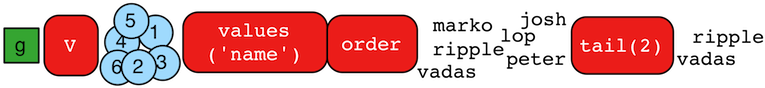The `tail()`-step is analogous to `limit()`-step, except that it emits the last `n`-objects instead of the first `n`-objects.

``````gremlin> g.V().values('name').order()
==>josh
==>lop
==>marko
==>peter
==>ripple
gremlin> g.V().values('name').order().tail() //1\
gremlin> g.V().values('name').order().tail(1) //2\
gremlin> g.V().values('name').order().tail(3) //3\
==>peter
==>ripple
``````g.V().values('name').order()
g.V().values('name').order().tail() //1\
g.V().values('name').order().tail(1) //2\
g.V().values('name').order().tail(3) //3``````
1. Last name (alphabetically).

2. Same as statement 1.

3. Last three names.

The `tail()`-step can also be applied with `Scope.local`, in which case it operates on the incoming collection.

``````gremlin> g.V().as('a').out().as('a').out().as('a').select('a').by(tail(local)).values('name') //1\
==>ripple
==>lop
gremlin> g.V().as('a').out().as('a').out().as('a').select('a').by(unfold().values('name').fold()).tail(local) //2\
==>ripple
==>lop
gremlin> g.V().as('a').out().as('a').out().as('a').select('a').by(unfold().values('name').fold()).tail(local, 2) //3\
==>[ripple]
==>[lop]
gremlin> g.V().valueMap().tail(local) //4\
==>[age:]
==>[age:]
==>[lang:[java]]
==>[age:]
==>[lang:[java]]
==>[age:]``````
``````g.V().as('a').out().as('a').out().as('a').select('a').by(tail(local)).values('name') //1\
g.V().as('a').out().as('a').out().as('a').select('a').by(unfold().values('name').fold()).tail(local) //2\
g.V().as('a').out().as('a').out().as('a').select('a').by(unfold().values('name').fold()).tail(local, 2) //3\
g.V().valueMap().tail(local) //4``````
1. Only the most recent name from the "a" step (`List<Vertex>` becomes `Vertex`).

2. Same result as statement 1 (`List<String>` becomes `String`).

3. `List<String>` for each path containing the last two names from the 'a' step.

4. `Map<String, Object>` for each vertex, but containing only the last property value.

### TimeLimit Step

In many situations, a graph traversal is not about getting an exact answer as its about getting a relative ranking. A classic example is recommendation. What is desired is a relative ranking of vertices, not their absolute rank. Next, it may be desirable to have the traversal execute for no more than 2 milliseconds. In such situations, `timeLimit()`-step (filter) can be used.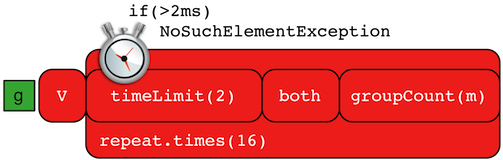Note The method `clock(int runs, Closure code)` is a utility preloaded in the Gremlin Console that can be used to time execution of a body of code.
``````gremlin> g.V().repeat(both().groupCount('m')).times(16).cap('m').order(local).by(values,desc).next()
==>v=2744208
==>v=2744208
==>v=2744208
==>v=1136688
==>v=1136688
==>v=1136688
gremlin> clock(1) {g.V().repeat(both().groupCount('m')).times(16).cap('m').order(local).by(values,desc).next()}
==>5.133465
gremlin> g.V().repeat(timeLimit(2).both().groupCount('m')).times(16).cap('m').order(local).by(values,desc).next()
==>v=2744208
==>v=2744208
==>v=2744208
==>v=1136688
==>v=1136688
==>v=1136688
gremlin> clock(1) {g.V().repeat(timeLimit(2).both().groupCount('m')).times(16).cap('m').order(local).by(values,desc).next()}
==>2.3989659999999997``````
``````g.V().repeat(both().groupCount('m')).times(16).cap('m').order(local).by(values,desc).next()
clock(1) {g.V().repeat(both().groupCount('m')).times(16).cap('m').order(local).by(values,desc).next()}
g.V().repeat(timeLimit(2).both().groupCount('m')).times(16).cap('m').order(local).by(values,desc).next()
clock(1) {g.V().repeat(timeLimit(2).both().groupCount('m')).times(16).cap('m').order(local).by(values,desc).next()}``````

In essence, the relative order is respected, even through the number of traversers at each vertex is not. The primary benefit being that the calculation is guaranteed to complete at the specified time limit (in milliseconds). Finally, note that the internal clock of `timeLimit()`-step starts when the first traverser enters it. When the time limit is reached, any `next()` evaluation of the step will yield a `NoSuchElementException` and any `hasNext()` evaluation will yield `false`.

### To Step

The `to()`-step is not an actual step, but instead is a "step-modulator" similar to `as()` and `by()`. If a step is able to accept traversals or strings then `to()` is the means by which they are added. The general pattern is `step().to()`. See `from()`-step.

The list of steps that support `to()`-modulation are: `simplePath()`, `cyclicPath()`, `path()`, and `addE()`.

### Tree Step

From any one element (i.e. vertex or edge), the emanating paths from that element can be aggregated to form a tree. Gremlin provides `tree()`-step (sideEffect) for such this situation.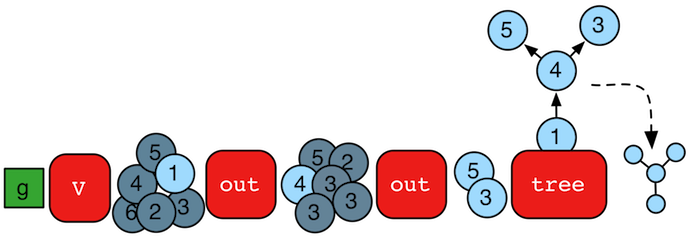``````gremlin> tree = g.V().out().out().tree().next()
==>v={v={v={}, v={}}}``````
``tree = g.V().out().out().tree().next()``

It is important to see how the paths of all the emanating traversers are united to form the tree.The resultant tree data structure can then be manipulated (see `Tree` JavaDoc).

``````gremlin> tree = g.V().out().out().tree().by('name').next()
==>marko={josh={ripple={}, lop={}}}
gremlin> tree['marko']
==>josh={ripple={}, lop={}}
gremlin> tree['marko']['josh']
==>ripple={}
==>lop={}
gremlin> tree.getObjectsAtDepth(3)
==>ripple
==>lop``````
``````tree = g.V().out().out().tree().by('name').next()
tree['marko']
tree['marko']['josh']
tree.getObjectsAtDepth(3)``````

Note that when using `by()`-modulation, tree nodes are combined based on projection uniqueness, not on the uniqueness of the original objects being projected. For instance:

``````gremlin> g.V().has('name','josh').out('created').values('name').tree() //1\
==>[v:[v:[lop:[]],v:[ripple:[]]]]
gremlin> g.V().has('name','josh').out('created').values('name').
tree().by('name').by(label).by() //2\
==>[josh:[software:[ripple:[],lop:[]]]]``````
``````g.V().has('name','josh').out('created').values('name').tree() //1\
g.V().has('name','josh').out('created').values('name').
tree().by('name').by(label).by() //2``````
1. When the `tree()` is created, vertex 3 and 5 are unique and thus, form unique branches in the tree structure.

2. When the `tree()` is `by()`-modulated by `label`, then vertex 3 and 5 are both "software" and thus are merged to a single node in the tree.

### Unfold Step

If the object reaching `unfold()` (flatMap) is an iterator, iterable, or map, then it is unrolled into a linear form. If not, then the object is simply emitted. Please see `fold()` step for the inverse behavior.

``````gremlin> g.V(1).out().fold().inject('gremlin',[1.23,2.34])
==>gremlin
==>[1.23,2.34]
==>[v,v,v]
gremlin> g.V(1).out().fold().inject('gremlin',[1.23,2.34]).unfold()
==>gremlin
==>1.23
==>2.34
==>v
==>v
==>v``````
``````g.V(1).out().fold().inject('gremlin',[1.23,2.34])
g.V(1).out().fold().inject('gremlin',[1.23,2.34]).unfold()``````

Note that `unfold()` does not recursively unroll iterators. Instead, `repeat()` can be used to for recursive unrolling.

``````gremlin> inject(1,[2,3,[4,5,]])
==>1
==>[2,3,[4,5,]]
gremlin> inject(1,[2,3,[4,5,]]).unfold()
==>1
==>2
==>3
==>[4,5,]
gremlin> inject(1,[2,3,[4,5,]]).repeat(unfold()).until(count(local).is(1)).unfold()
==>1
==>2
==>3
==>4
==>5
==>6``````
``````inject(1,[2,3,[4,5,]])
inject(1,[2,3,[4,5,]]).unfold()
inject(1,[2,3,[4,5,]]).repeat(unfold()).until(count(local).is(1)).unfold()``````

### Union Step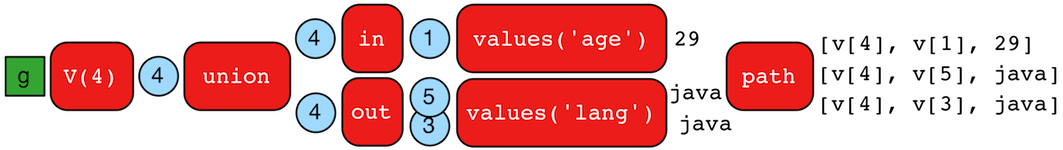The `union()`-step (branch) supports the merging of the results of an arbitrary number of traversals. When a traverser reaches a `union()`-step, it is copied to each of its internal steps. The traversers emitted from `union()` are the outputs of the respective internal traversals.

``````gremlin> g.V(4).union(
__.in().values('age'),
out().values('lang'))
==>29
==>java
==>java
gremlin> g.V(4).union(
__.in().values('age'),
out().values('lang')).path()
==>[v,v,29]
==>[v,v,java]
==>[v,v,java]``````
``````g.V(4).union(
__.in().values('age'),
out().values('lang'))
g.V(4).union(
__.in().values('age'),
out().values('lang')).path()``````

### Until Step

The `until`-step is not an actual step, but is instead a step modulator for `repeat()` (find more documentation on the `until()` there).

### Value Step

The `value()`-step (map) takes a `Property` and extracts the value from it.

``````gremlin> g.V(1).properties().value()
==>marko
==>san diego
==>santa cruz
==>brussels
==>santa fe
gremlin> g.V(1).properties().properties().value()
==>1997
==>2001
==>2001
==>2004
==>2004
==>2005
==>2005``````
``````g.V(1).properties().value()
g.V(1).properties().properties().value()``````

### ValueMap Step

The `valueMap()`-step yields a Map representation of the properties of an element.

``````gremlin> g.V().valueMap()
==>[name:[marko],age:]
==>[name:[lop],lang:[java]]
==>[name:[josh],age:]
==>[name:[ripple],lang:[java]]
==>[name:[peter],age:]
gremlin> g.V().valueMap('age')
==>[age:]
==>[age:]
==>[]
==>[age:]
==>[]
==>[age:]
gremlin> g.V().valueMap('age','blah')
==>[age:]
==>[age:]
==>[]
==>[age:]
==>[]
==>[age:]
gremlin> g.E().valueMap()
==>[weight:0.5]
==>[weight:1.0]
==>[weight:0.4]
==>[weight:1.0]
==>[weight:0.4]
==>[weight:0.2]``````
``````g.V().valueMap()
g.V().valueMap('age')
g.V().valueMap('age','blah')
g.E().valueMap()``````

It is important to note that the map of a vertex maintains a list of values for each key. The map of an edge or vertex-property represents a single property (not a list). The reason is that vertices in TinkerPop leverage vertex properties which support multiple values per key. Using the The Crew toy graph, the point is made explicit.

``````gremlin> g.V().valueMap()
==>[name:[marko],location:[san diego,santa cruz,brussels,santa fe]]
==>[name:[stephen],location:[centreville,dulles,purcellville]]
==>[name:[matthias],location:[bremen,baltimore,oakland,seattle]]
==>[name:[daniel],location:[spremberg,kaiserslautern,aachen]]
==>[name:[gremlin]]
==>[name:[tinkergraph]]
gremlin> g.V().has('name','marko').properties('location')
==>vp[location->san diego]
==>vp[location->santa cruz]
==>vp[location->brussels]
==>vp[location->santa fe]
gremlin> g.V().has('name','marko').properties('location').valueMap()
==>[startTime:1997,endTime:2001]
==>[startTime:2001,endTime:2004]
==>[startTime:2004,endTime:2005]
==>[startTime:2005]``````
``````g.V().valueMap()
g.V().has('name','marko').properties('location')
g.V().has('name','marko').properties('location').valueMap()``````

To turn list of values into single items, the `by()` modulator can be used as shown below.

``````gremlin> g.V().valueMap().by(unfold())
==>[name:marko,location:san diego]
==>[name:stephen,location:centreville]
==>[name:matthias,location:bremen]
==>[name:daniel,location:spremberg]
==>[name:gremlin]
==>[name:tinkergraph]
gremlin> g.V().valueMap('name','location').by().by(unfold())
==>[name:[marko],location:san diego]
==>[name:[stephen],location:centreville]
==>[name:[matthias],location:bremen]
==>[name:[daniel],location:spremberg]
==>[name:[gremlin]]
==>[name:[tinkergraph]]``````
``````g.V().valueMap().by(unfold())
g.V().valueMap('name','location').by().by(unfold())``````

If the `id`, `label`, `key`, and `value` of the `Element` is desired, then the `with()` modulator can be used to trigger its insertion into the returned map.

``````gremlin> g.V().hasLabel('person').valueMap().with(WithOptions.tokens)
==>[id:1,label:person,name:[marko],location:[san diego,santa cruz,brussels,santa fe]]
==>[id:7,label:person,name:[stephen],location:[centreville,dulles,purcellville]]
==>[id:8,label:person,name:[matthias],location:[bremen,baltimore,oakland,seattle]]
==>[id:9,label:person,name:[daniel],location:[spremberg,kaiserslautern,aachen]]
gremlin> g.V().hasLabel('person').valueMap('name').with(WithOptions.tokens, WithOptions.labels)
==>[label:person,name:[marko]]
==>[label:person,name:[stephen]]
==>[label:person,name:[matthias]]
==>[label:person,name:[daniel]]
gremlin> g.V().hasLabel('person').properties('location').valueMap().with(WithOptions.tokens, WithOptions.values)
==>[value:san diego,startTime:1997,endTime:2001]
==>[value:santa cruz,startTime:2001,endTime:2004]
==>[value:brussels,startTime:2004,endTime:2005]
==>[value:santa fe,startTime:2005]
==>[value:centreville,startTime:1990,endTime:2000]
==>[value:dulles,startTime:2000,endTime:2006]
==>[value:purcellville,startTime:2006]
==>[value:bremen,startTime:2004,endTime:2007]
==>[value:baltimore,startTime:2007,endTime:2011]
==>[value:oakland,startTime:2011,endTime:2014]
==>[value:seattle,startTime:2014]
==>[value:spremberg,startTime:1982,endTime:2005]
==>[value:kaiserslautern,startTime:2005,endTime:2009]
==>[value:aachen,startTime:2009]``````
``````g.V().hasLabel('person').valueMap().with(WithOptions.tokens)
g.V().hasLabel('person').valueMap('name').with(WithOptions.tokens, WithOptions.labels)
g.V().hasLabel('person').properties('location').valueMap().with(WithOptions.tokens, WithOptions.values)``````

### Values Step

The `values()`-step (map) extracts the values of properties from an `Element` in the traversal stream.

``````gremlin> g.V(1).values()
==>marko
==>san diego
==>santa cruz
==>brussels
==>santa fe
gremlin> g.V(1).values('location')
==>san diego
==>santa cruz
==>brussels
==>santa fe
gremlin> g.V(1).properties('location').values()
==>1997
==>2001
==>2001
==>2004
==>2004
==>2005
==>2005``````
``````g.V(1).values()
g.V(1).values('location')
g.V(1).properties('location').values()``````

### Vertex Steps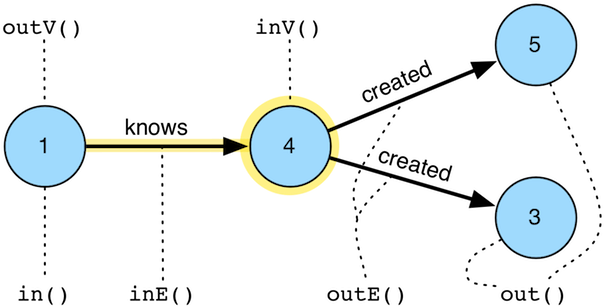The vertex steps (flatMap) are fundamental to the Gremlin language. Via these steps, its possible to "move" on the graph — i.e. traverse.

• `out(string…​)`: Move to the outgoing adjacent vertices given the edge labels.

• `in(string…​)`: Move to the incoming adjacent vertices given the edge labels.

• `both(string…​)`: Move to both the incoming and outgoing adjacent vertices given the edge labels.

• `outE(string…​)`: Move to the outgoing incident edges given the edge labels.

• `inE(string…​)`: Move to the incoming incident edges given the edge labels.

• `bothE(string…​)`: Move to both the incoming and outgoing incident edges given the edge labels.

• `outV()`: Move to the outgoing vertex.

• `inV()`: Move to the incoming vertex.

• `bothV()`: Move to both vertices.

• `otherV()` : Move to the vertex that was not the vertex that was moved from.

 Groovy The term `in` is a reserved word in Groovy, and when therefore used as part of an anonymous traversal must be referred to in Gremlin with the double underscore `__.in()`.
 Javascript The term `in` is a reserved word in Python, and therefore must be referred to in Gremlin with `in_()`.
 Python The term `in` is a reserved word in Python, and therefore must be referred to in Gremlin with `in_()`.
``````gremlin> g.V(4)
==>v
gremlin> g.V(4).outE() //1\
==>e[4-created->5]
==>e[4-created->3]
gremlin> g.V(4).inE('knows') //2\
==>e[1-knows->4]
gremlin> g.V(4).inE('created') //3\
gremlin> g.V(4).bothE('knows','created','blah')
==>e[4-created->5]
==>e[4-created->3]
==>e[1-knows->4]
gremlin> g.V(4).bothE('knows','created','blah').otherV()
==>v
==>v
==>v
gremlin> g.V(4).both('knows','created','blah')
==>v
==>v
==>v
gremlin> g.V(4).outE().inV() //4\
==>v
==>v
gremlin> g.V(4).out() //5\
==>v
==>v
gremlin> g.V(4).inE().outV()
==>v
gremlin> g.V(4).inE().bothV()
==>v
==>v``````
``````g.V(4)
g.V(4).outE() //1\
g.V(4).inE('knows') //2\
g.V(4).inE('created') //3\
g.V(4).bothE('knows','created','blah')
g.V(4).bothE('knows','created','blah').otherV()
g.V(4).both('knows','created','blah')
g.V(4).outE().inV() //4\
g.V(4).out() //5\
g.V(4).inE().outV()
g.V(4).inE().bothV()``````
1. All outgoing edges.

2. All incoming knows-edges.

3. All incoming created-edges.

4. Moving forward touching edges and vertices.

5. Moving forward only touching vertices.

### Where Step

The `where()`-step filters the current object based on either the object itself (`Scope.local`) or the path history of the object (`Scope.global`) (filter). This step is typically used in conjunction with either `match()`-step or `select()`-step, but can be used in isolation.

``````gremlin> g.V(1).as('a').out('created').in('created').where(neq('a')) //1\
==>v
==>v
gremlin> g.withSideEffect('a',['josh','peter']).V(1).out('created').in('created').values('name').where(within('a')) //2\
==>josh
==>peter
gremlin> g.V(1).out('created').in('created').where(out('created').count().is(gt(1))).values('name') //3\
==>josh``````
``````g.V(1).as('a').out('created').in('created').where(neq('a')) //1\
g.withSideEffect('a',['josh','peter']).V(1).out('created').in('created').values('name').where(within('a')) //2\
g.V(1).out('created').in('created').where(out('created').count().is(gt(1))).values('name') //3``````
1. Who are marko’s collaborators, where marko can not be his own collaborator? (predicate)

2. Of the co-creators of marko, only keep those whose name is josh or peter. (using a sideEffect)

3. Which of marko’s collaborators have worked on more than 1 project? (using a traversal)

 Important Please see `match().where()` and `select().where()` for how `where()` can be used in conjunction with `Map` projecting steps — i.e. `Scope.local`.

A few more examples of filtering an arbitrary object based on a anonymous traversal is provided below.

``````gremlin> g.V().where(out('created')).values('name') //1\
==>marko
==>josh
==>peter
gremlin> g.V().out('knows').where(out('created')).values('name') //2\
==>josh
gremlin> g.V().where(out('created').count().is(gte(2))).values('name') //3\
==>josh
gremlin> g.V().where(out('knows').where(out('created'))).values('name') //4\
==>marko
gremlin> g.V().where(__.not(out('created'))).where(__.in('knows')).values('name') //5\
gremlin> g.V().where(__.not(out('created')).and().in('knows')).values('name') //6\
gremlin> g.V().as('a').out('knows').as('b').
where('a',gt('b')).
by('age').
select('a','b').
by('name') //7\
gremlin> g.V().as('a').out('knows').as('b').
where('a',gt('b').or(eq('b'))).
by('age').
by('age').
by(__.in('knows').values('age')).
select('a','b').
by('name') //8\
==>[a:marko,b:josh]``````
``````g.V().where(out('created')).values('name') //1\
g.V().out('knows').where(out('created')).values('name') //2\
g.V().where(out('created').count().is(gte(2))).values('name') //3\
g.V().where(out('knows').where(out('created'))).values('name') //4\
g.V().where(__.not(out('created'))).where(__.in('knows')).values('name') //5\
g.V().where(__.not(out('created')).and().in('knows')).values('name') //6\
g.V().as('a').out('knows').as('b').
where('a',gt('b')).
by('age').
select('a','b').
by('name') //7\
g.V().as('a').out('knows').as('b').
where('a',gt('b').or(eq('b'))).
by('age').
by('age').
by(__.in('knows').values('age')).
select('a','b').
by('name') //8``````
1. What are the names of the people who have created a project?

2. What are the names of the people that are known by someone one and have created a project?

3. What are the names of the people how have created two or more projects?

4. What are the names of the people who know someone that has created a project? (This only works in OLTP — see the `WARNING` below)

5. What are the names of the people who have not created anything, but are known by someone?

6. The concatenation of `where()`-steps is the same as a single `where()`-step with an and’d clause.

7. Marko knows josh and vadas but is only older than vadas.

8. Marko is younger than josh, but josh knows someone equal in age to marko (which is marko).

 Warning The anonymous traversal of `where()` processes the current object "locally". In OLAP, where the atomic unit of computing is the vertex and its local "star graph," it is important that the anonymous traversal does not leave the confines of the vertex’s star graph. In other words, it can not traverse to an adjacent vertex’s properties or edges.

### With Step

The `with()`-step is not an actual step, but is instead a "step modulator" which modifies the behavior of the step prior to it. The `with()`-step provides additional "configuration" information to steps that implement the `Configuring` interface. Steps that allow for this type of modulation will explicitly state so in their documentation.

## A Note on Predicates

A `P` is a predicate of the form `Function<Object,Boolean>`. That is, given some object, return true or false. As of the relase of TinkerPop 3.4.0, Gremlin also supports simple text predicates, which only work on `String` values. The `TextP` text predicates extend the `P` predicates, but are specialized in that they are of the form `Function<String,Boolean>`. The provided predicates are outlined in the table below and are used in various steps such as `has()`-step, `where()`-step, `is()`-step, etc.

Predicate Description

`P.eq(object)`

Is the incoming object equal to the provided object?

`P.neq(object)`

Is the incoming object not equal to the provided object?

`P.lt(number)`

Is the incoming number less than the provided number?

`P.lte(number)`

Is the incoming number less than or equal to the provided number?

`P.gt(number)`

Is the incoming number greater than the provided number?

`P.gte(number)`

Is the incoming number greater than or equal to the provided number?

`P.inside(number,number)`

Is the incoming number greater than the first provided number and less than the second?

`P.outside(number,number)`

Is the incoming number less than the first provided number or greater than the second?

`P.between(number,number)`

Is the incoming number greater than or equal to the first provided number and less than the second?

`P.within(objects…​)`

Is the incoming object in the array of provided objects?

`P.without(objects…​)`

Is the incoming object not in the array of the provided objects?

`TextP.startingWith(string)`

Does the incoming `String` start with the provided `String`?

`TextP.endingWith(string)`

Does the incoming `String` end with the provided `String`?

`TextP.containing(string)`

Does the incoming `String` contain the provided `String`?

`TextP.notStartingWith(string)`

Does the incoming `String` not start with the provided `String`?

`TextP.notEndingWith(string)`

Does the incoming `String` not end with the provided `String`?

`TextP.notContaining(string)`

Does the incoming `String` not contain the provided `String`?

``````gremlin> eq(2)
==>eq(2)
gremlin> not(neq(2)) //1\
==>eq(2)
gremlin> not(within('a','b','c'))
==>without([a, b, c])
gremlin> not(within('a','b','c')).test('d') //2\
==>true
gremlin> not(within('a','b','c')).test('a')
==>false
gremlin> within(1,2,3).and(not(eq(2))).test(3) //3\
==>true
gremlin> inside(1,4).or(eq(5)).test(3) //4\
==>true
gremlin> inside(1,4).or(eq(5)).test(5)
==>true
gremlin> between(1,2) //5\
==>and(gte(1), lt(2))
gremlin> not(between(1,2))
==>or(lt(1), gte(2))``````
``````eq(2)
not(neq(2)) //1\
not(within('a','b','c'))
not(within('a','b','c')).test('d') //2\
not(within('a','b','c')).test('a')
within(1,2,3).and(not(eq(2))).test(3) //3\
inside(1,4).or(eq(5)).test(3) //4\
inside(1,4).or(eq(5)).test(5)
between(1,2) //5\
not(between(1,2))``````
1. The `not()` of a `P`-predicate is another `P`-predicate.

2. `P`-predicates are arguments to various steps which internally `test()` the incoming value.

3. `P`-predicates can be and’d together.

4. `P`-predicates can be or' together.

5. `and()` is a `P`-predicate and thus, a `P`-predicate can be composed of multiple `P`-predicates.

 Tip To reduce the verbosity of predicate expressions, it is good to `import static org.apache.tinkerpop.gremlin.process.traversal.P.*`.

Finally, note that `where()`-step takes a `P<String>`. The provided string value refers to a variable binding, not to the explicit string value.

``````gremlin> g.V().as('a').both().both().as('b').count()
==>30
gremlin> g.V().as('a').both().both().as('b').where('a',neq('b')).count()
==>18``````
``````g.V().as('a').both().both().as('b').count()
g.V().as('a').both().both().as('b').where('a',neq('b')).count()``````
 Note It is possible for graph system providers and users to extend `P` and provide new predicates. For instance, a `regex(pattern)` could be a graph system specific `P`.

## A Note on Barrier Steps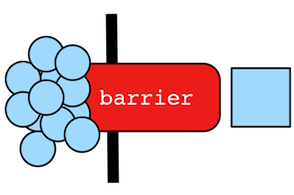Gremlin is primarily a lazy, stream processing language. This means that Gremlin fully processes (to the best of its abilities) any traversers currently in the traversal pipeline before getting more data from the start/head of the traversal. However, there are numerous situations in which a completely lazy computation is not possible (or impractical). When a computation is not lazy, a "barrier step" exists. There are three types of barriers:

1. `CollectingBarrierStep`: All of the traversers prior to the step are put into a collection and then processed in some way (e.g. ordered) prior to the collection being "drained" one-by-one to the next step. Examples include: `order()`, `sample()`, `aggregate()`, `barrier()`.

2. `ReducingBarrierStep`: All of the traversers prior to the step are processed by a reduce function and once all the previous traversers are processed, a single "reduced value" traverser is emitted to the next step. Note that the path history leading up to a reducing barrier step is destroyed given its many-to-one nature. Examples include: `fold()`, `count()`, `sum()`, `max()`, `min()`.

3. `SupplyingBarrierStep`: All of the traversers prior to the step are iterated (no processing) and then some provided supplier yields a single traverser to continue to the next step. Examples include: `cap()`.

In Gremlin OLAP (see `TraversalVertexProgram`), a barrier is introduced at the end of every adjacent vertex step. This means that the traversal does its best to compute as much as possible at the current, local vertex. What it can’t compute without referencing an adjacent vertex is aggregated into a barrier collection. When there are no more traversers at the local vertex, the barriered traversers are the messages that are propagated to remote vertices for further processing.

## A Note on Scopes

The `Scope` enum has two constants: `Scope.local` and `Scope.global`. Scope determines whether the particular step being scoped is with respects to the current object (`local`) at that step or to the entire stream of objects up to that step (`global`).

 Python The term `global` is a reserved word in Python, and therefore a `Scope` using that term must be referred as `global_`.
``````gremlin> g.V().has('name','marko').out('knows').count() //1\
==>2
gremlin> g.V().has('name','marko').out('knows').fold().count() //2\
==>1
gremlin> g.V().has('name','marko').out('knows').fold().count(local) //3\
==>2
gremlin> g.V().has('name','marko').out('knows').fold().count(global) //4\
==>1``````
``````g.V().has('name','marko').out('knows').count() //1\
g.V().has('name','marko').out('knows').fold().count() //2\
g.V().has('name','marko').out('knows').fold().count(local) //3\
g.V().has('name','marko').out('knows').fold().count(global) //4``````
1. Marko knows 2 people.

2. A list of Marko’s friends is created and thus, one object is counted (the single list).

3. A list of Marko’s friends is created and a `local`-count yields the number of objects in that list.

4. `count(global)` is the same as `count()` as the default behavior for most scoped steps is `global`.

The steps that support scoping are:

A few more examples of the use of `Scope` are provided below:

``````gremlin> g.V().both().group().by(label).select('software').dedup(local)
==>[v,v]
gremlin> g.V().groupCount().by(label).select(values).min(local)
==>2
gremlin> g.V().groupCount().by(label).order(local).by(values,desc)
==>[person:4,software:2]
gremlin> g.V().fold().sample(local,2)
==>[v,v]``````
``````g.V().both().group().by(label).select('software').dedup(local)
g.V().groupCount().by(label).select(values).min(local)
g.V().groupCount().by(label).order(local).by(values,desc)
g.V().fold().sample(local,2)``````

Finally, note that `local()`-step is a "hard-scoped step" that transforms any internal traversal into a locally-scoped operation. A contrived example is provided below:

``````gremlin> g.V().fold().local(unfold().count())
==>6
gremlin> g.V().fold().count(local)
==>6``````
``````g.V().fold().local(unfold().count())
g.V().fold().count(local)``````

## A Note On LambdasA lambda is a function that can be referenced by software and thus, passed around like any other piece of data. In Gremlin, lambdas make it possible to generalize the behavior of a step such that custom steps can be created (on-the-fly) by the user. However, it is advised to avoid using lambdas if possible.

``````gremlin> g.V().filter{it.get().value('name') == 'marko'}.
flatMap{it.get().vertices(OUT,'created')}.
map {it.get().value('name')} //1\
==>lop
gremlin> g.V().has('name','marko').out('created').values('name') //2\
==>lop``````
``````g.V().filter{it.get().value('name') == 'marko'}.
flatMap{it.get().vertices(OUT,'created')}.
map {it.get().value('name')} //1\
g.V().has('name','marko').out('created').values('name') //2``````
1. A lambda-rich Gremlin traversal which should and can be avoided. (bad)

2. The same traversal (result), but without using lambdas. (good)

Gremlin attempts to provide the user a comprehensive collection of steps in the hopes that the user will never need to leverage a lambda in practice. It is advised that users only leverage a lambda if and only if there is no corresponding lambda-less step that encompasses the desired functionality. The reason being, lambdas can not be optimized by Gremlin’s compiler strategies as they can not be programmatically inspected (see traversal strategies). It is also not currently possible to send a natively written lambda for remote execution to Gremlin-Server or a driver that supports remote execution.

In many situations where a lambda could be used, either a corresponding step exists or a traversal can be provided in its place. A `TraversalLambda` behaves like a typical lambda, but it can be optimized and it yields less objects than the corresponding pure-lambda form.

``````gremlin> g.V().out().out().path().by {it.value('name')}.
by {it.value('name')}.
by {g.V(it).in('created').values('name').fold().next()} //1\
==>[marko,josh,[josh]]
==>[marko,josh,[marko,josh,peter]]
gremlin> g.V().out().out().path().by('name').
by('name').
by(__.in('created').values('name').fold()) //2\
==>[marko,josh,[josh]]
==>[marko,josh,[marko,josh,peter]]``````
``````g.V().out().out().path().by {it.value('name')}.
by {it.value('name')}.
by {g.V(it).in('created').values('name').fold().next()} //1\
g.V().out().out().path().by('name').
by('name').
by(__.in('created').values('name').fold()) //2``````
1. The length-3 paths have each of their objects transformed by a lambda. (bad)

2. The length-3 paths have their objects transformed by a lambda-less step and a traversal lambda. (good)

## TraversalStrategyA `TraversalStrategy` analyzes a `Traversal` and, if the traversal meets its criteria, can mutate it accordingly. Traversal strategies are executed at compile-time and form the foundation of the Gremlin traversal machine’s compiler. There are 5 categories of strategies which are itemized below:

• There is an application-level feature that can be embedded into the traversal logic (decoration).

• There is a more efficient way to express the traversal at the TinkerPop level (optimization).

• There is a more efficient way to express the traversal at the graph system/language/driver level (provider optimization).

• There are some final adjustments/cleanups/analyses required before executing the traversal (finalization).

• There are certain traversals that are not legal for the application or traversal engine (verification).

 Note The `explain()`-step shows the user how each registered strategy mutates the traversal.

A simple `OptimizationStrategy` is the `IdentityRemovalStrategy`.

``````public final class IdentityRemovalStrategy extends AbstractTraversalStrategy<TraversalStrategy.OptimizationStrategy> implements TraversalStrategy.OptimizationStrategy {

private static final IdentityRemovalStrategy INSTANCE = new IdentityRemovalStrategy();

private IdentityRemovalStrategy() {
}

@Override
public void apply(Traversal.Admin<?, ?> traversal) {
if (traversal.getSteps().size() <= 1)
return;

for (IdentityStep<?> identityStep : TraversalHelper.getStepsOfClass(IdentityStep.class, traversal)) {
if (identityStep.getLabels().isEmpty() || !(identityStep.getPreviousStep() instanceof EmptyStep)) {
TraversalHelper.copyLabels(identityStep, identityStep.getPreviousStep(), false);
traversal.removeStep(identityStep);
}
}
}

public static IdentityRemovalStrategy instance() {
return INSTANCE;
}
}``````

This strategy simply removes any `IdentityStep` steps in the Traversal as `aStep().identity().identity().bStep()` is equivalent to `aStep().bStep()`. For those traversal strategies that require other strategies to execute prior or post to the strategy, then the following two methods can be defined in `TraversalStrategy` (with defaults being an empty set). If the `TraversalStrategy` is in a particular traversal category (i.e. decoration, optimization, provider-optimization, finalization, or verification), then priors and posts are only possible within the respective category.

``````public Set<Class<? extends S>> applyPrior();
public Set<Class<? extends S>> applyPost();``````
 Important `TraversalStrategy` categories are sorted within their category and the categories are then executed in the following order: decoration, optimization, provider